# ML Aggarwal Solutions for Class 10 Maths Chapter 5 Quadratic Equations in One Variable

ML Aggarwal Solutions for Class 10 Maths Chapter 5 – Quadratic Equations in One Variable are provided here to help students prepare for their exams at ease. This chapter mainly deals with problems based on quadratic equations in one variable. Students having any doubts in understanding the concepts can refer to ML Aggarwal Solutions for Class 10 Maths. The solutions are created by subject matter experts having vast experience in the academic field, which helps students attain good marks in Maths. From the exam point of view, the solutions are solved in a simple and structured manner where students can secure an excellent score by practicing the problems from the textbook. Solutions that are provided here will help you in getting acquainted with a wide variety of questions and thus, develop problem-solving skills. Students can easily download the pdf consisting of this chapter solutions, which are available in the links provided below and can use it for future reference as well.

Chapter 5 – Quadratic Equations in One Variable contains five exercises and the ML Aggarwal Class 10 Solutions present in this page provide solutions to questions of each exercise discussed in this chapter.

## ML Aggarwal Solutions for Class 10 Maths Chapter 5 :-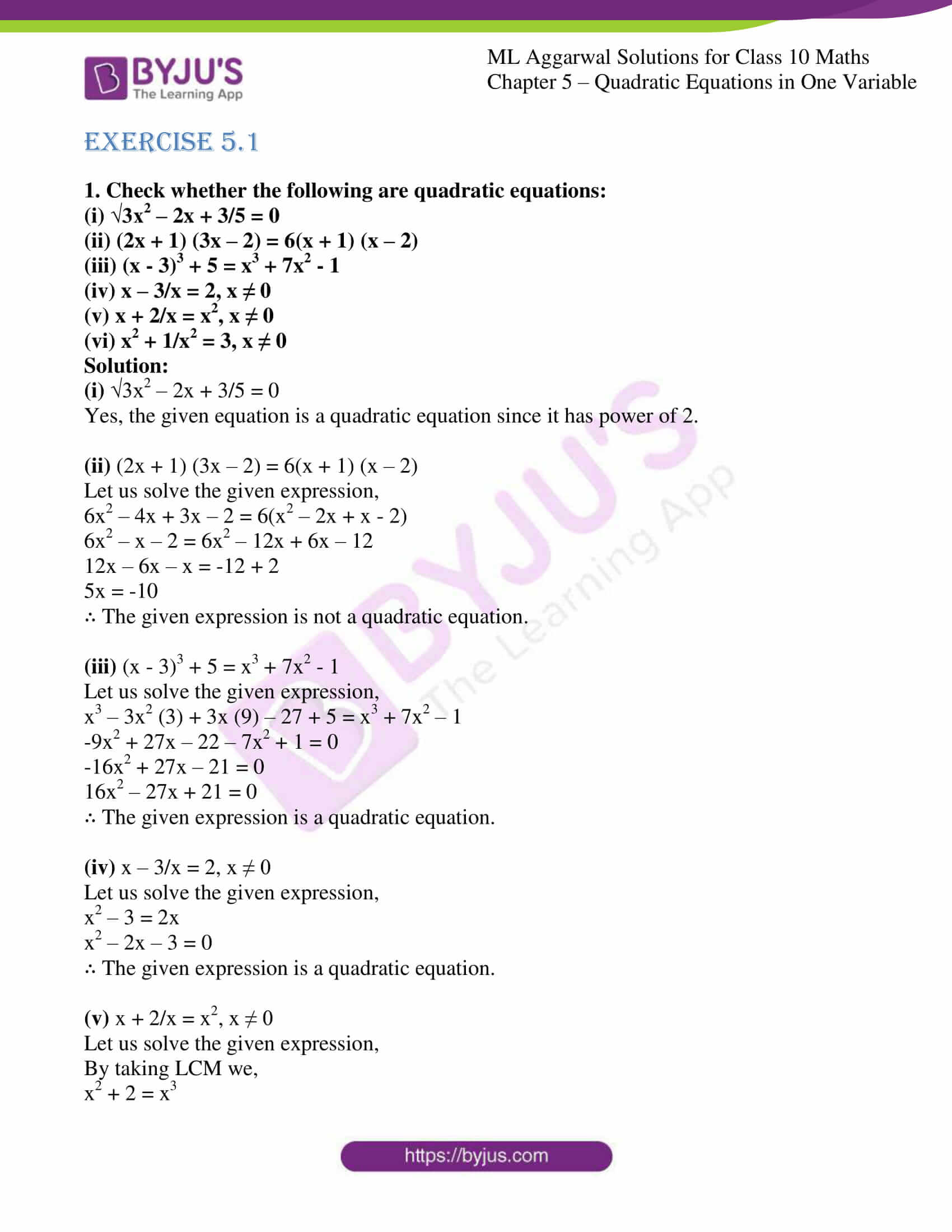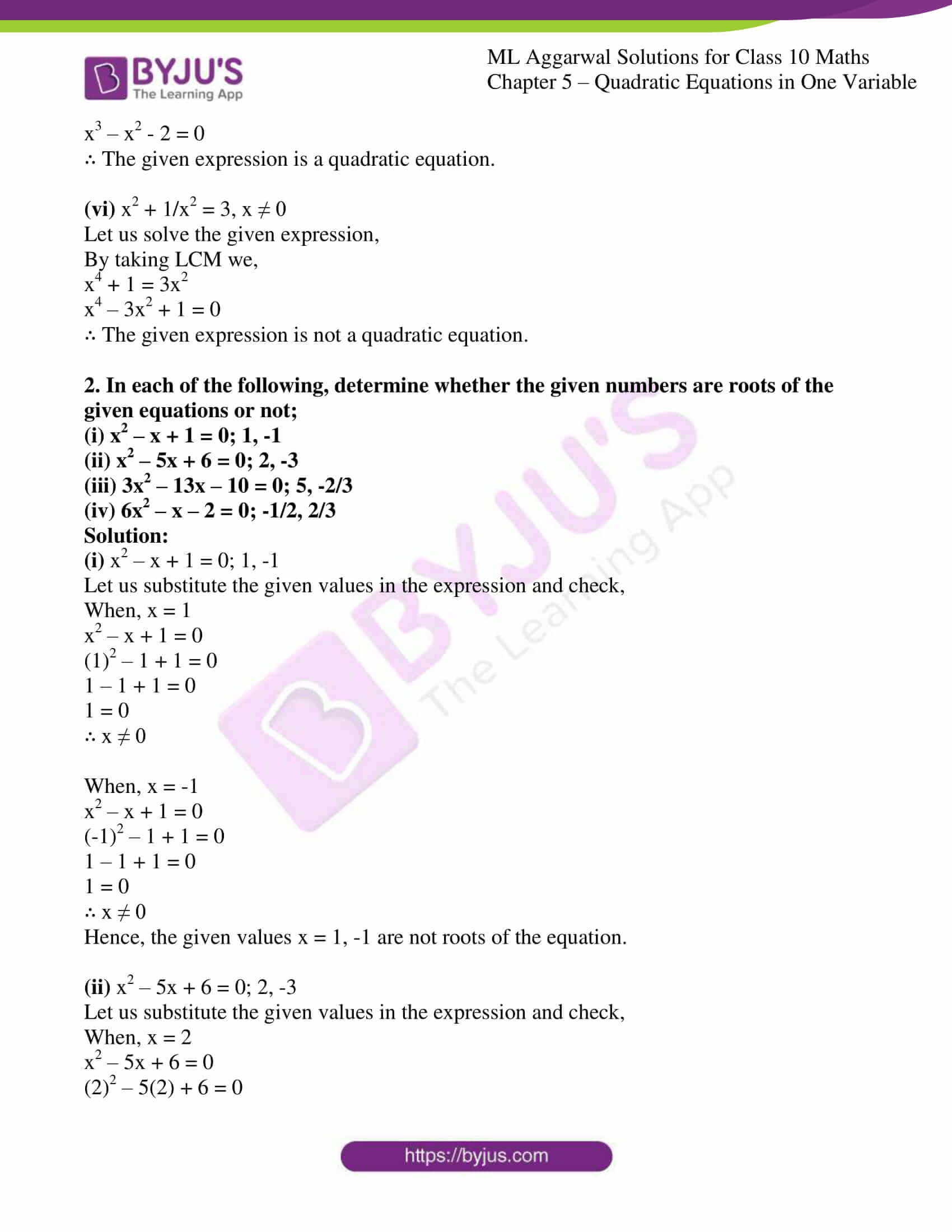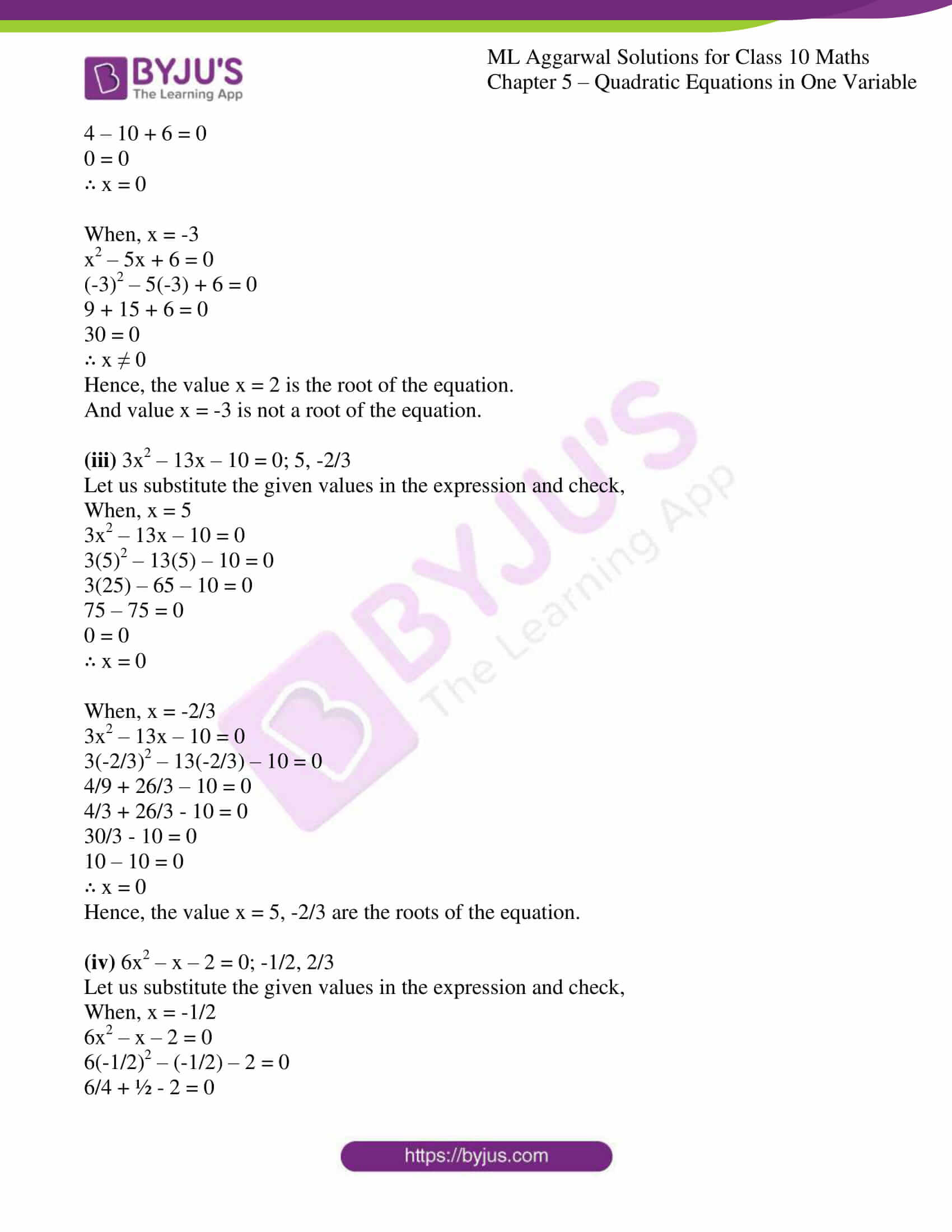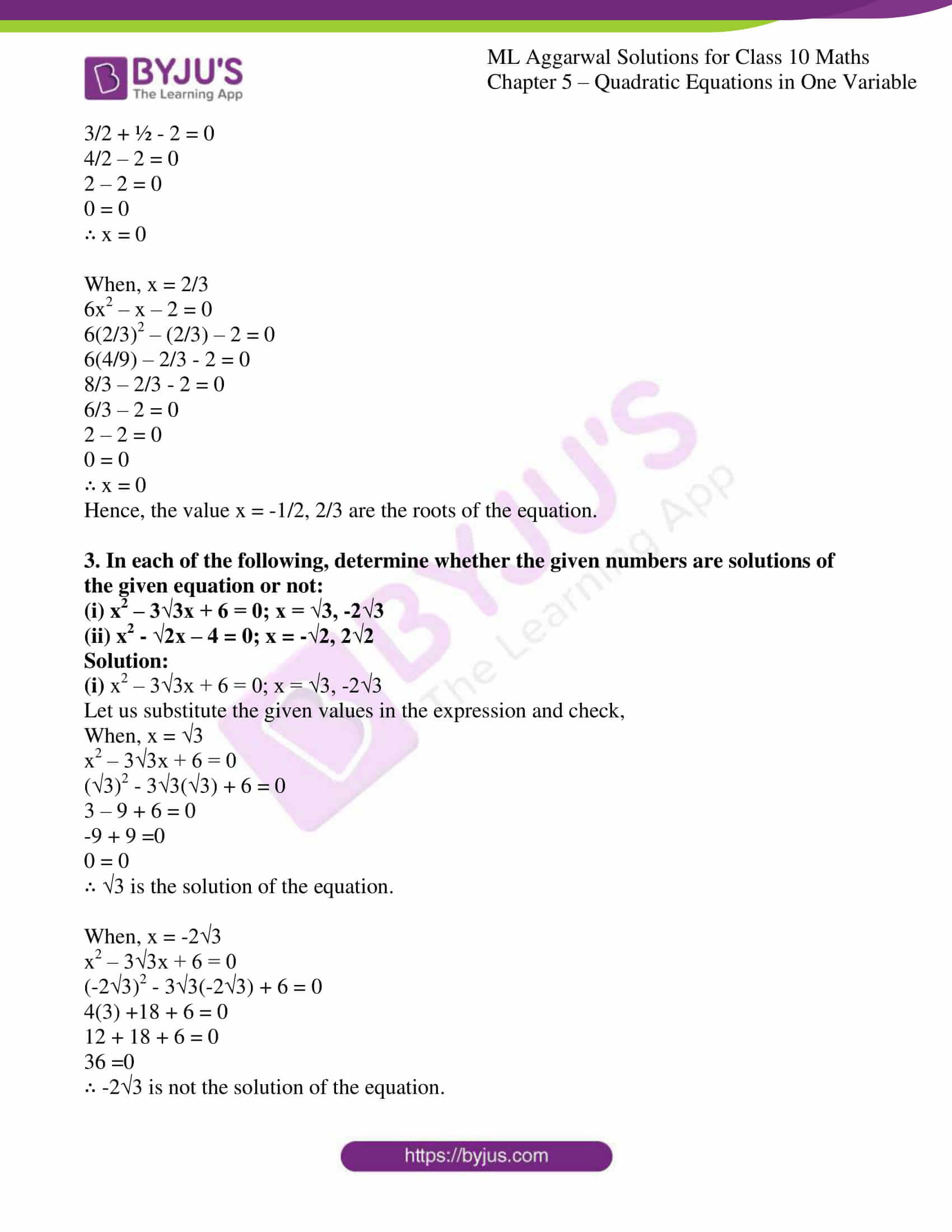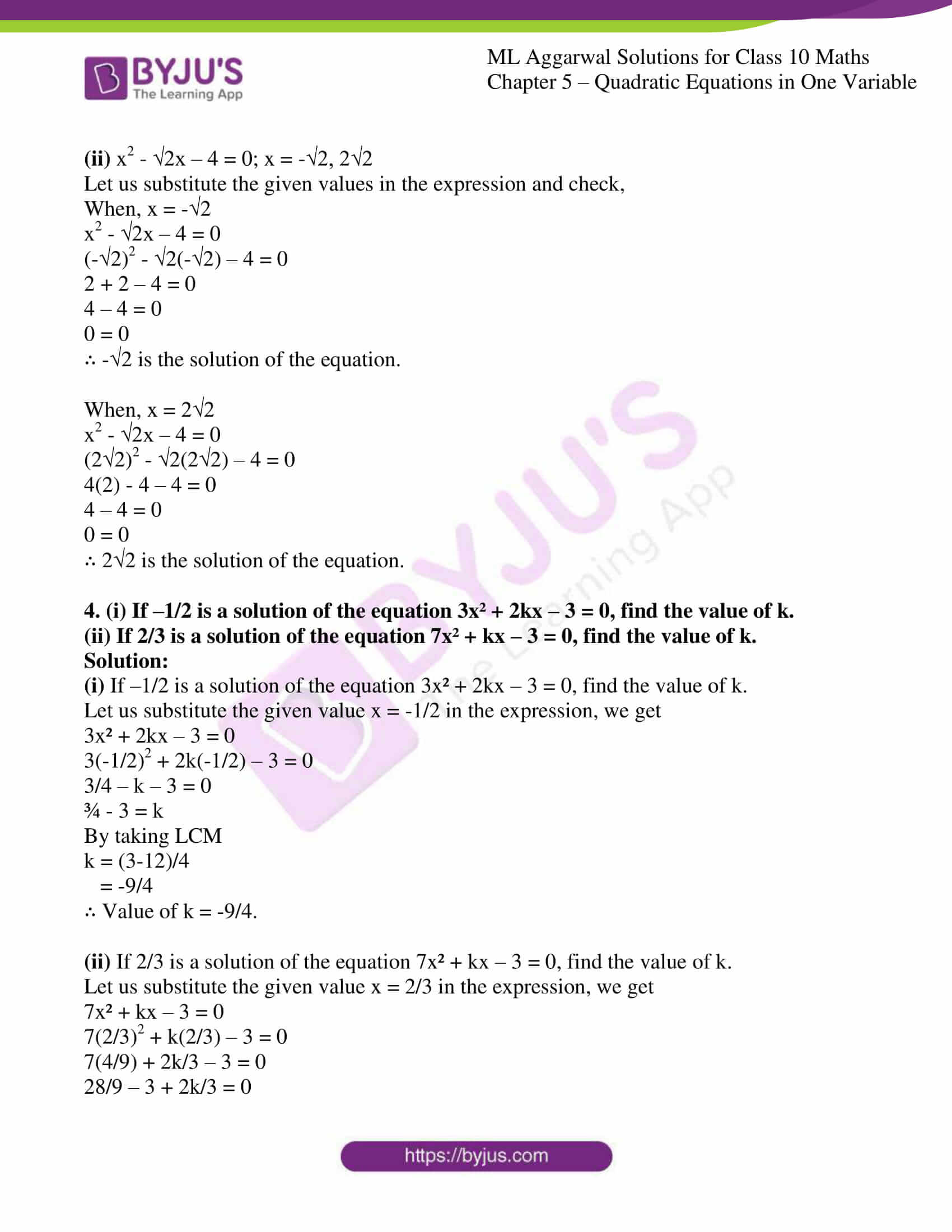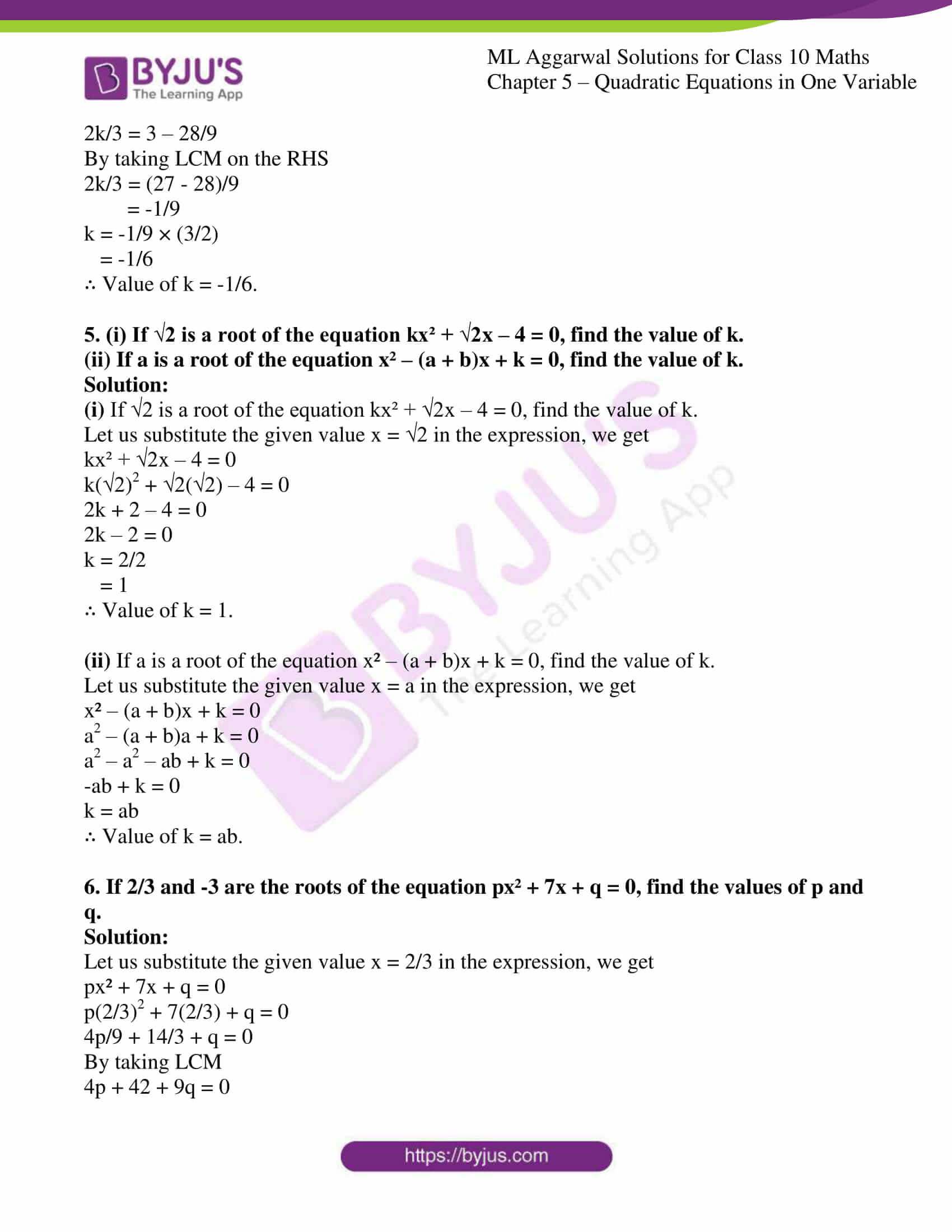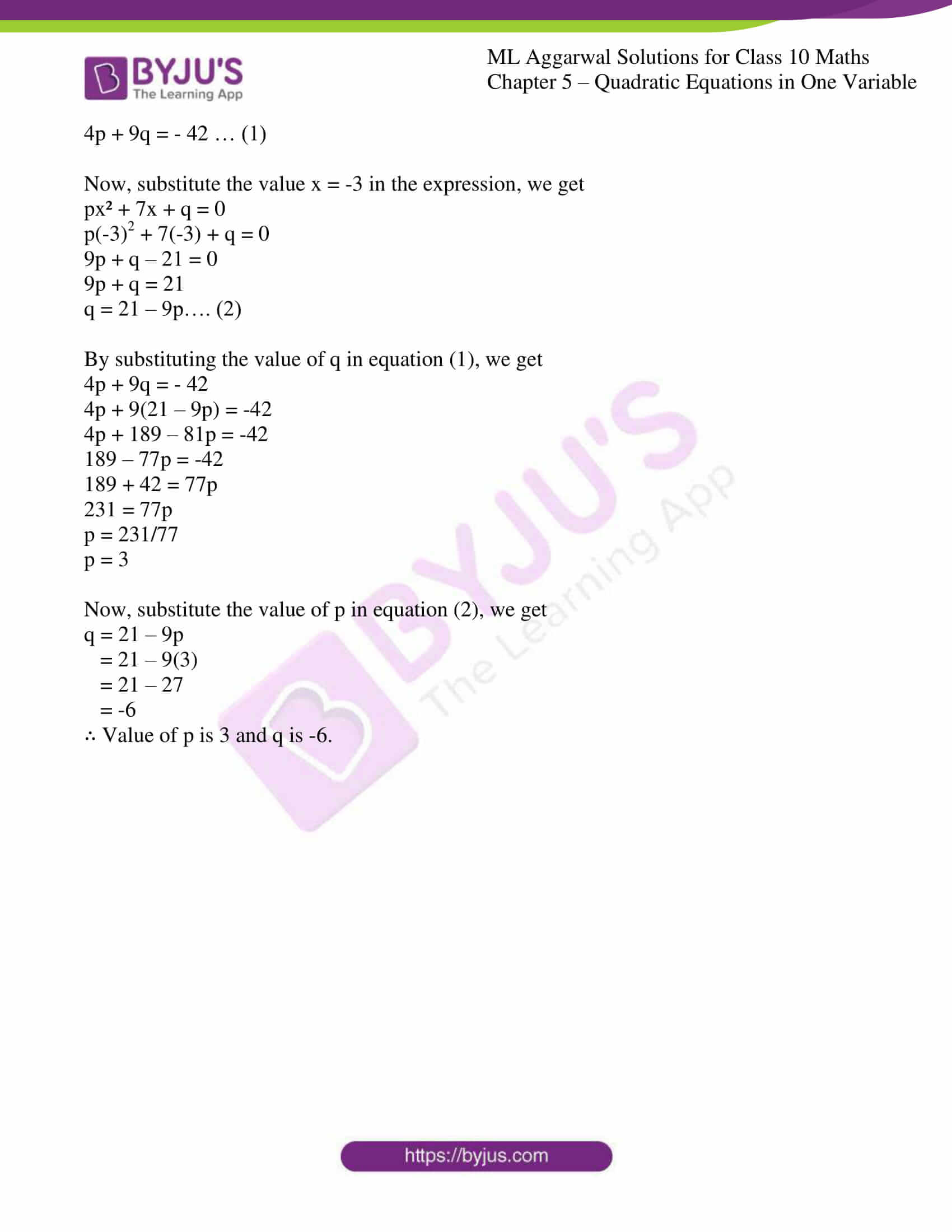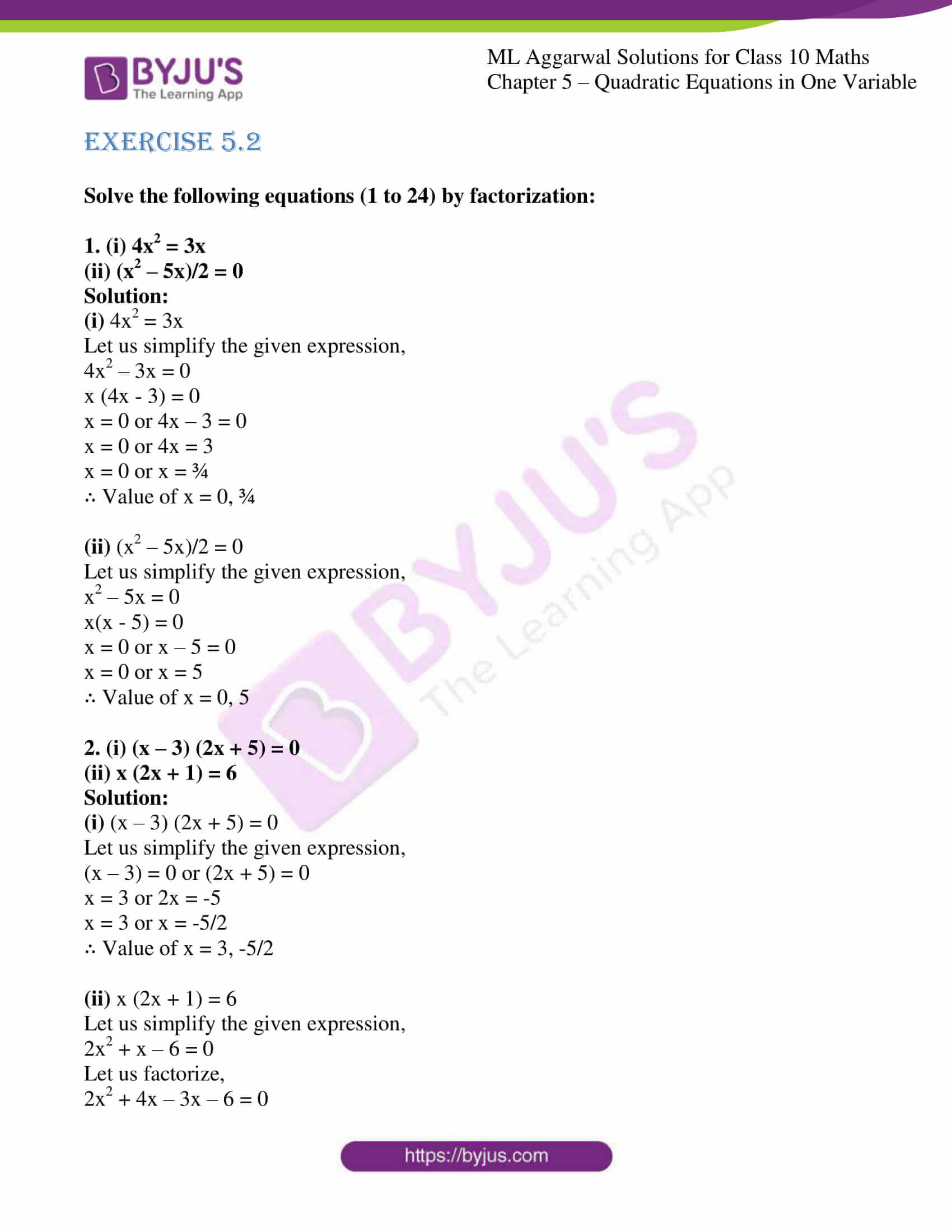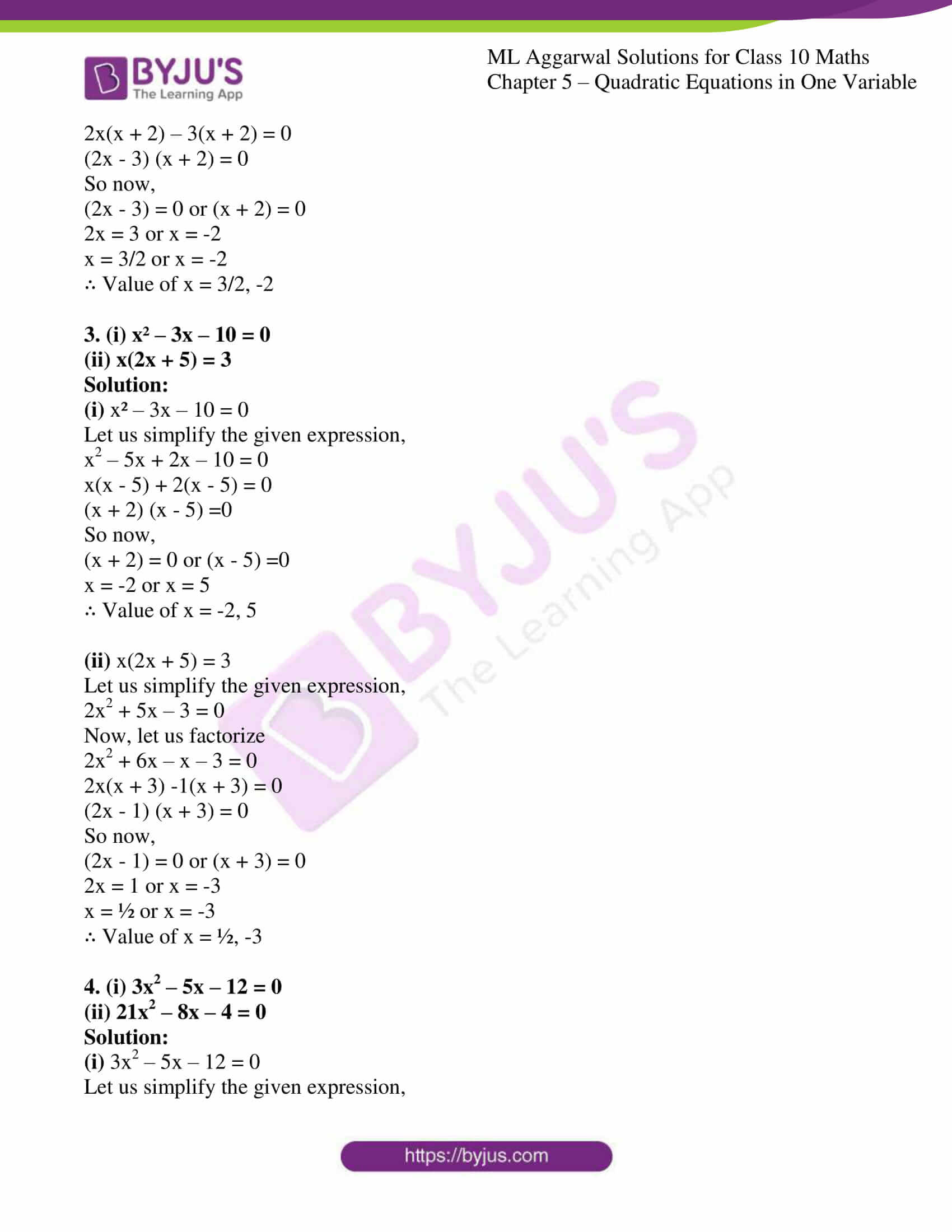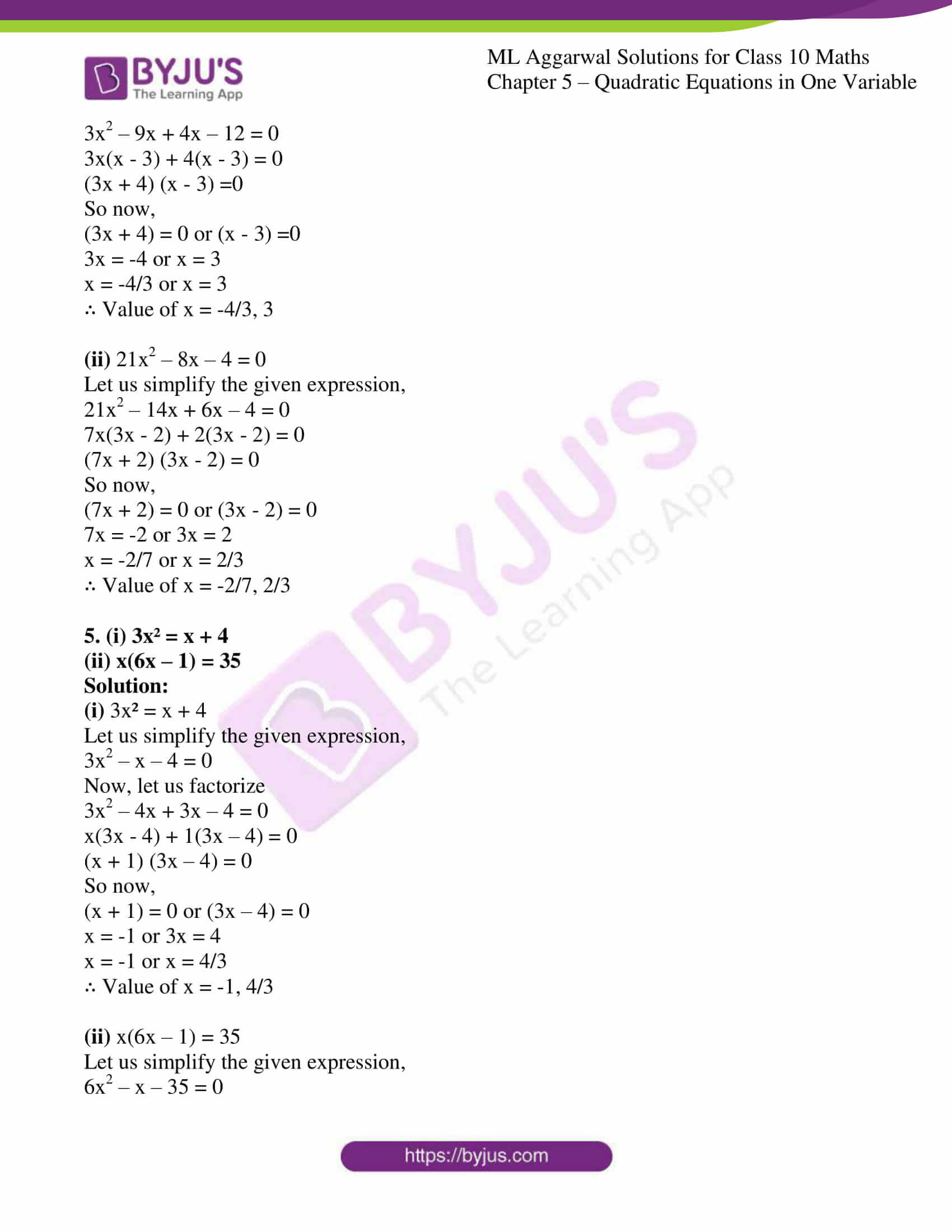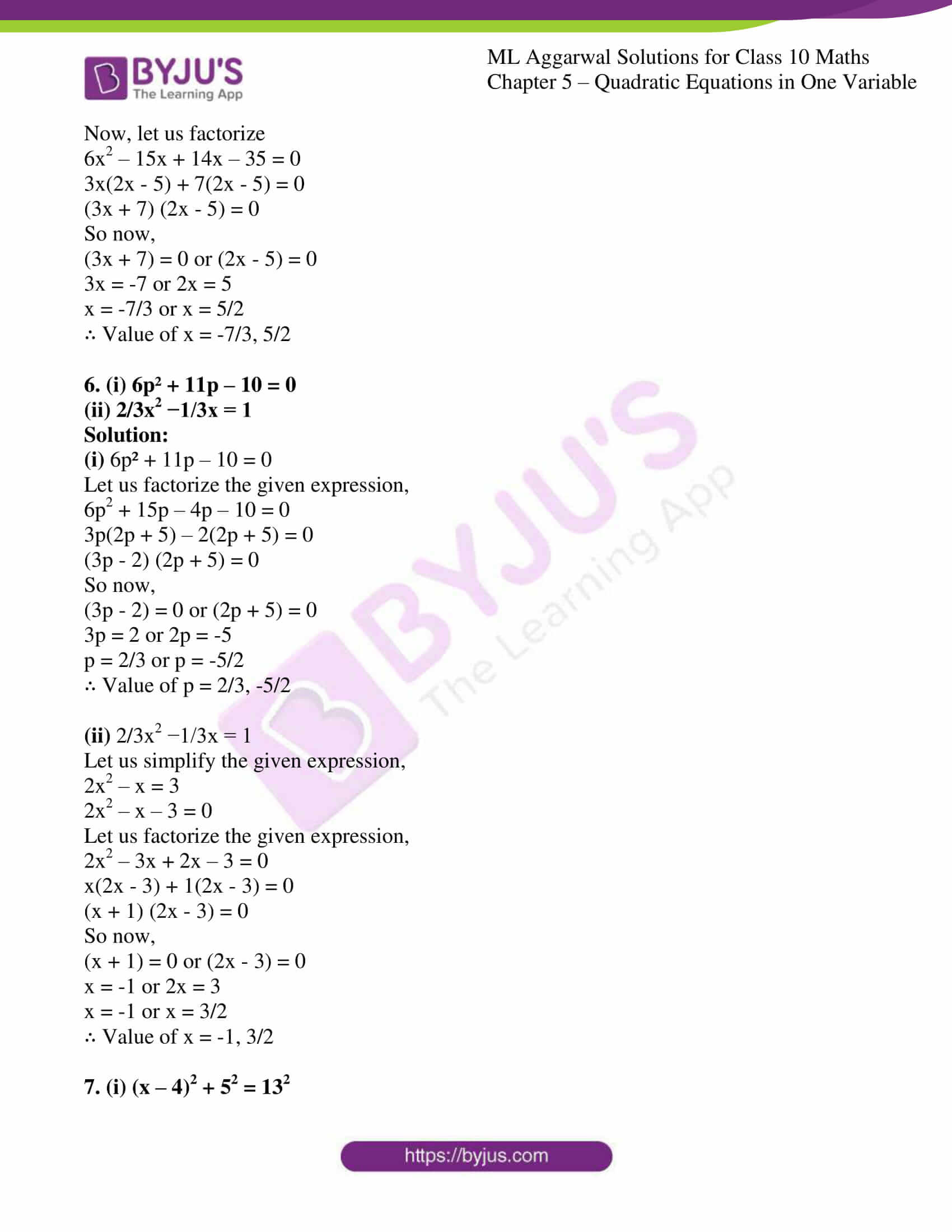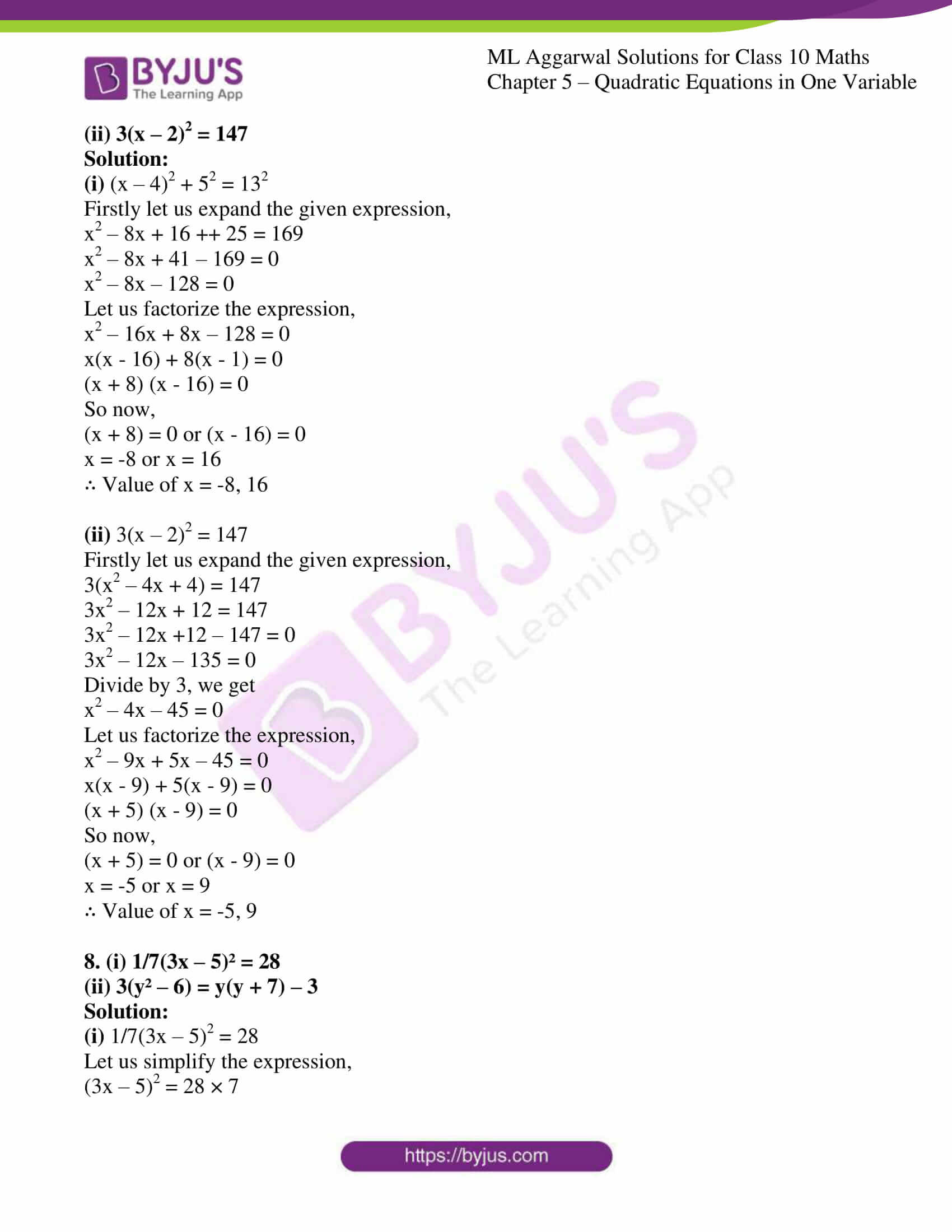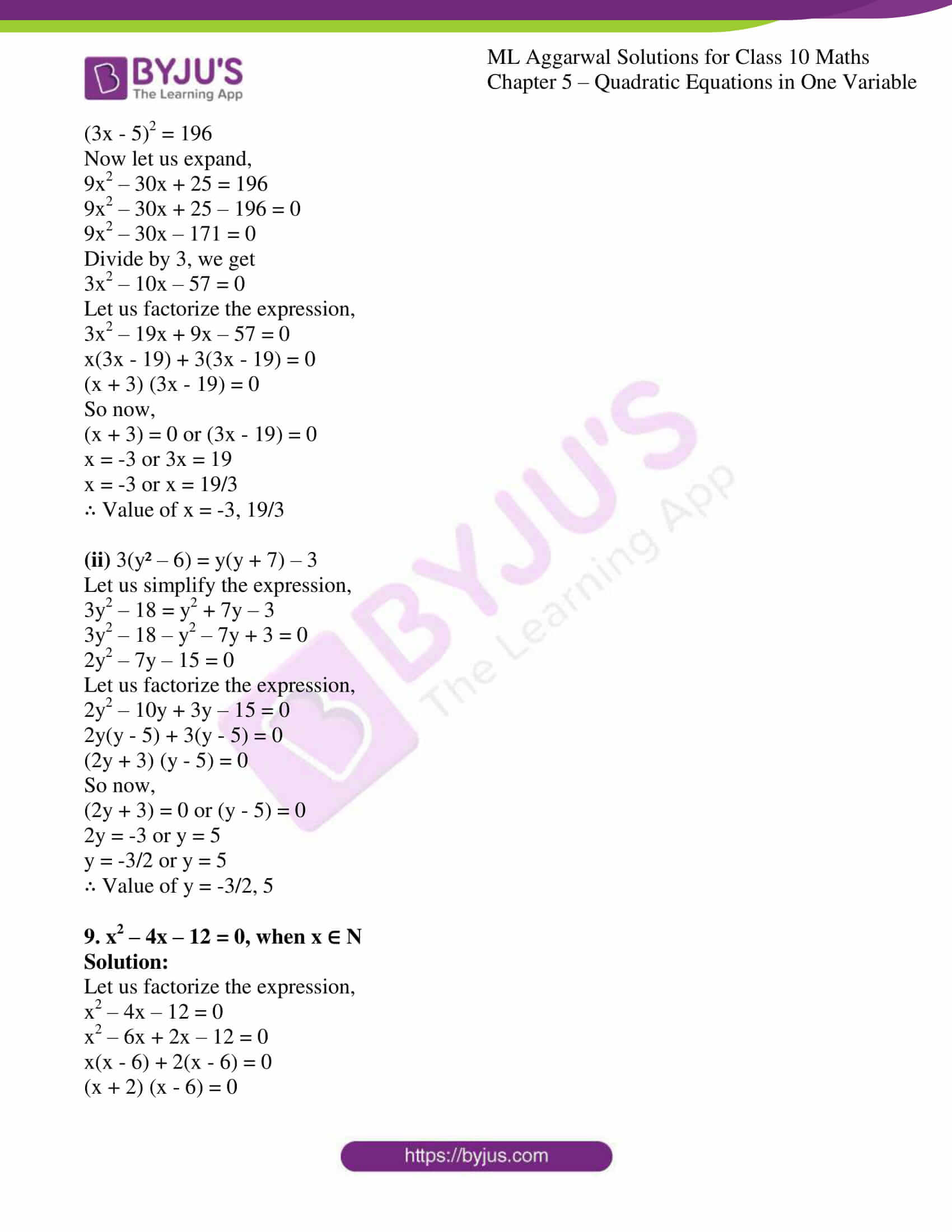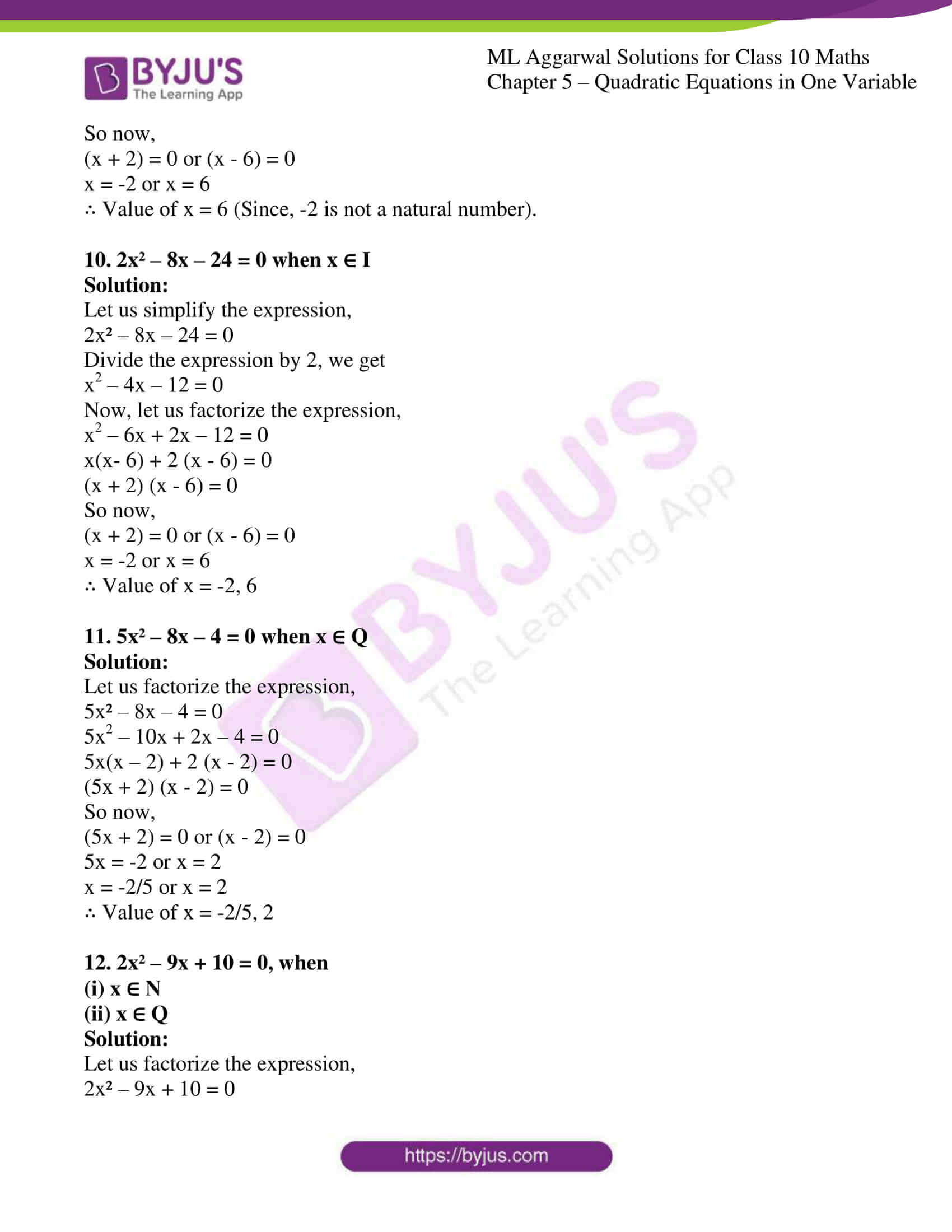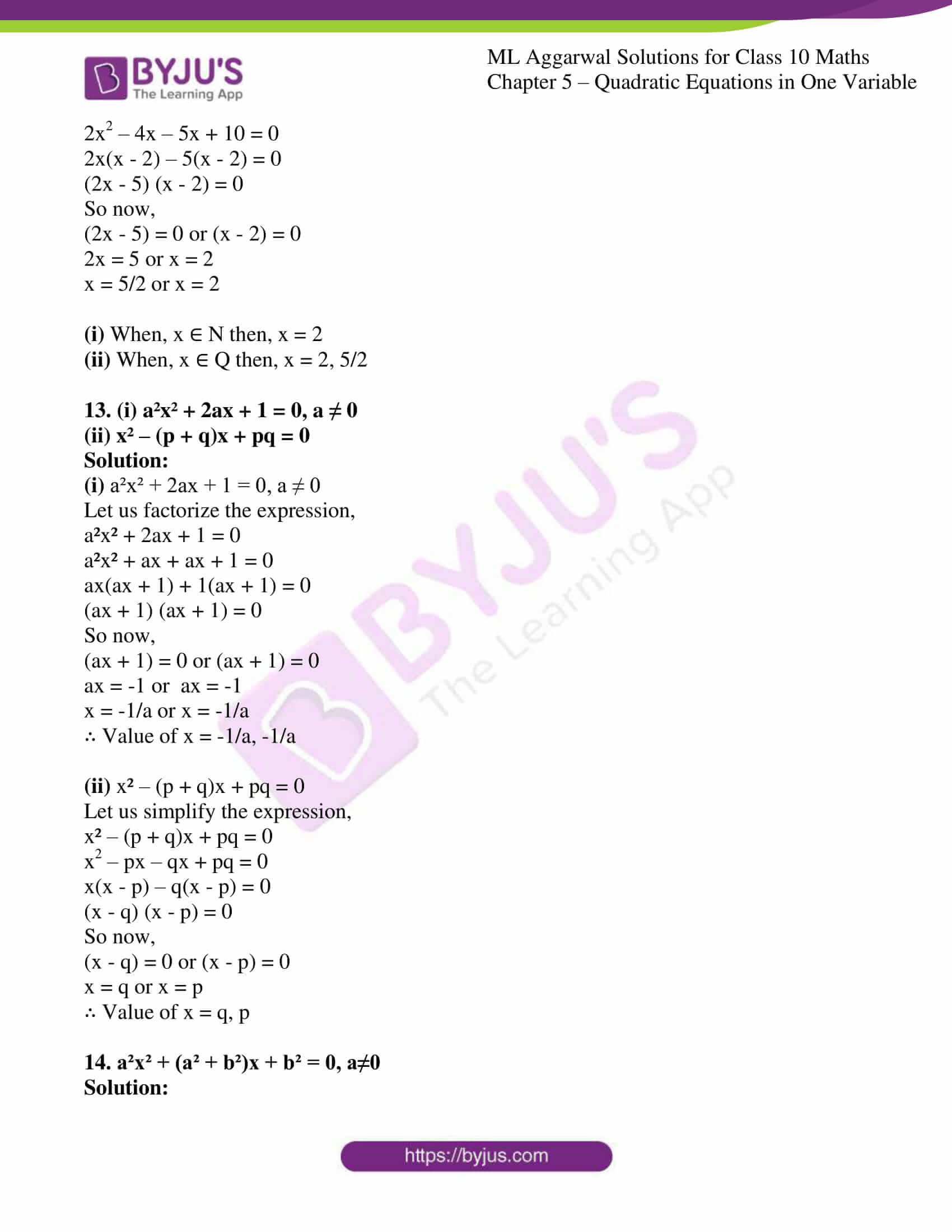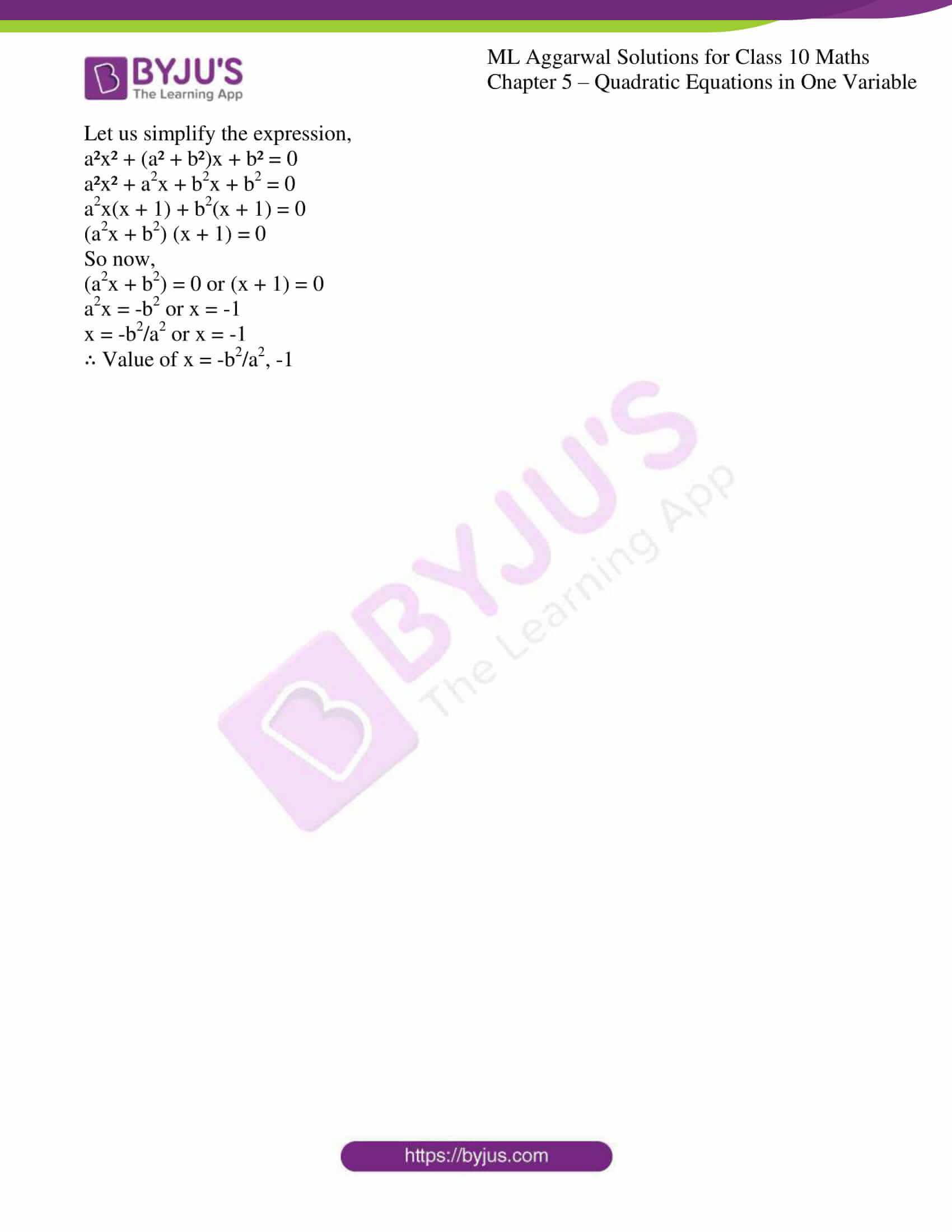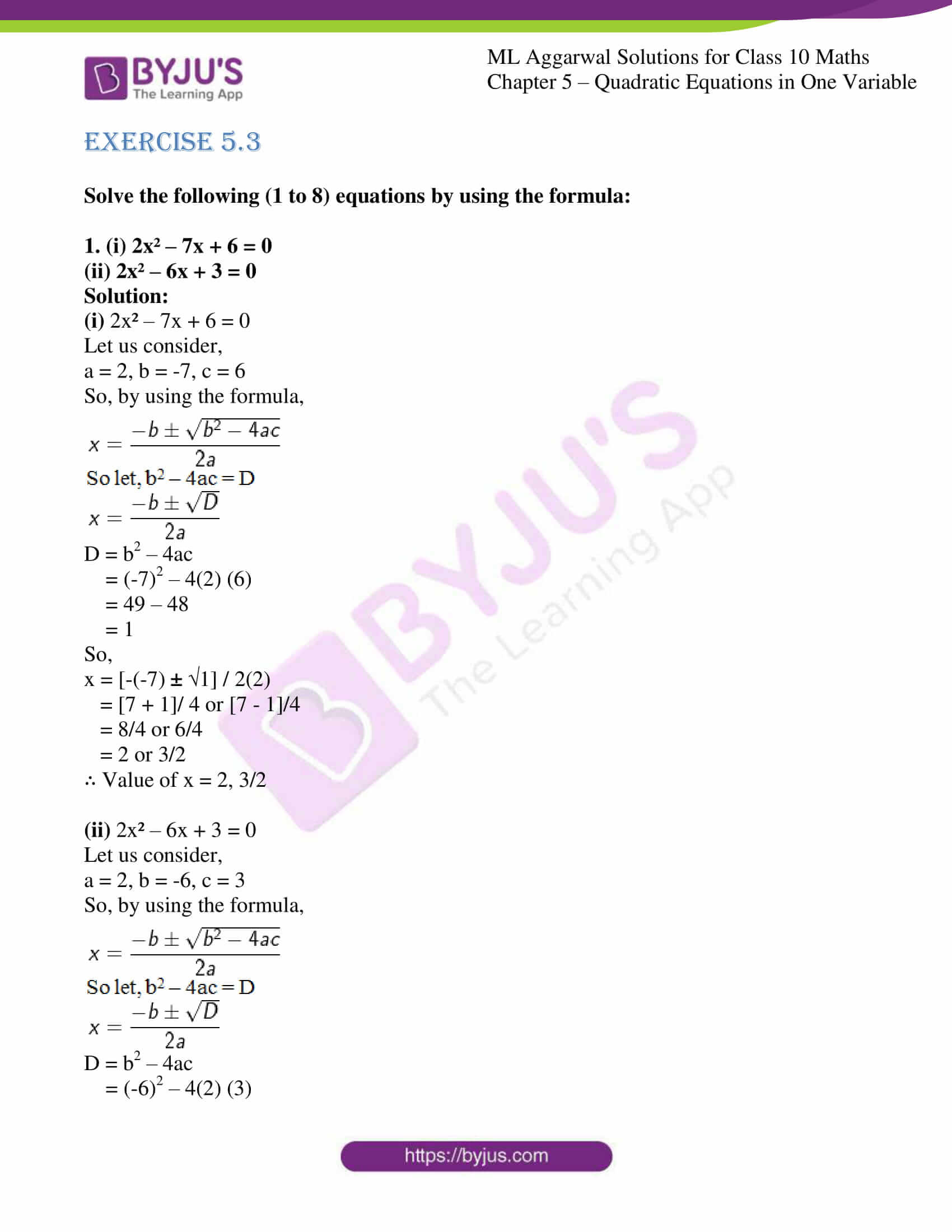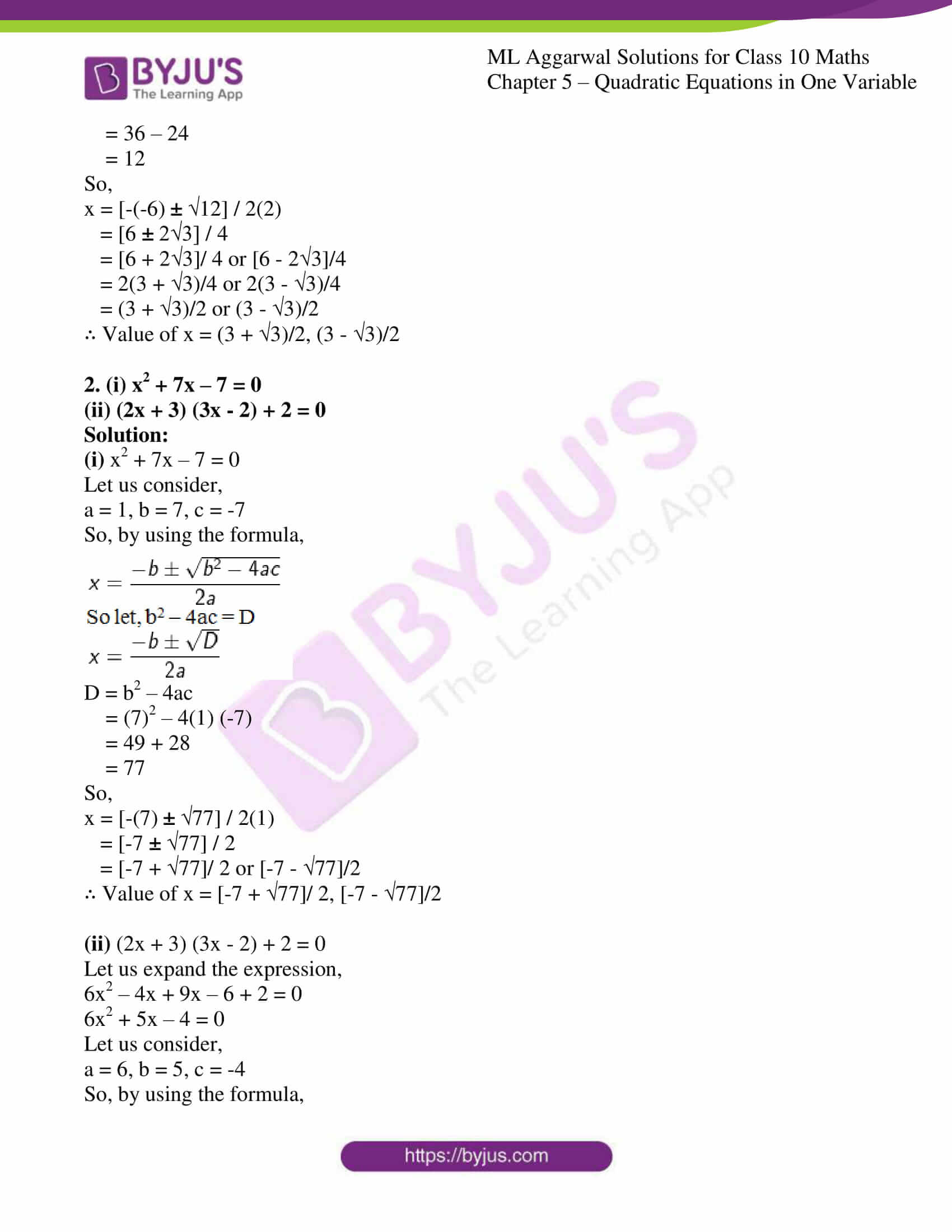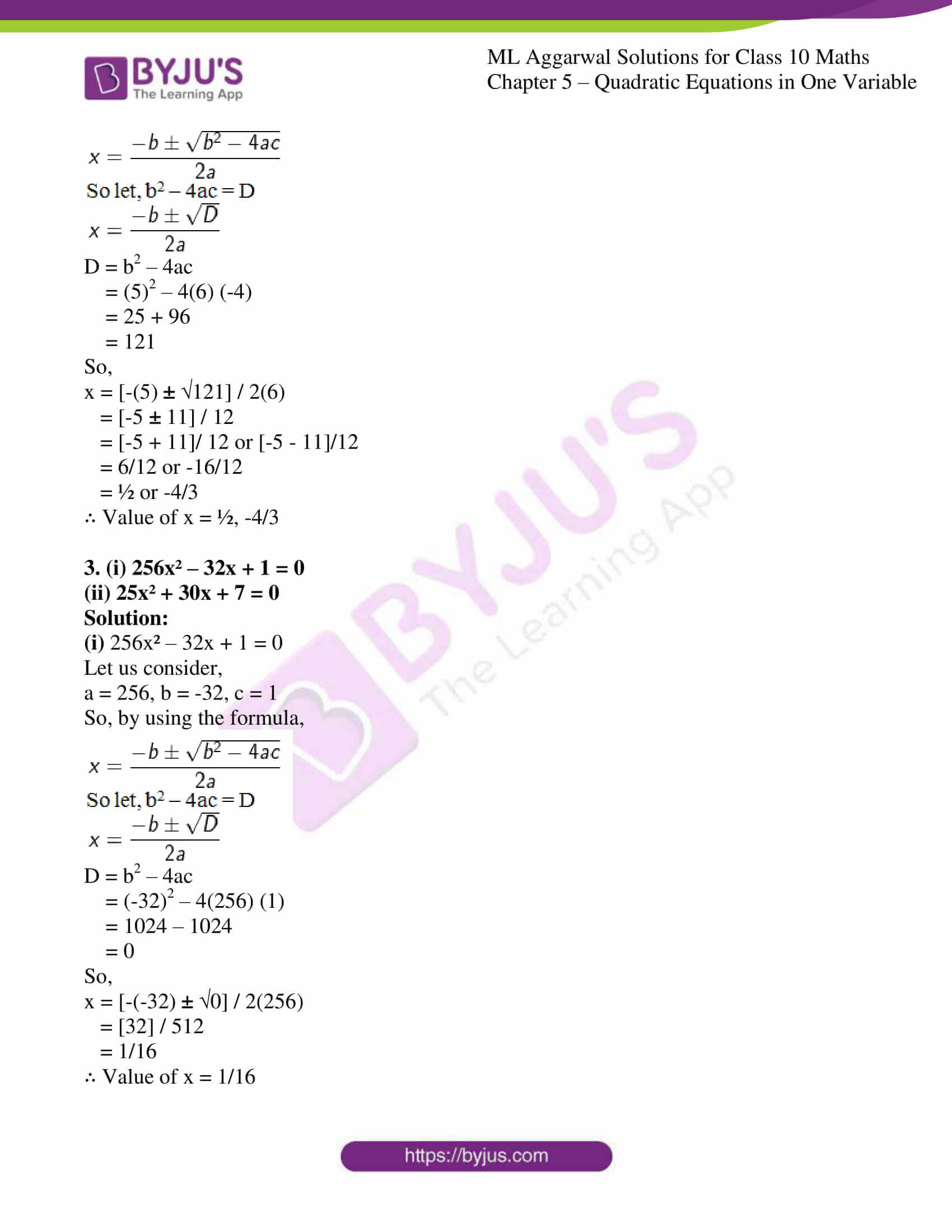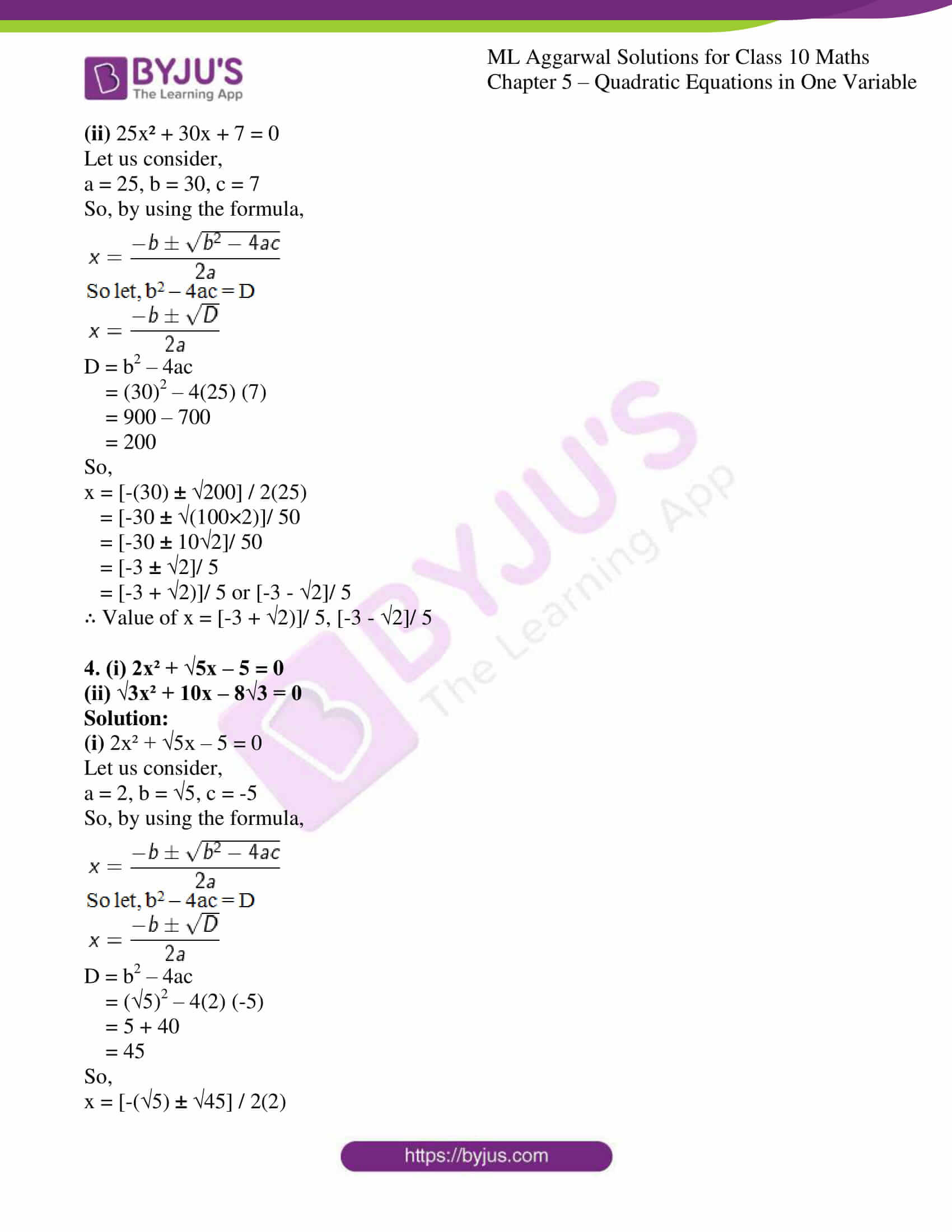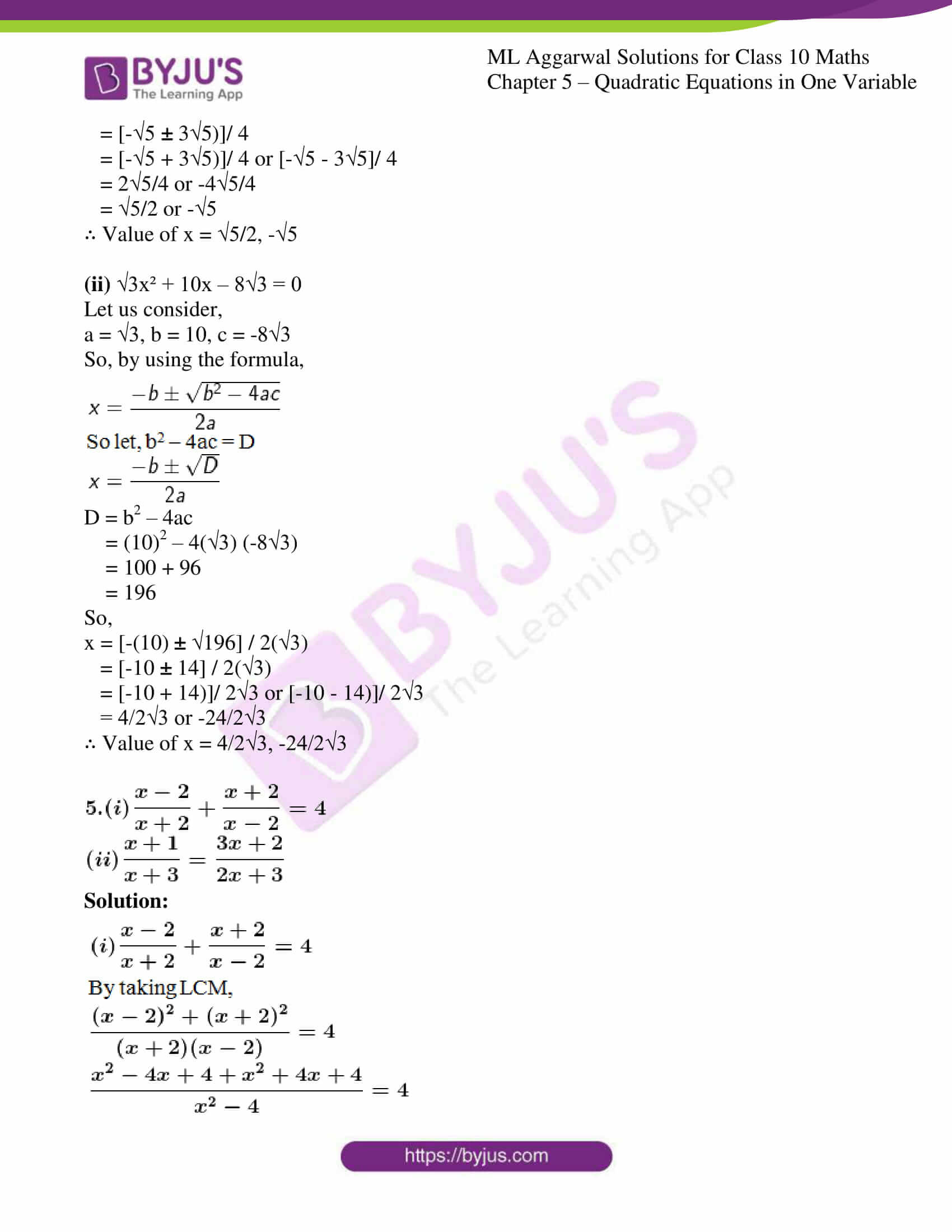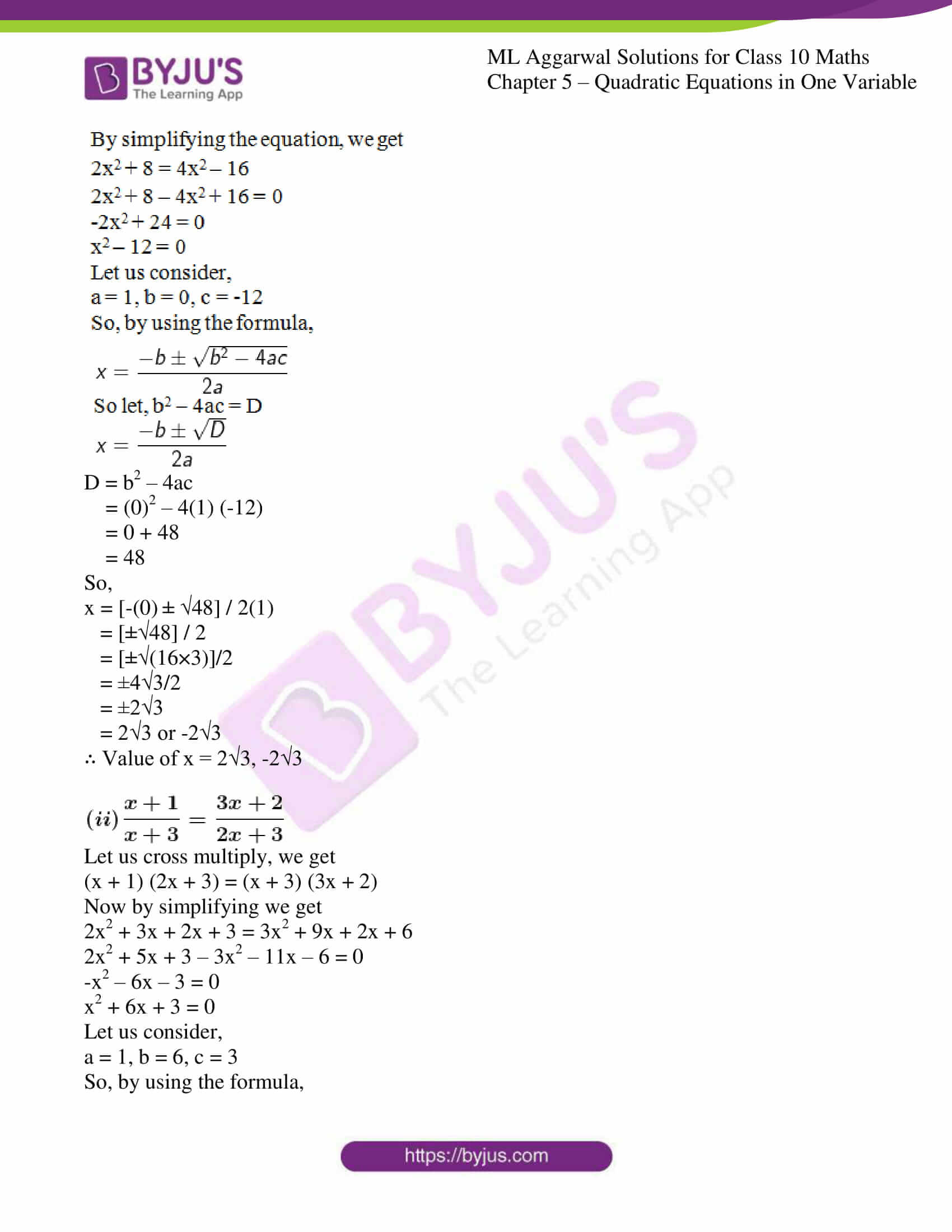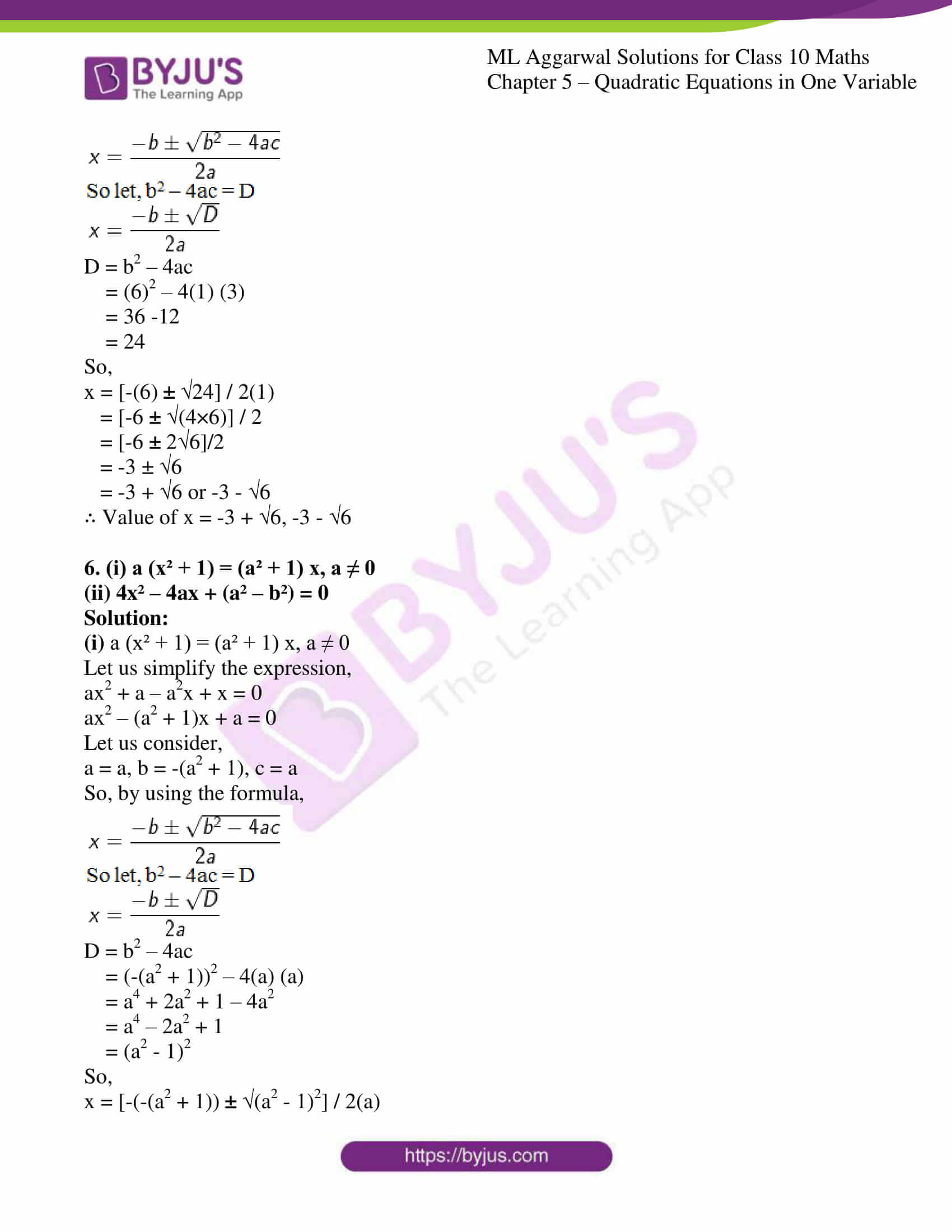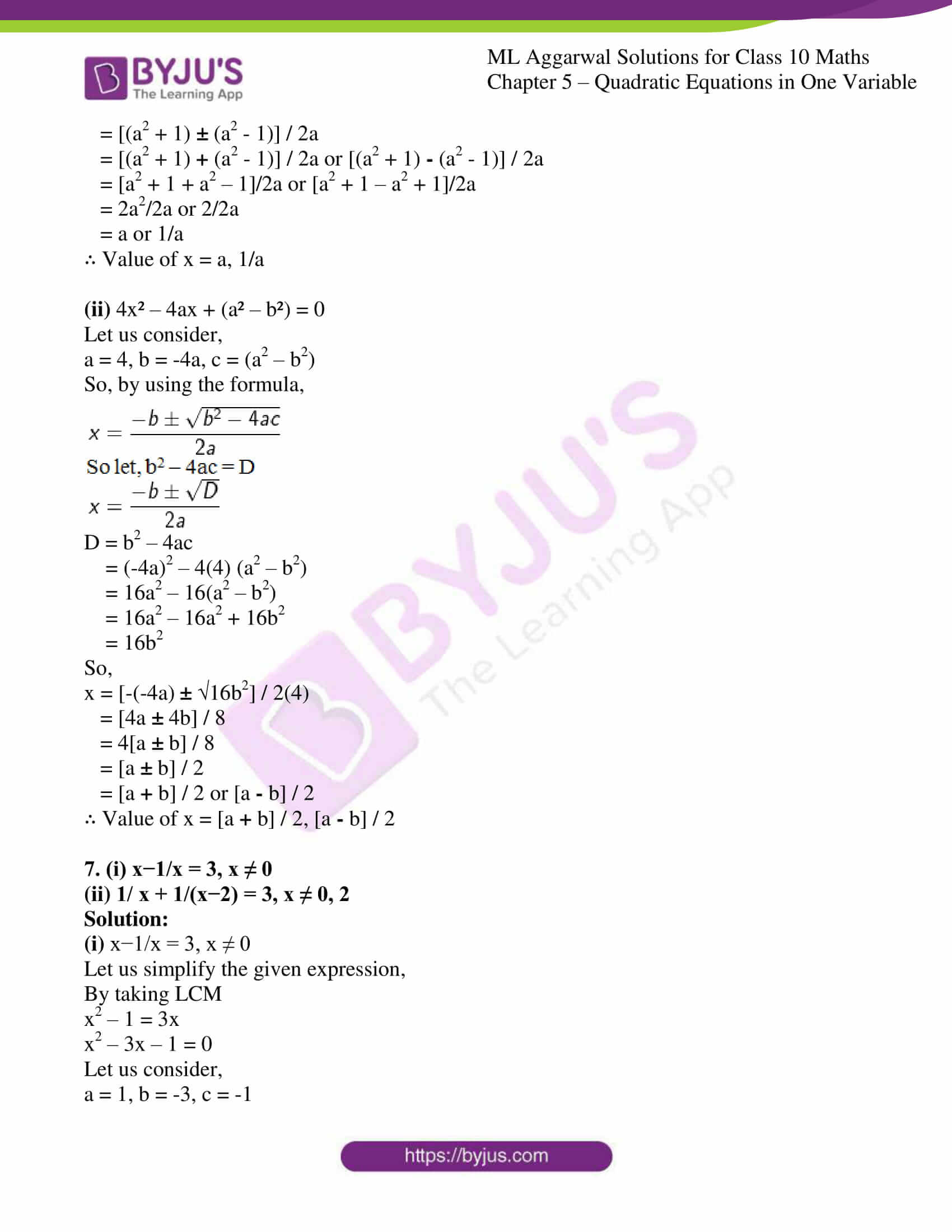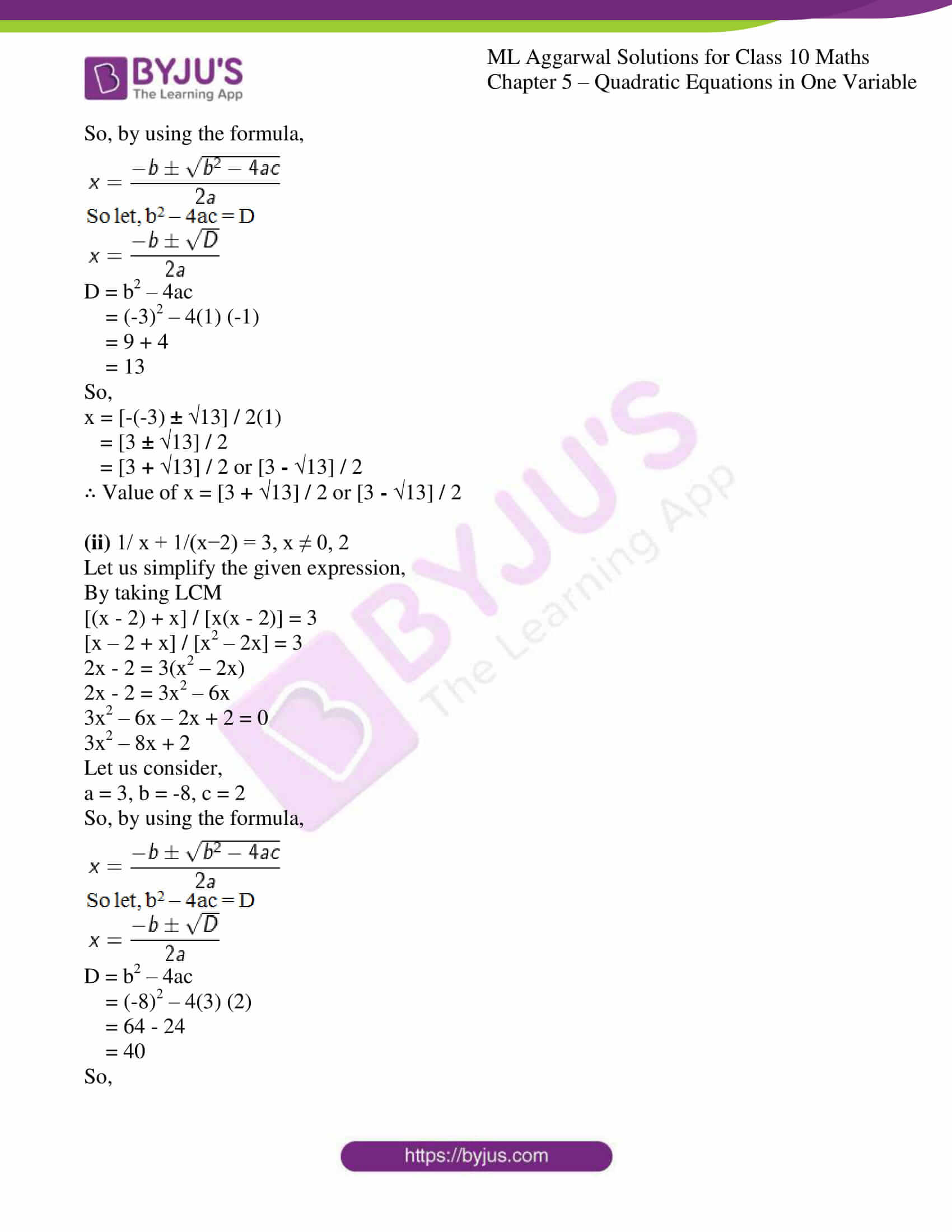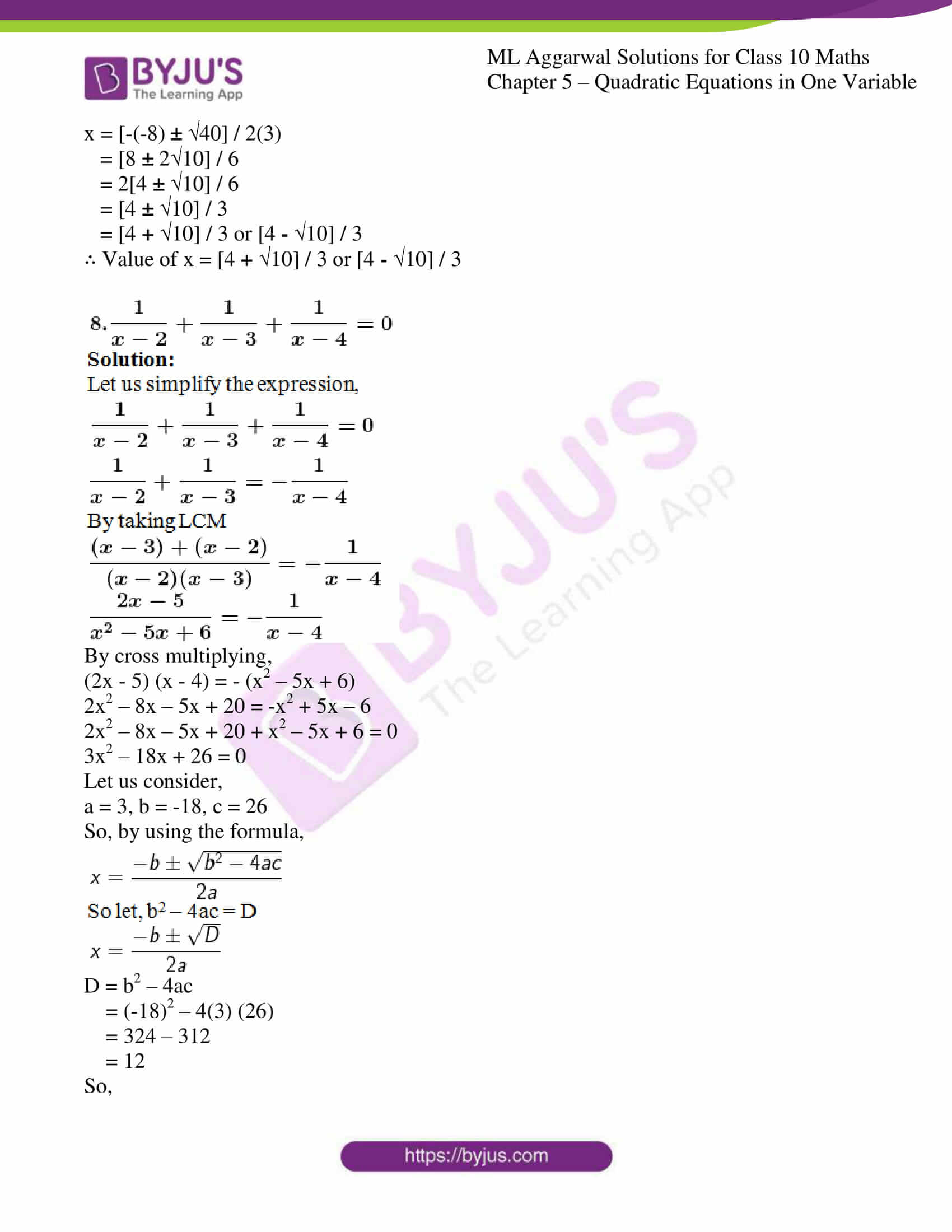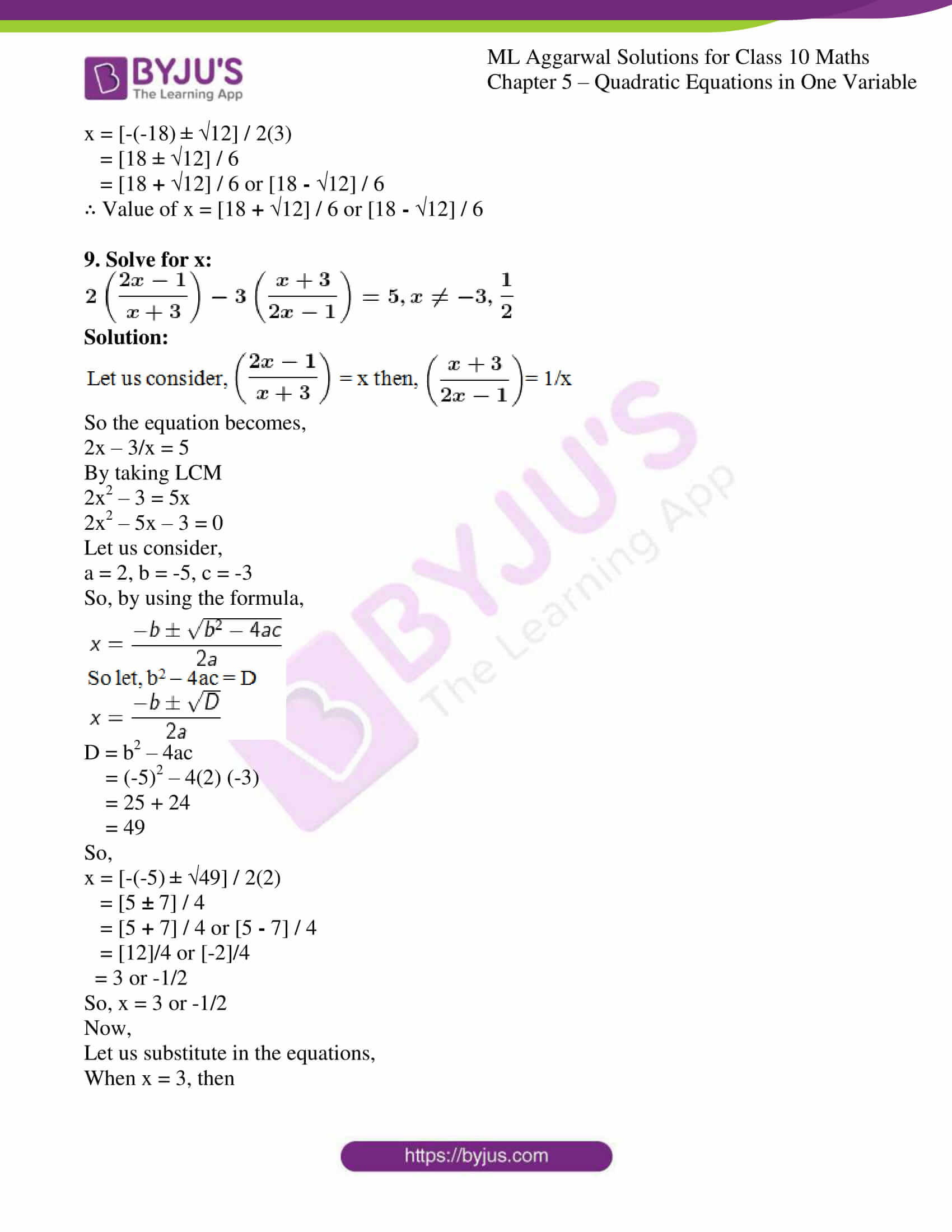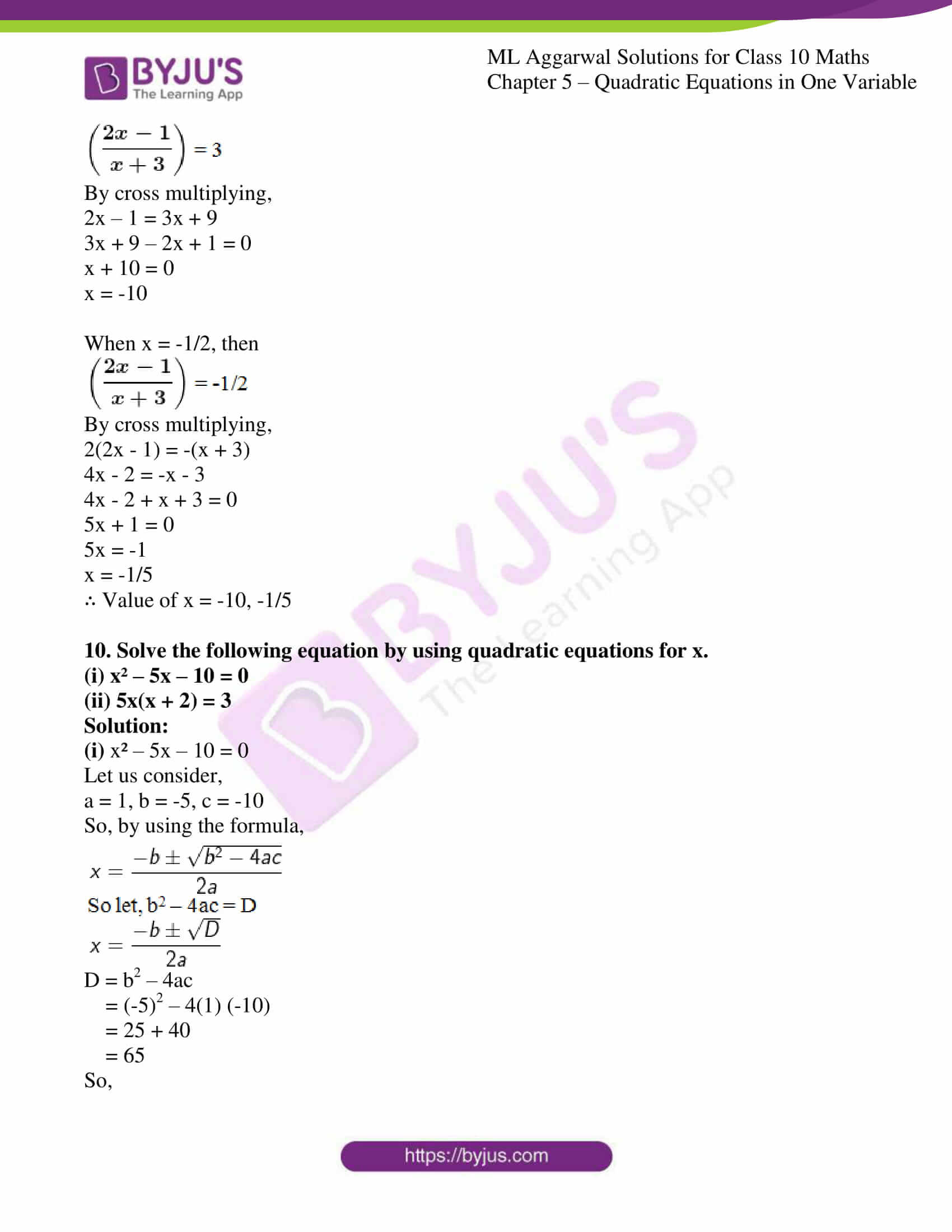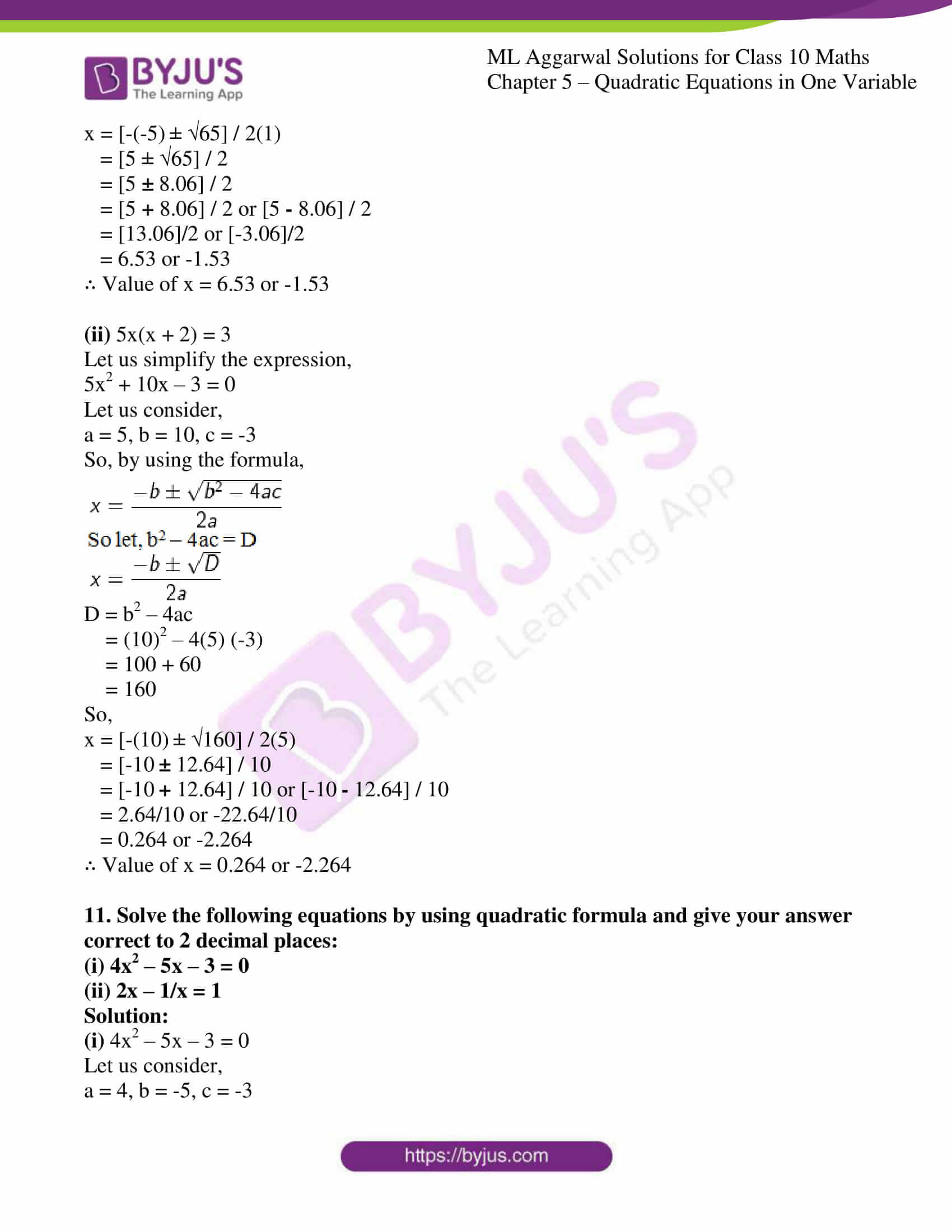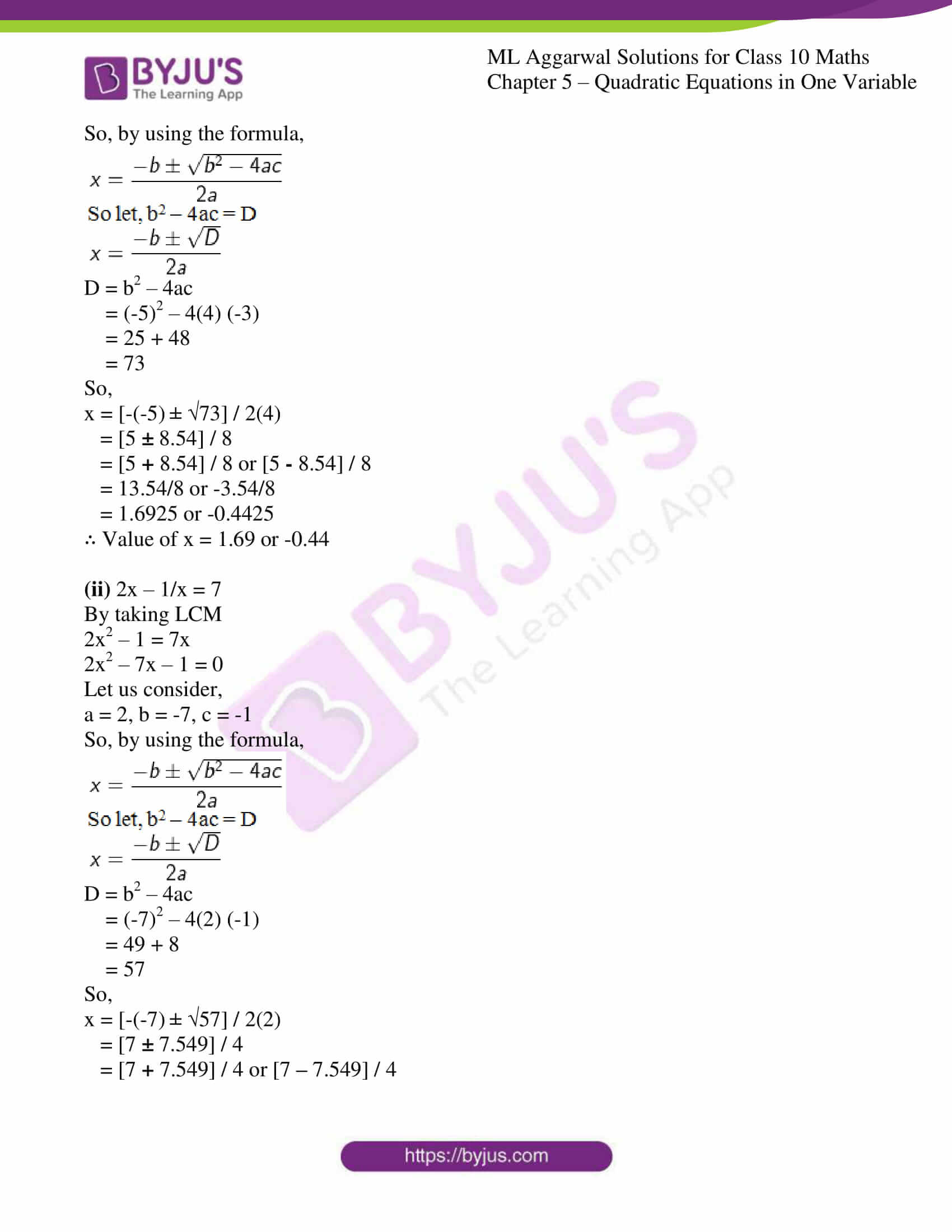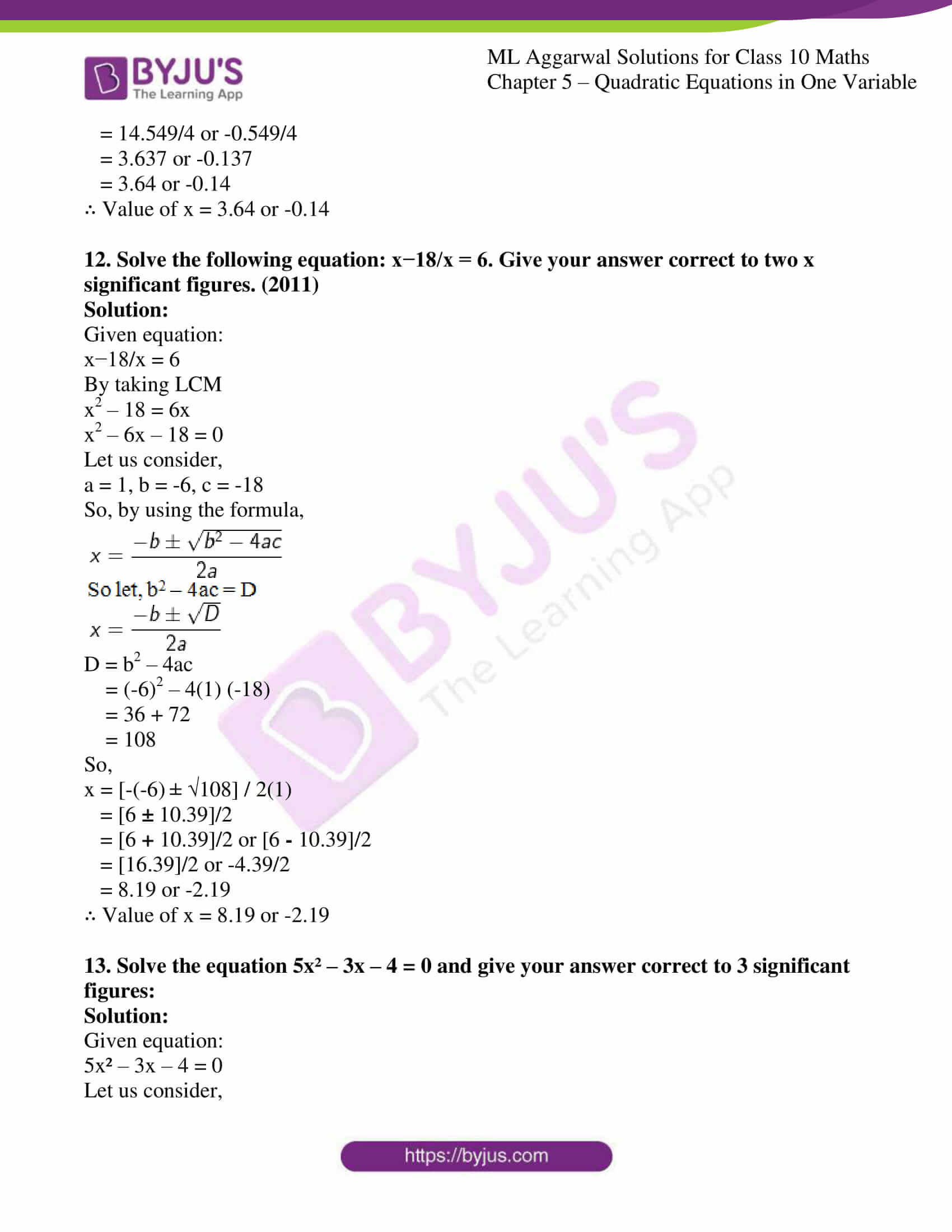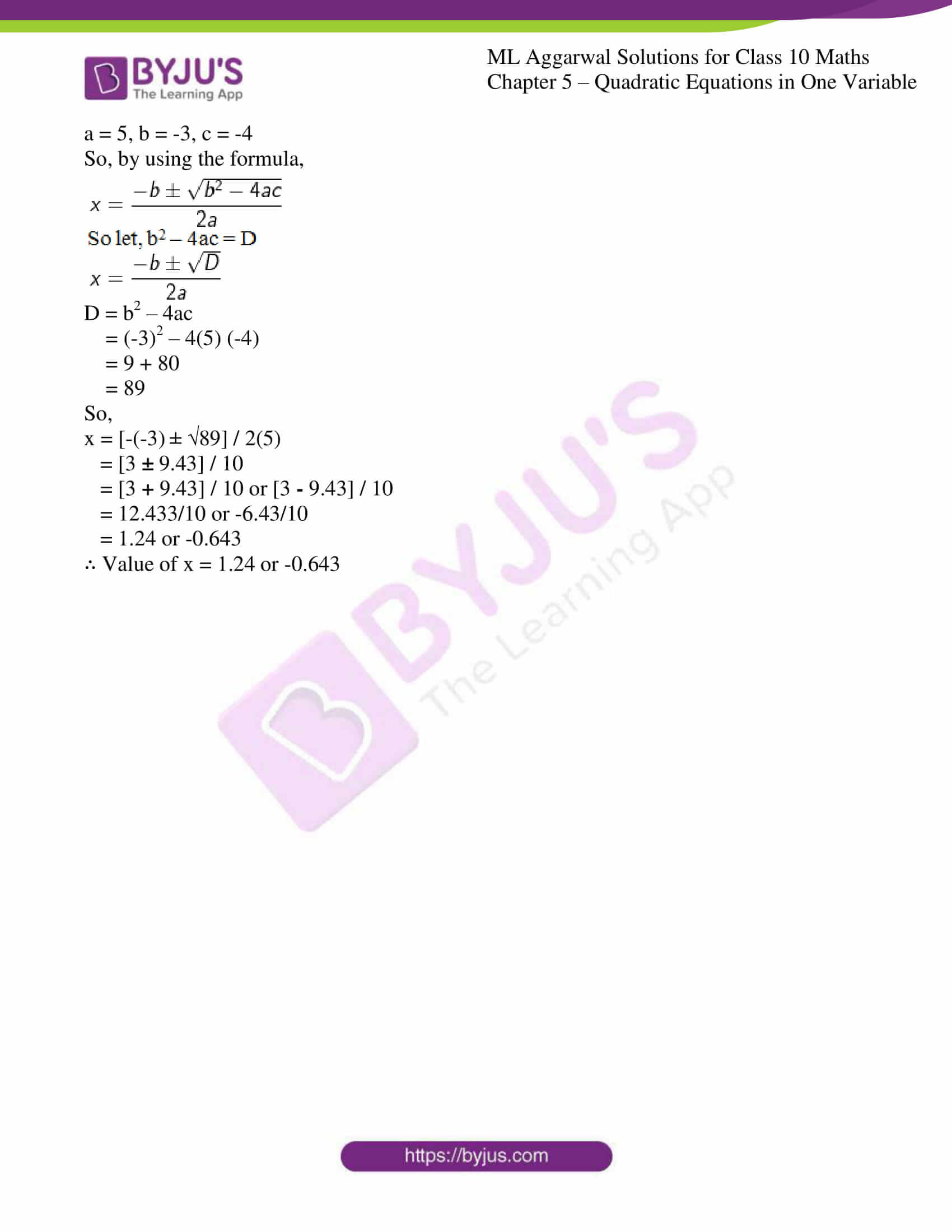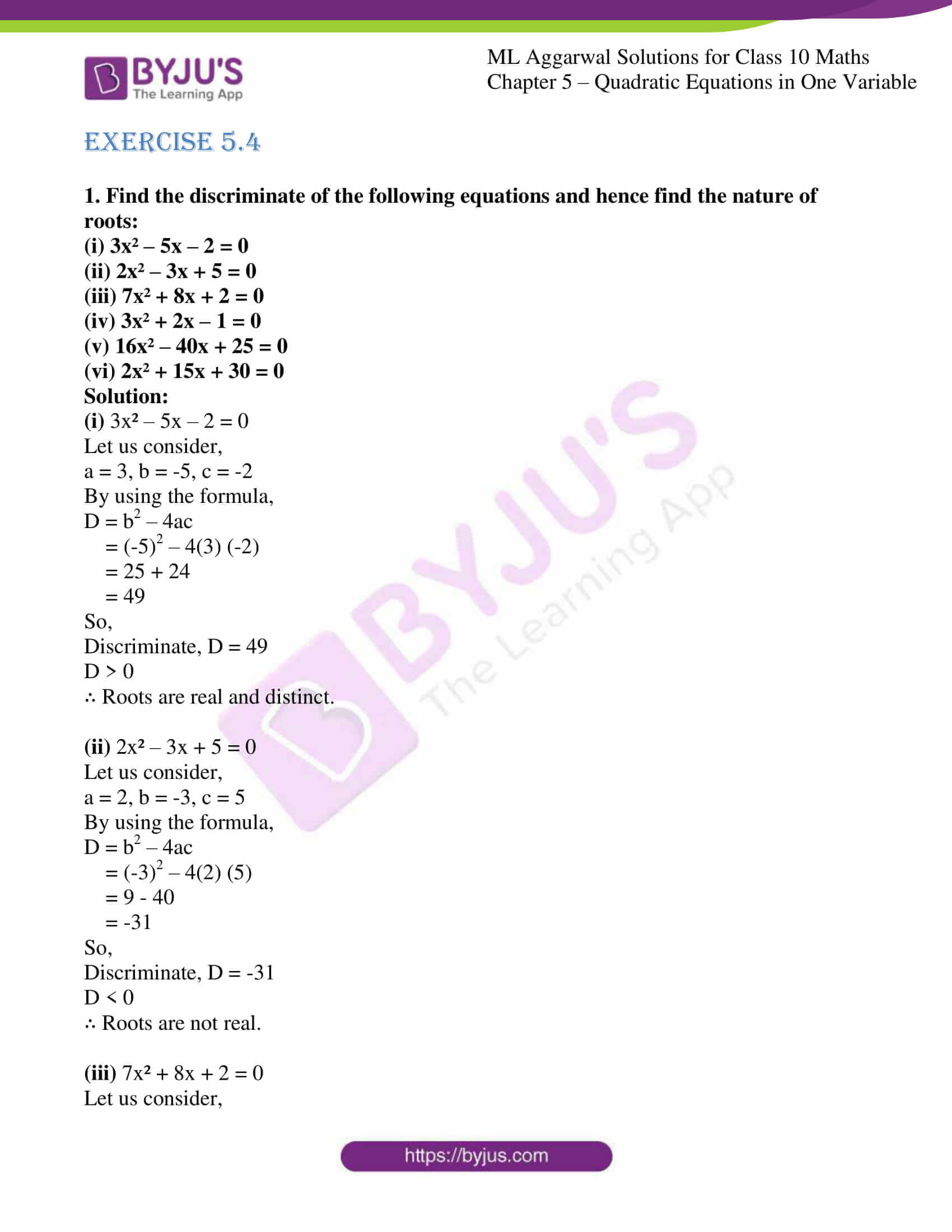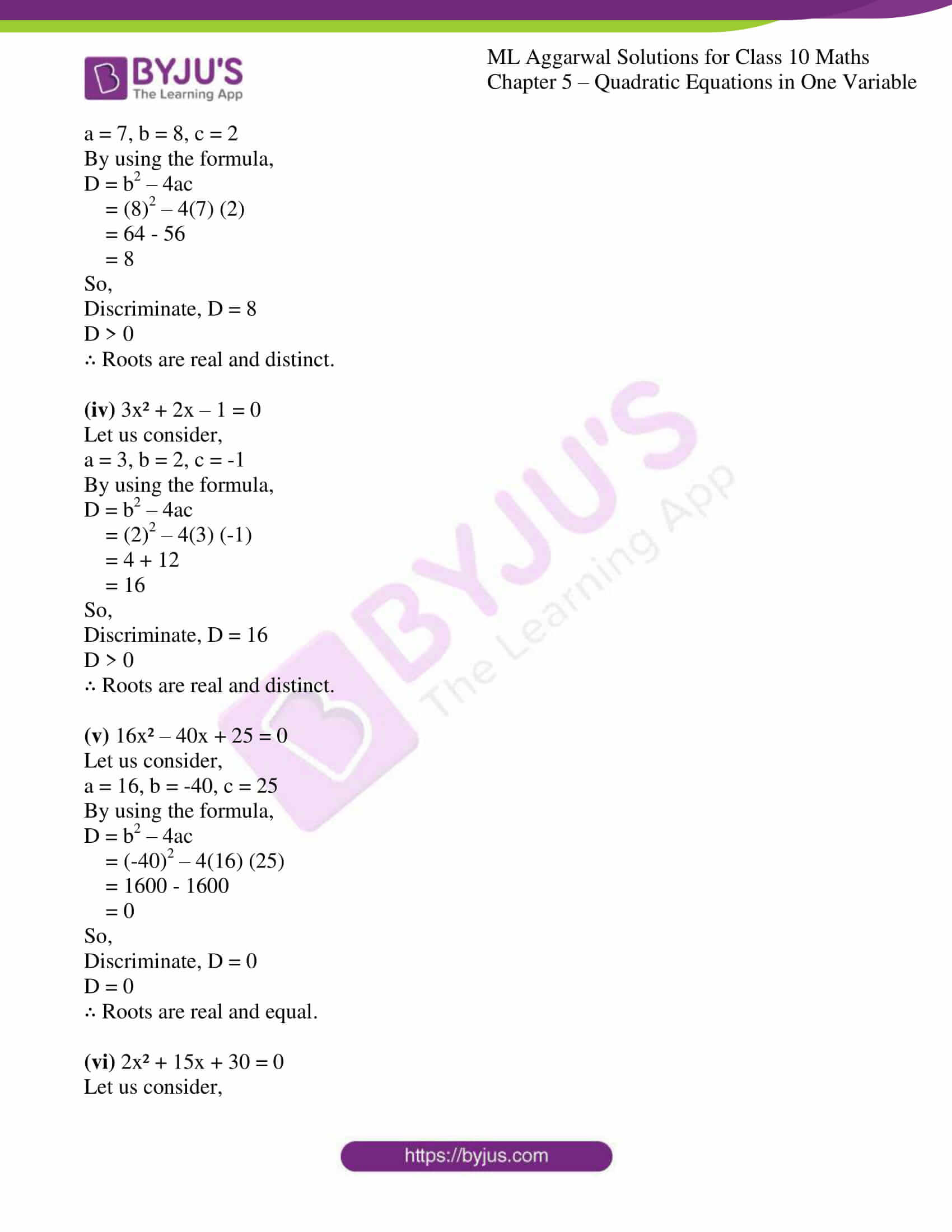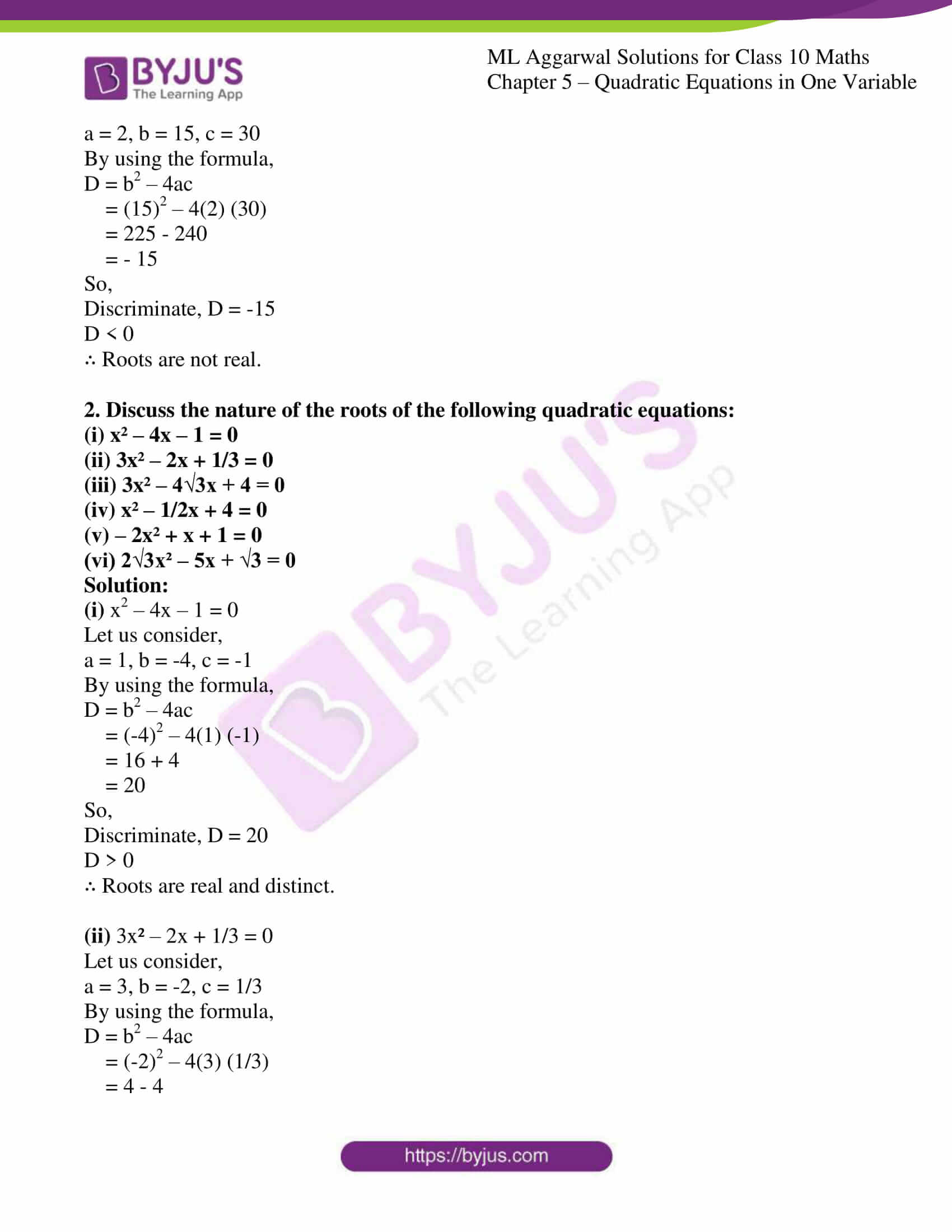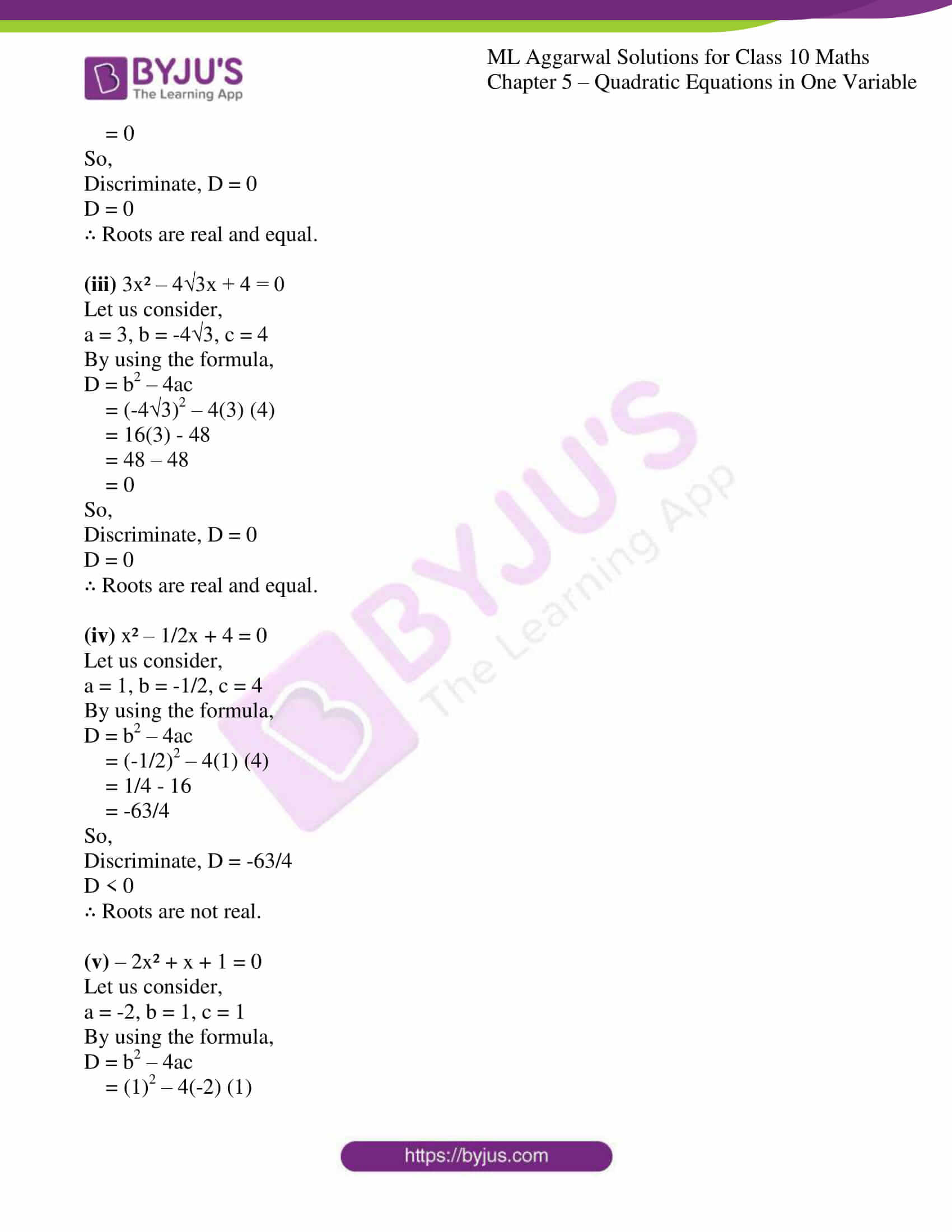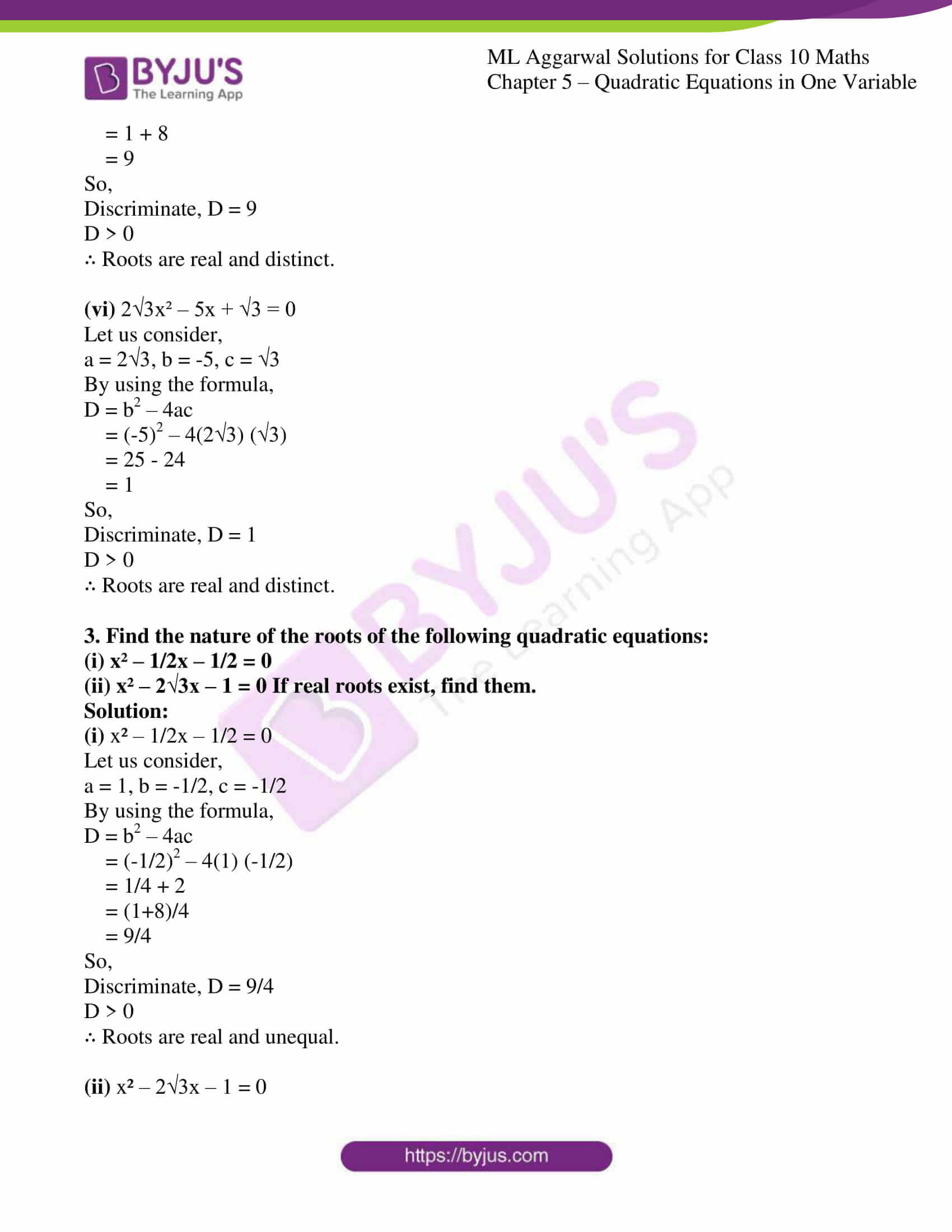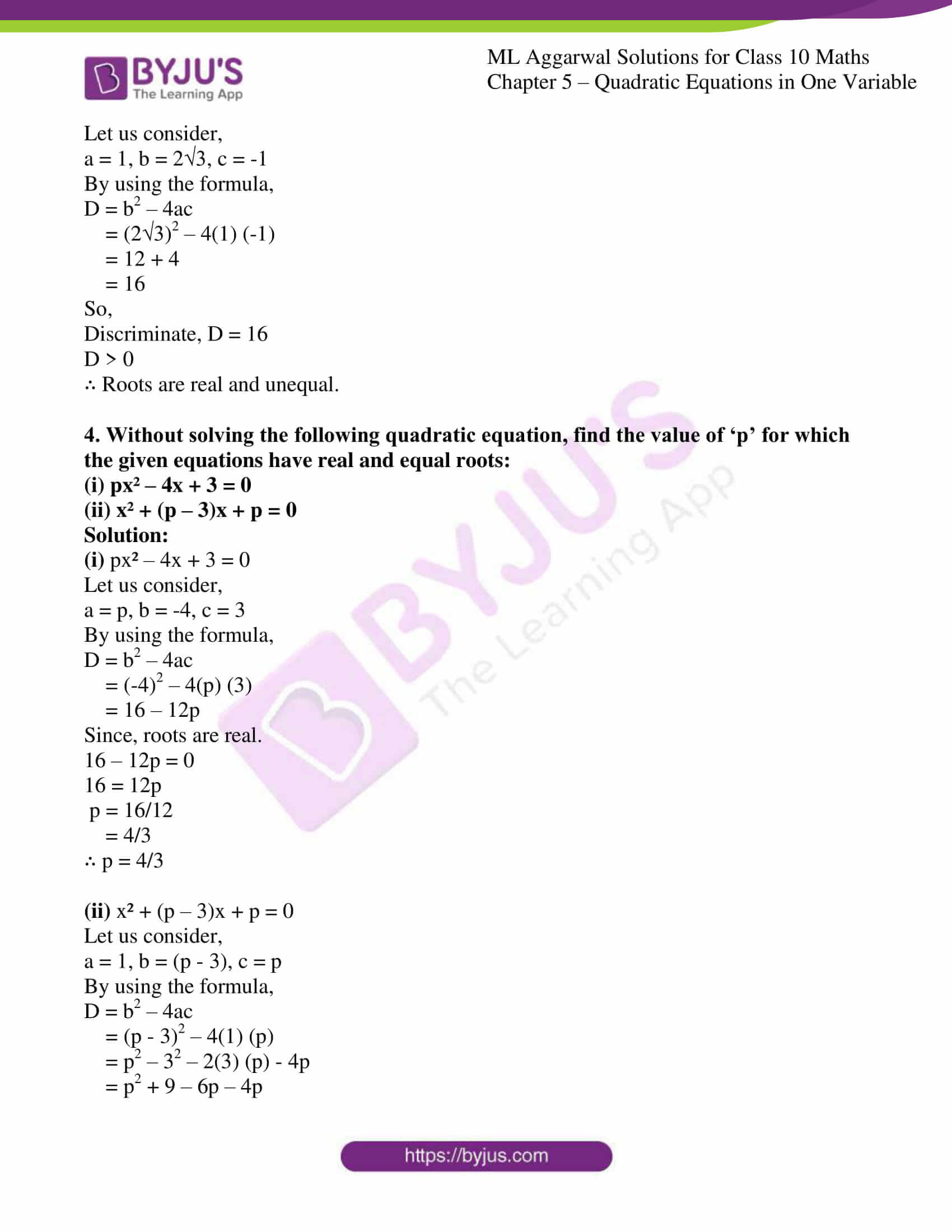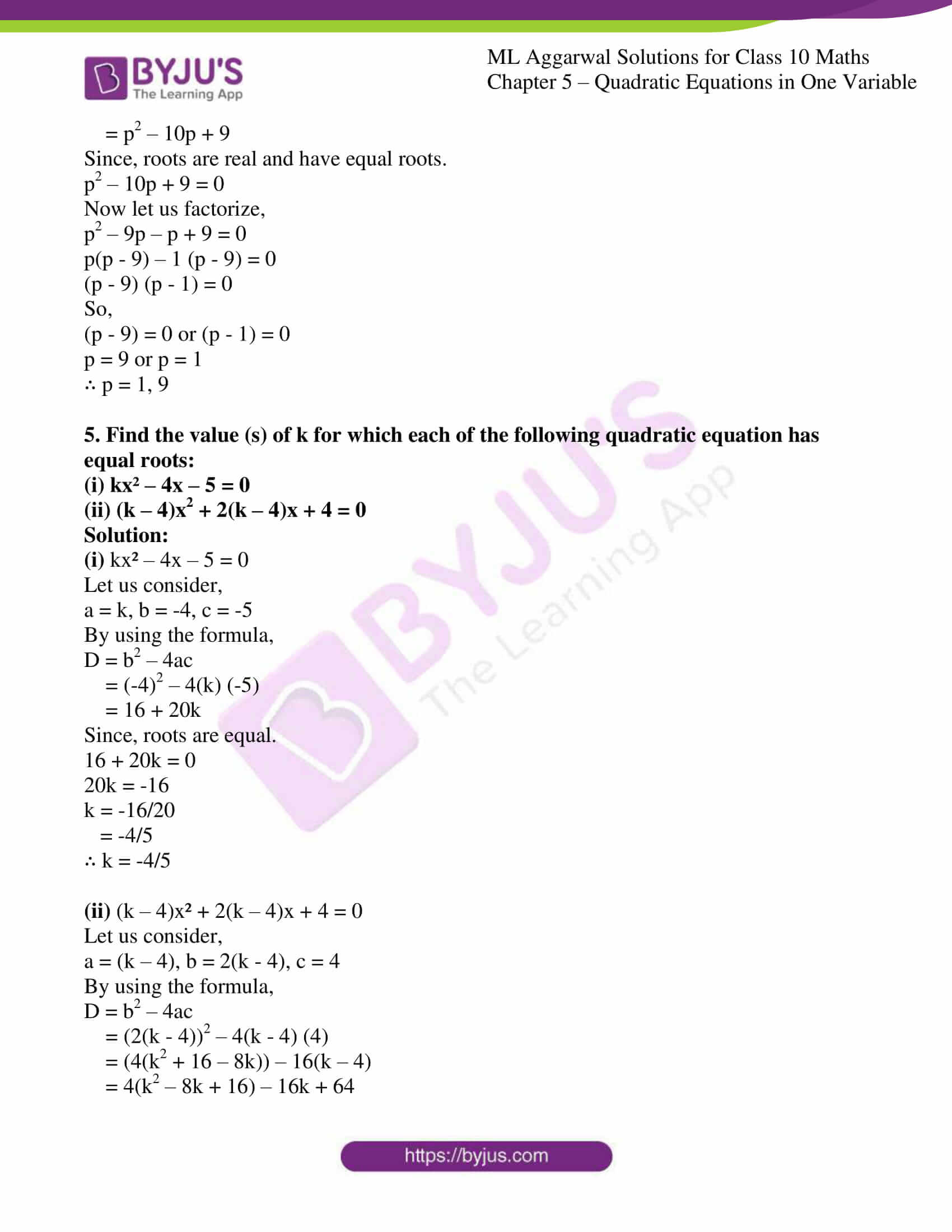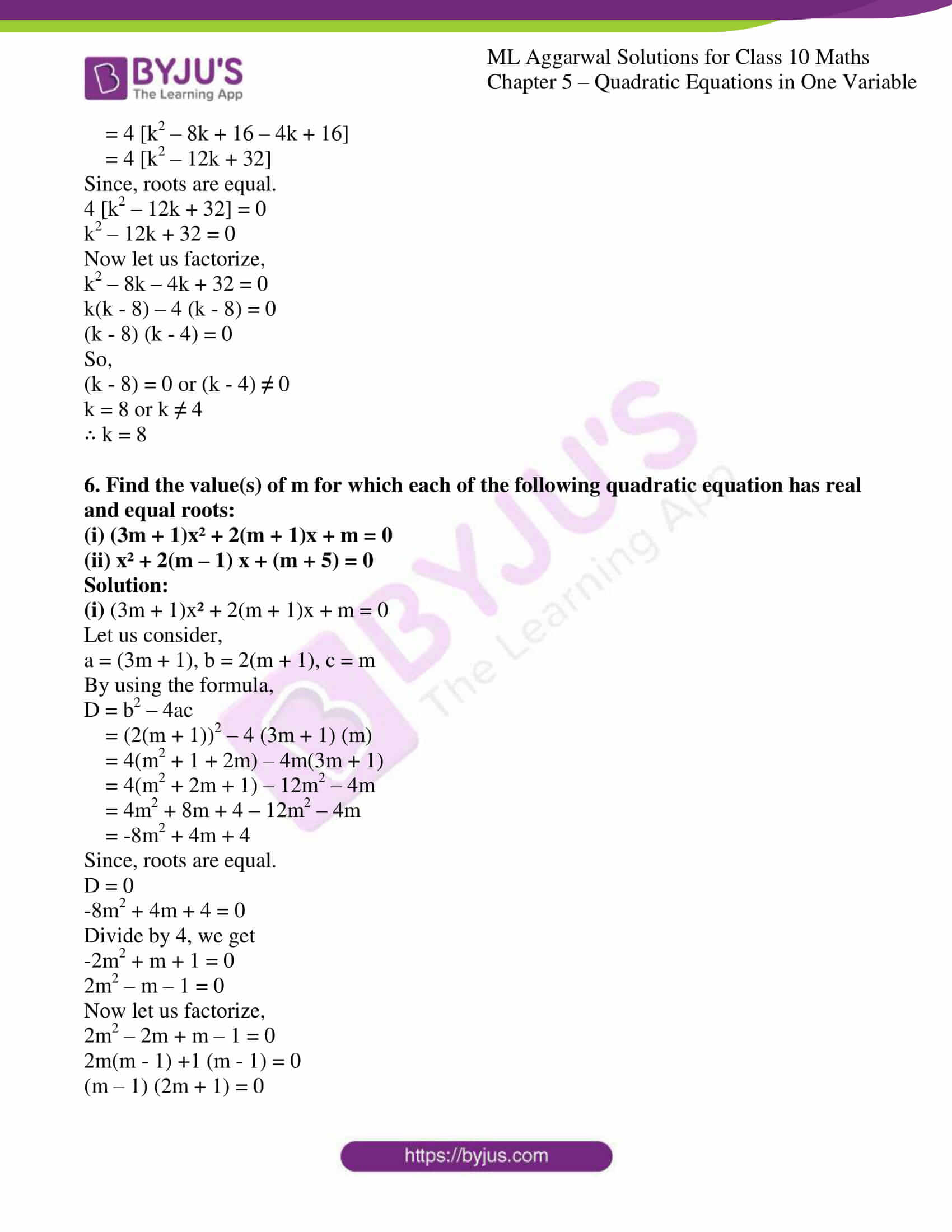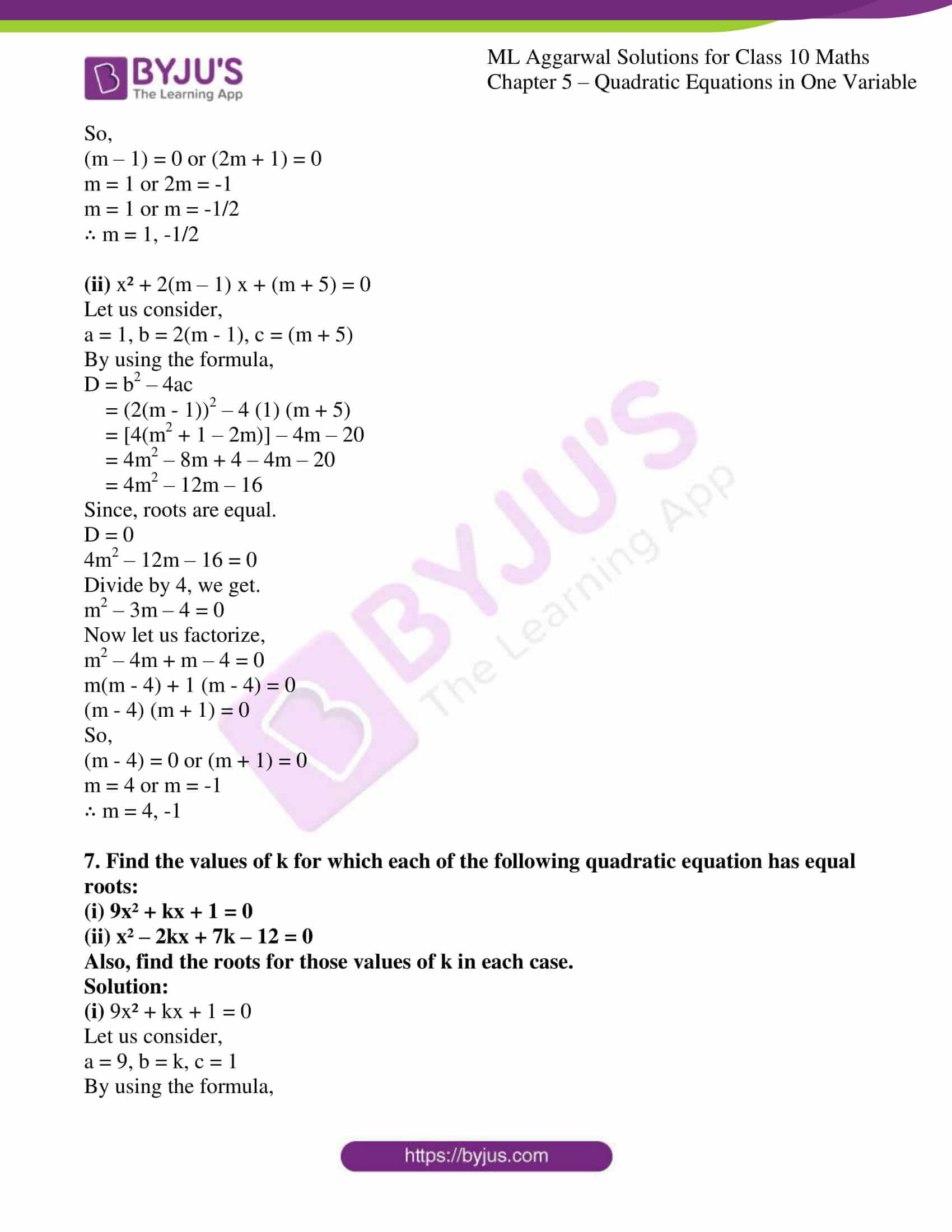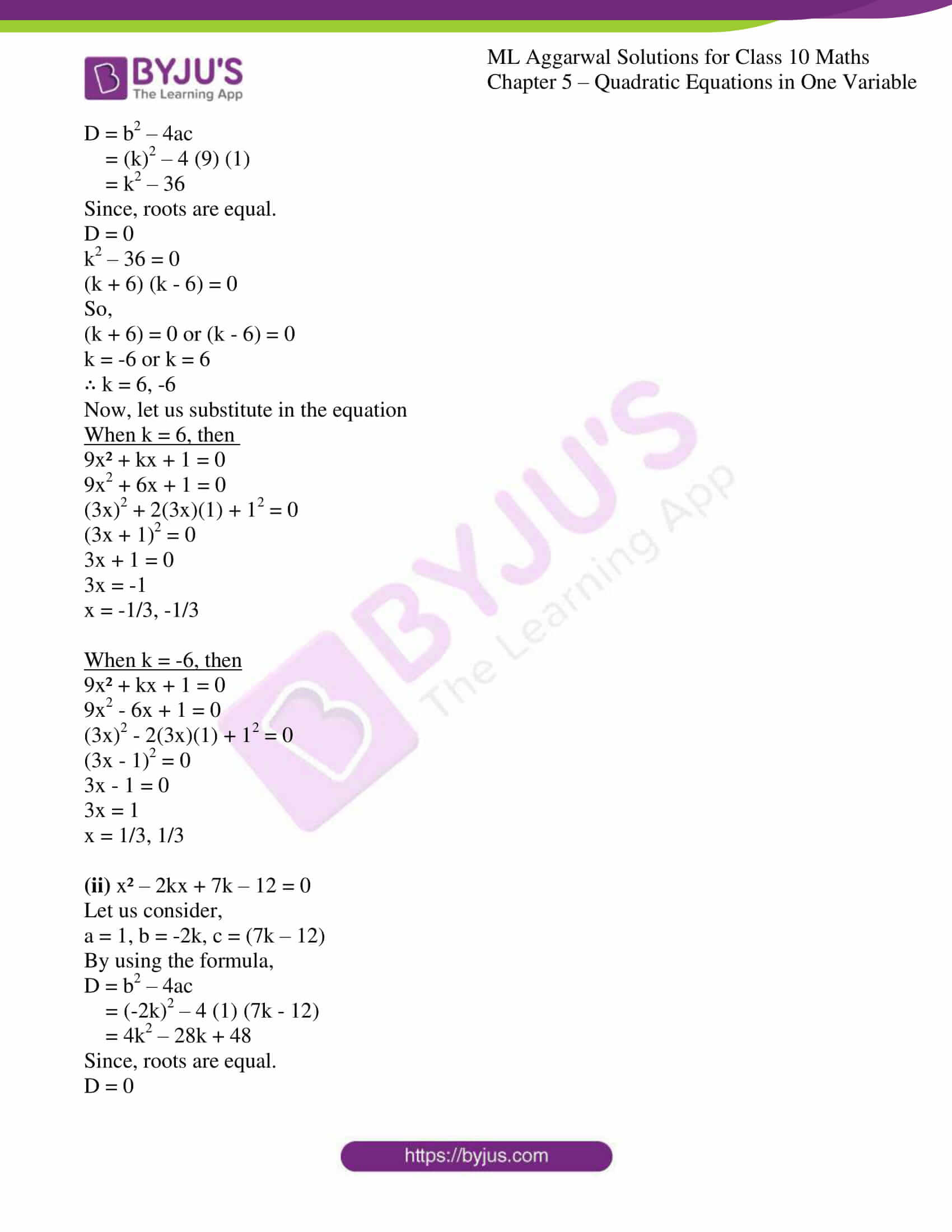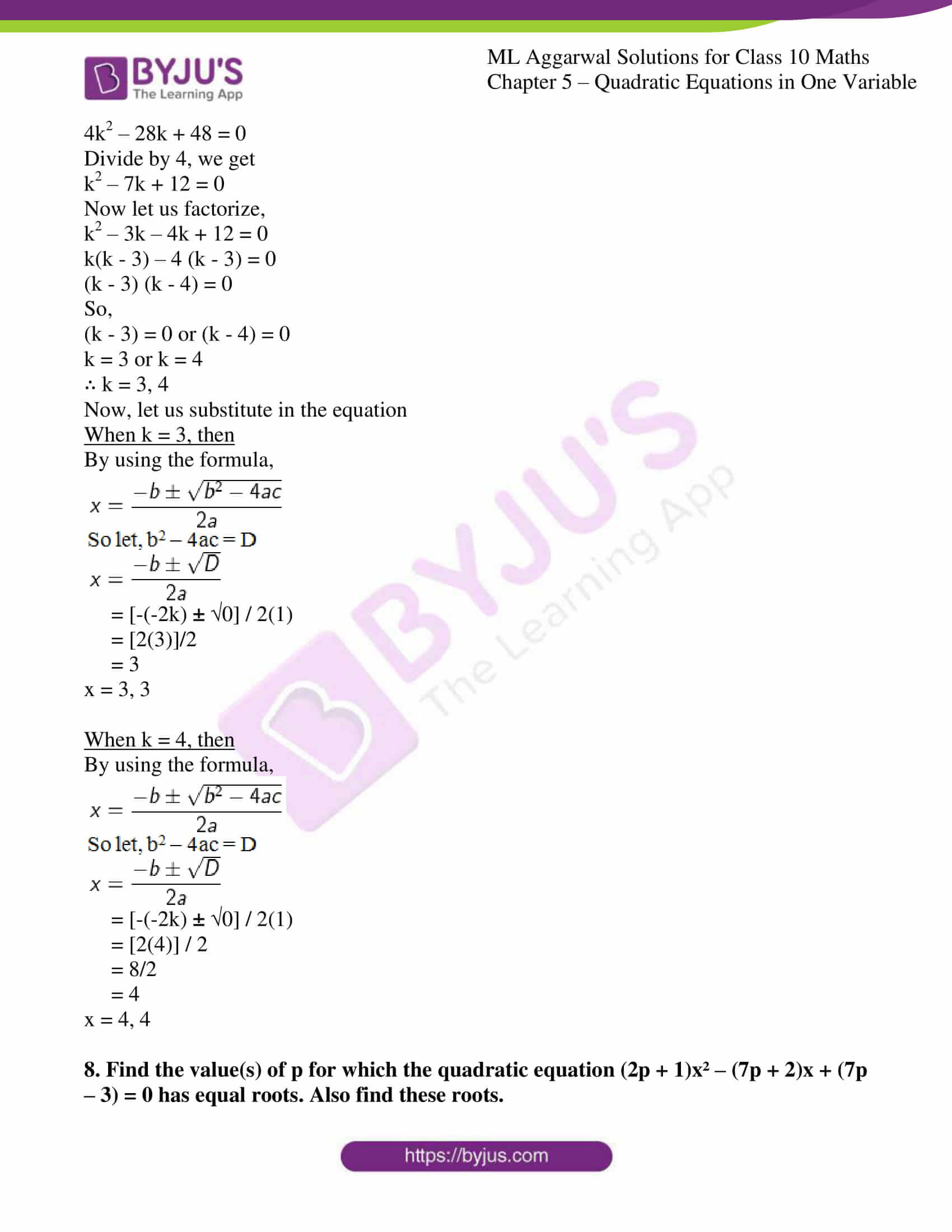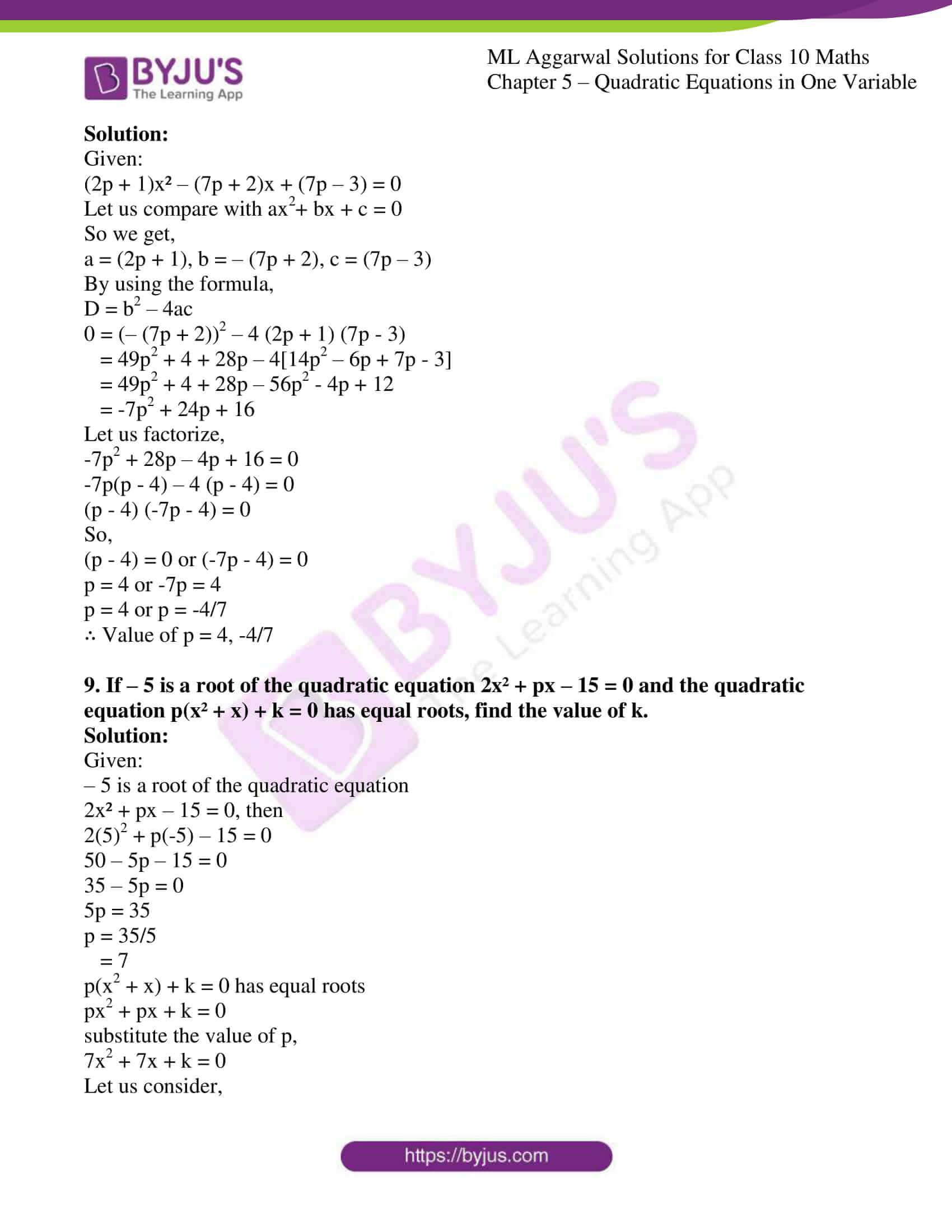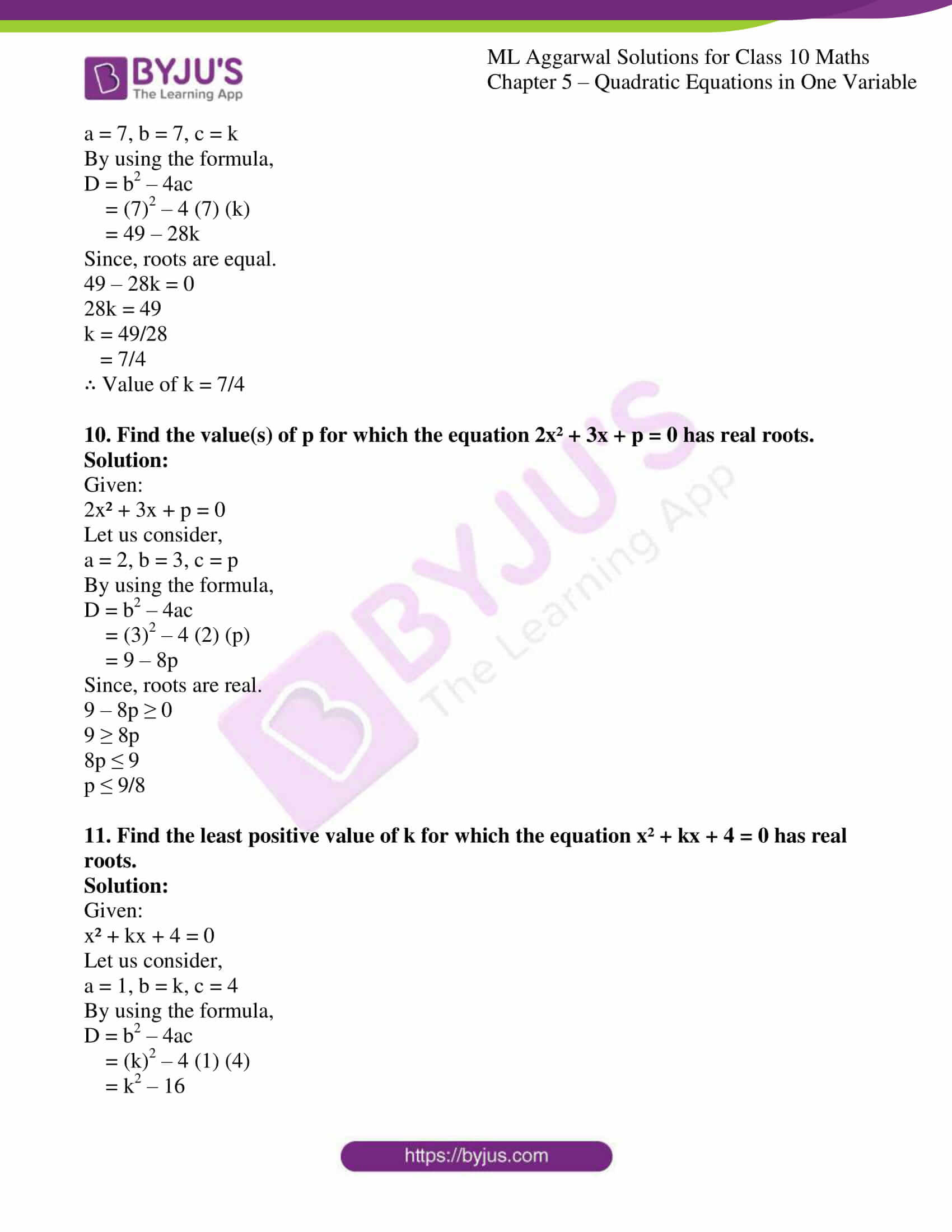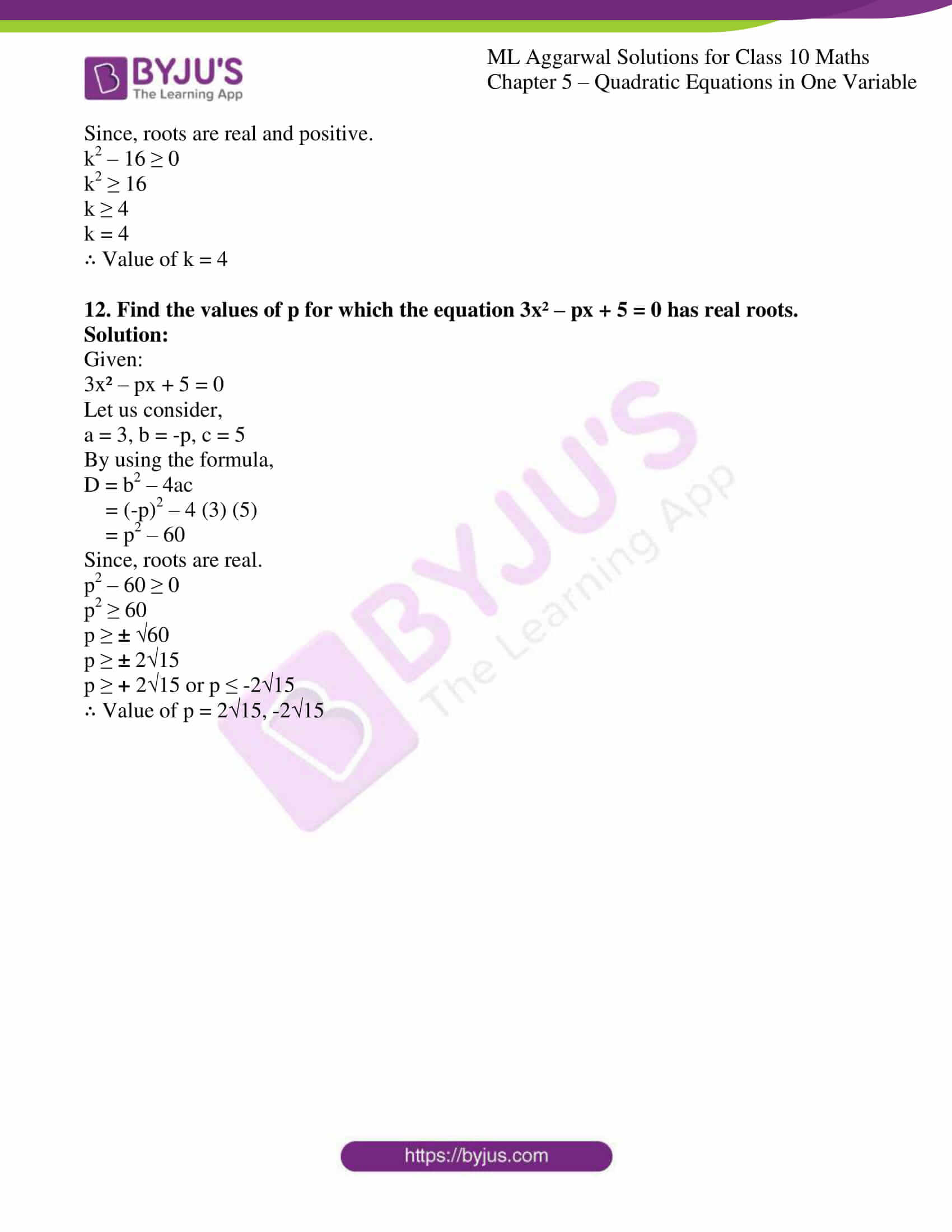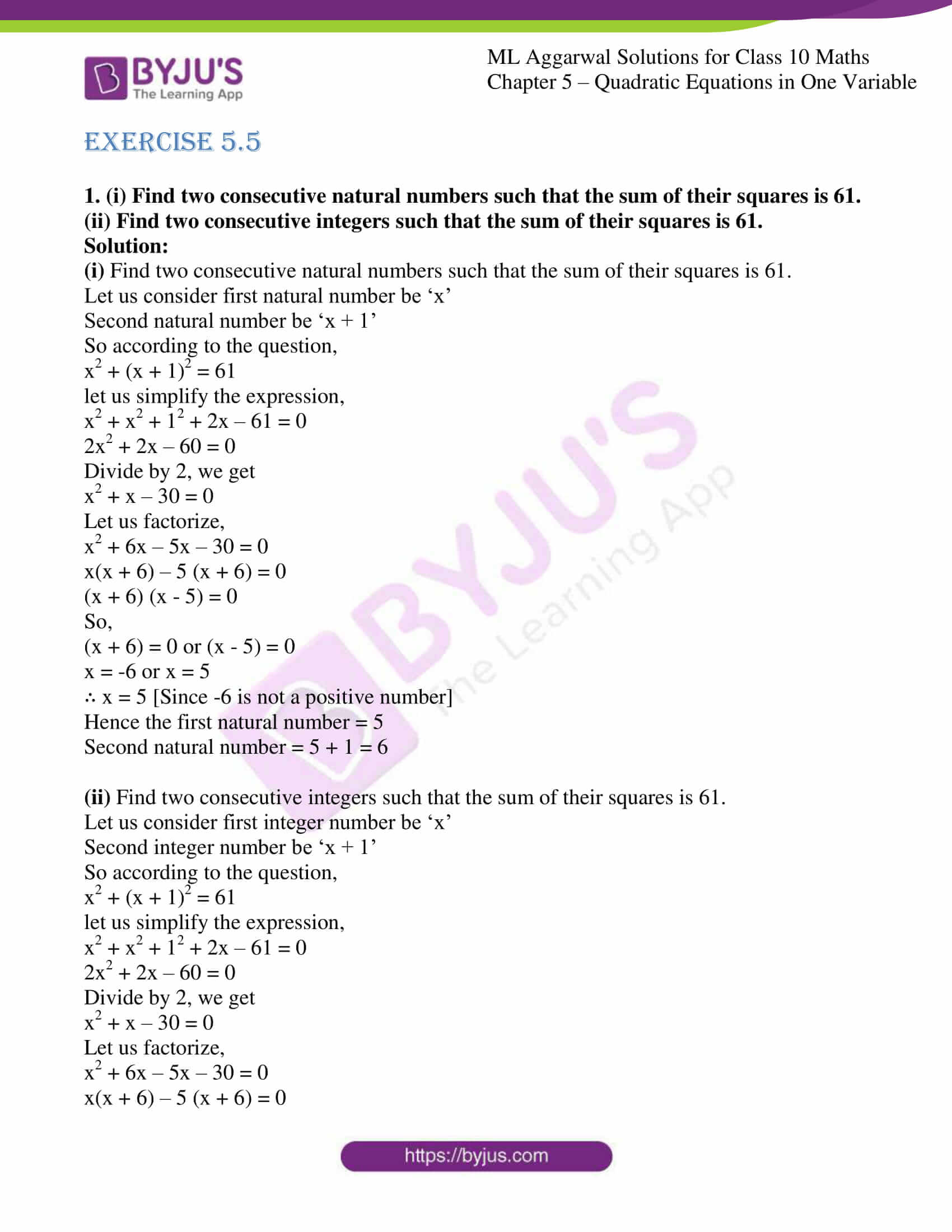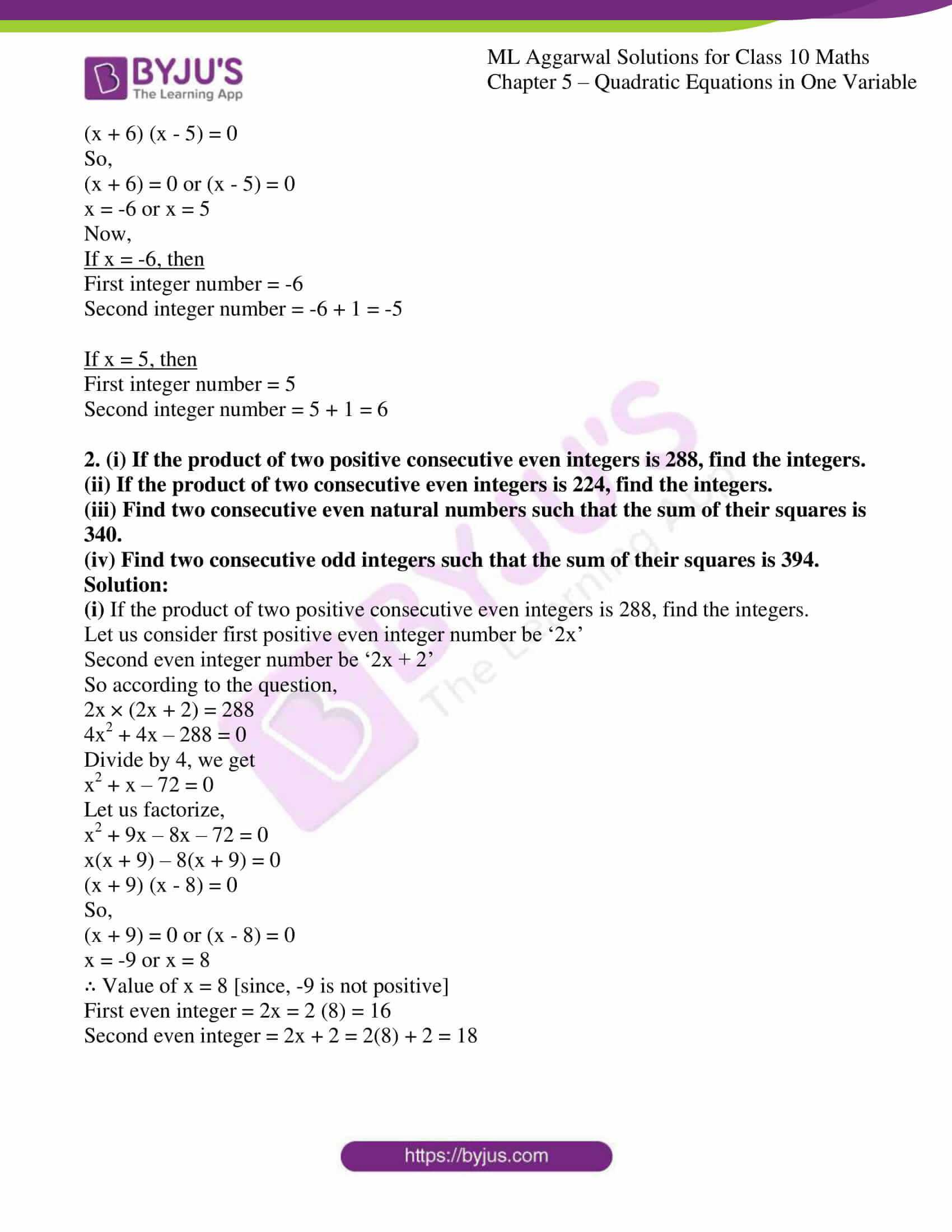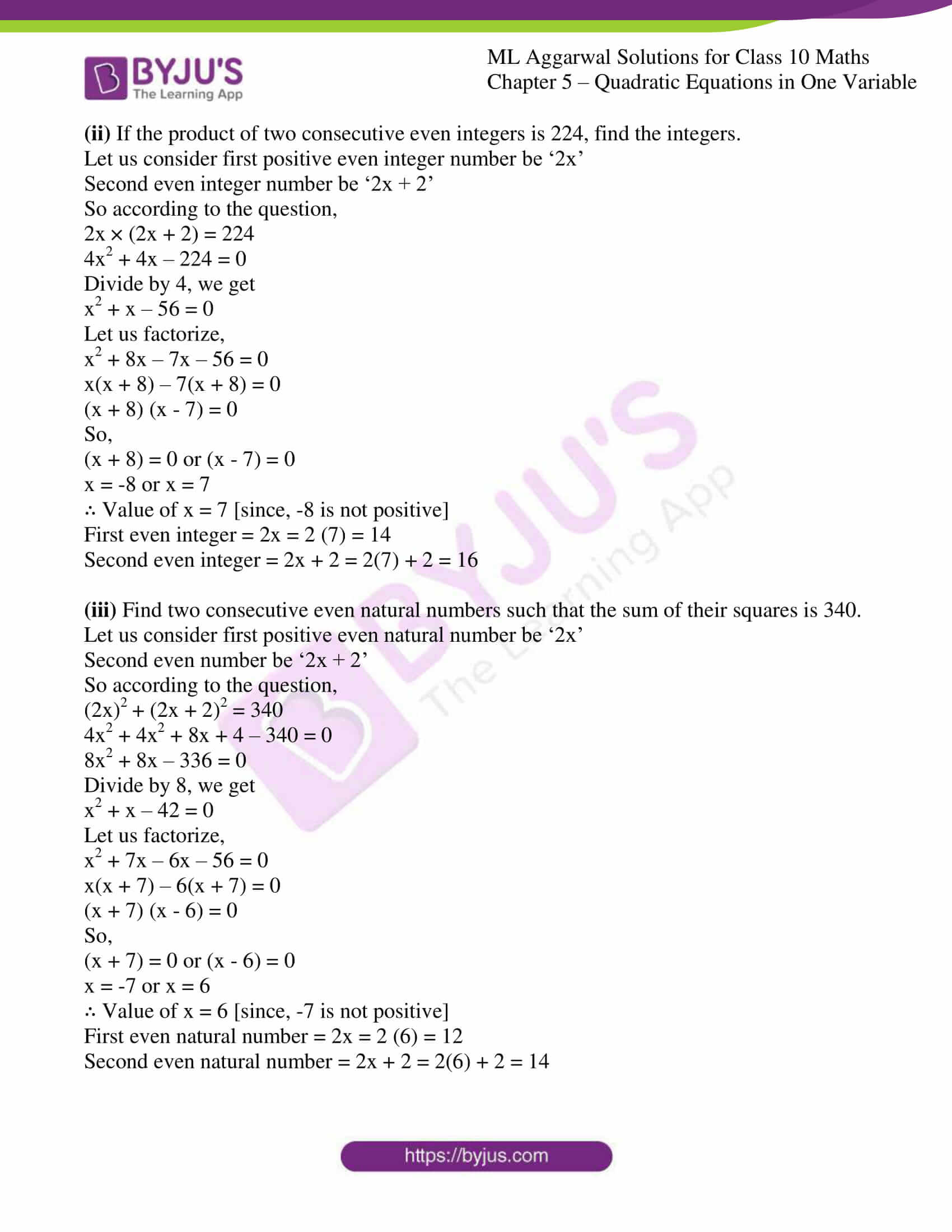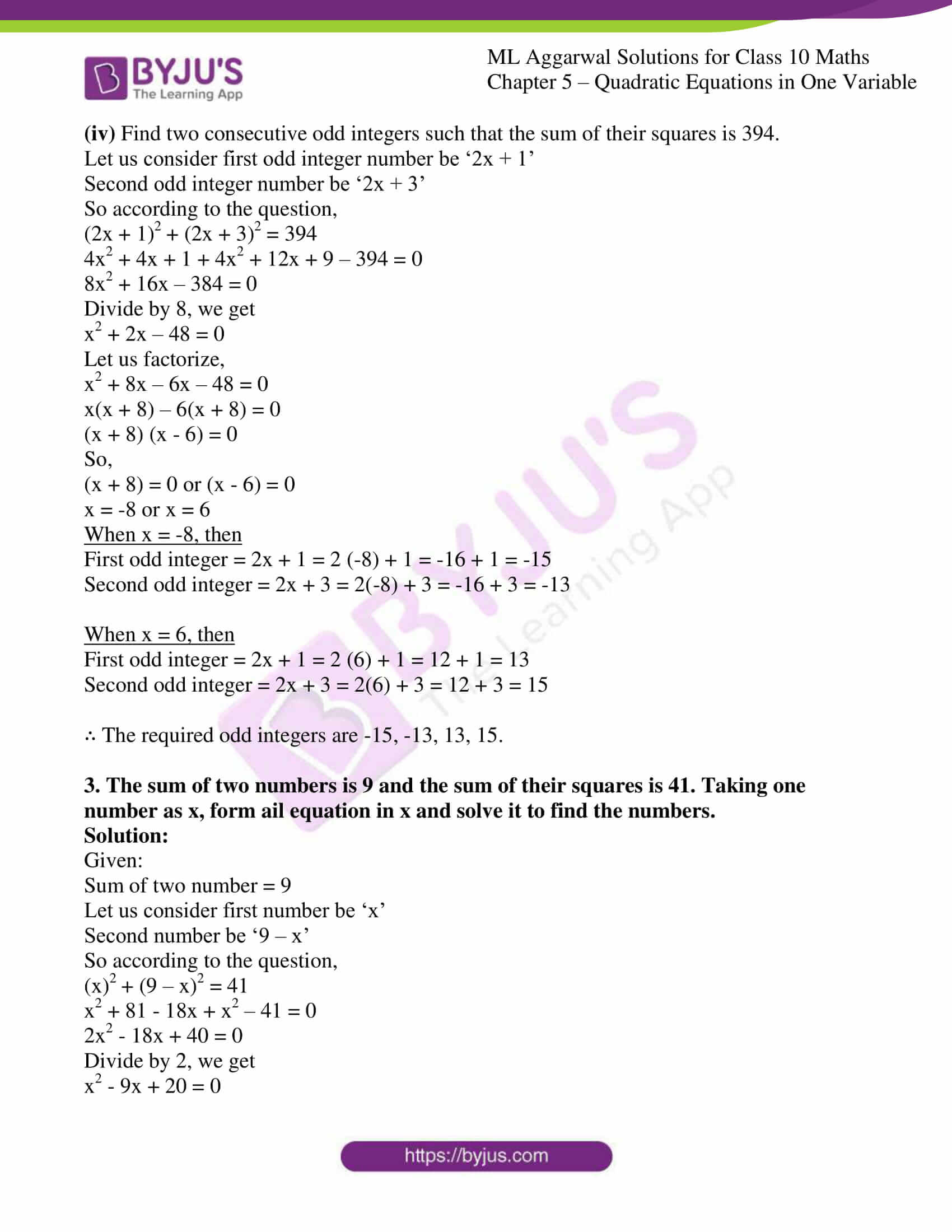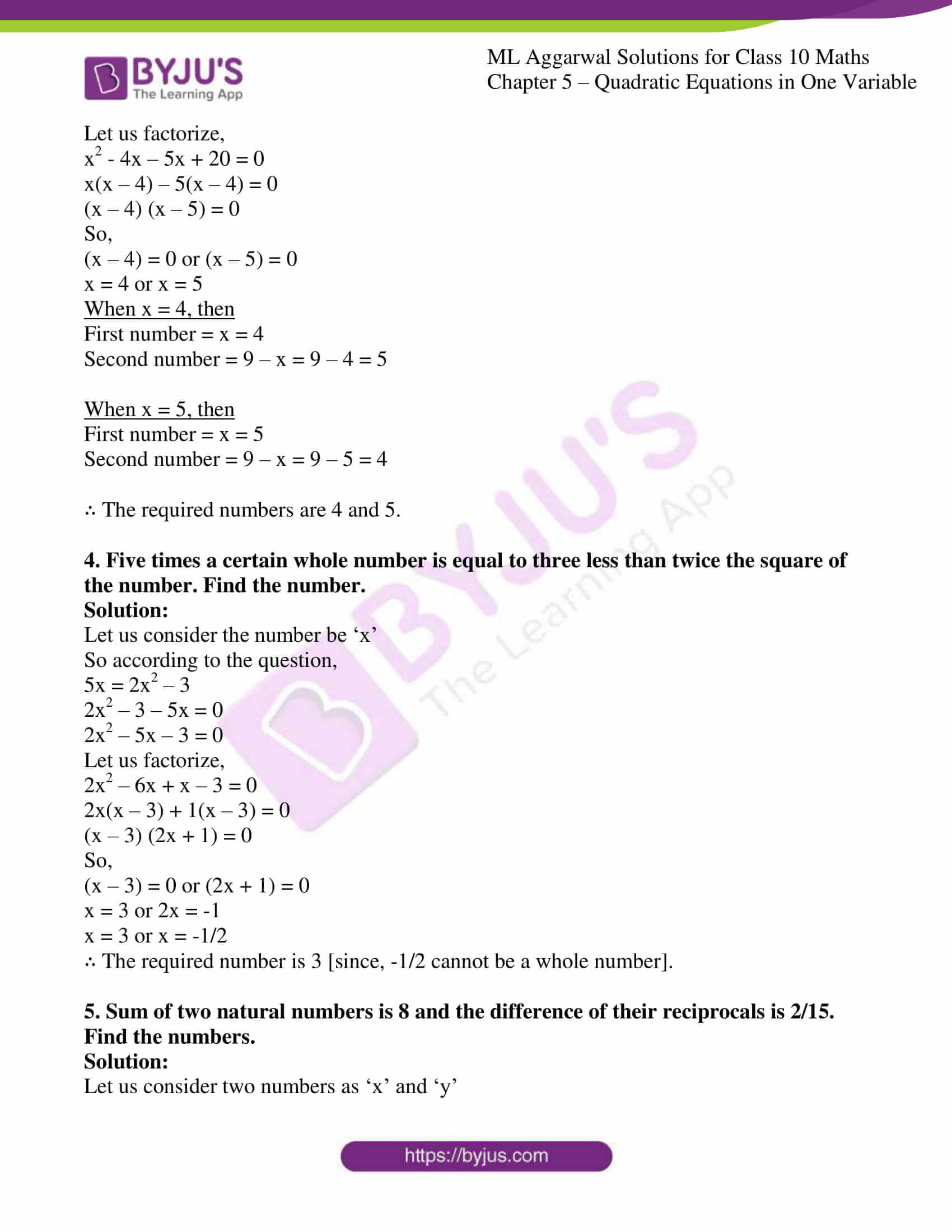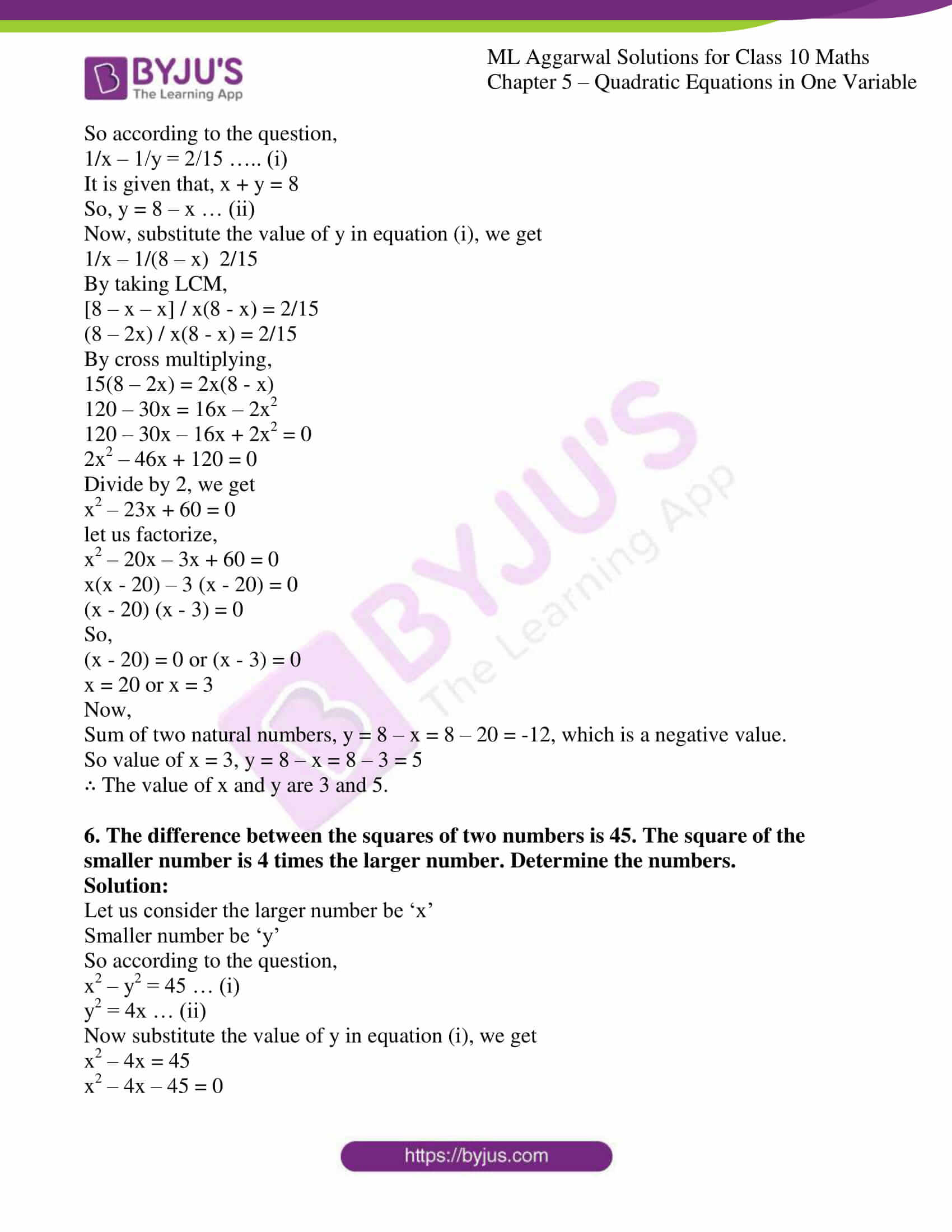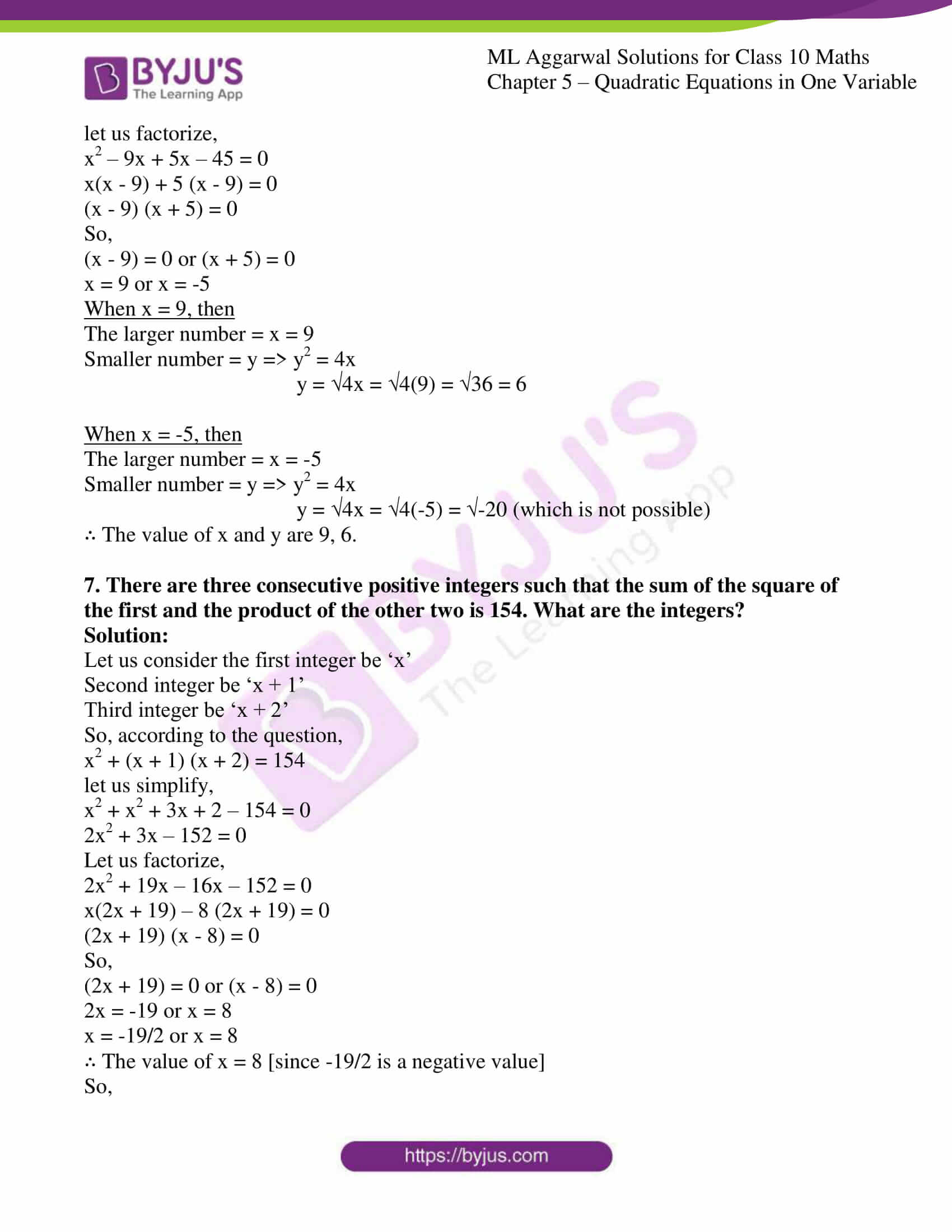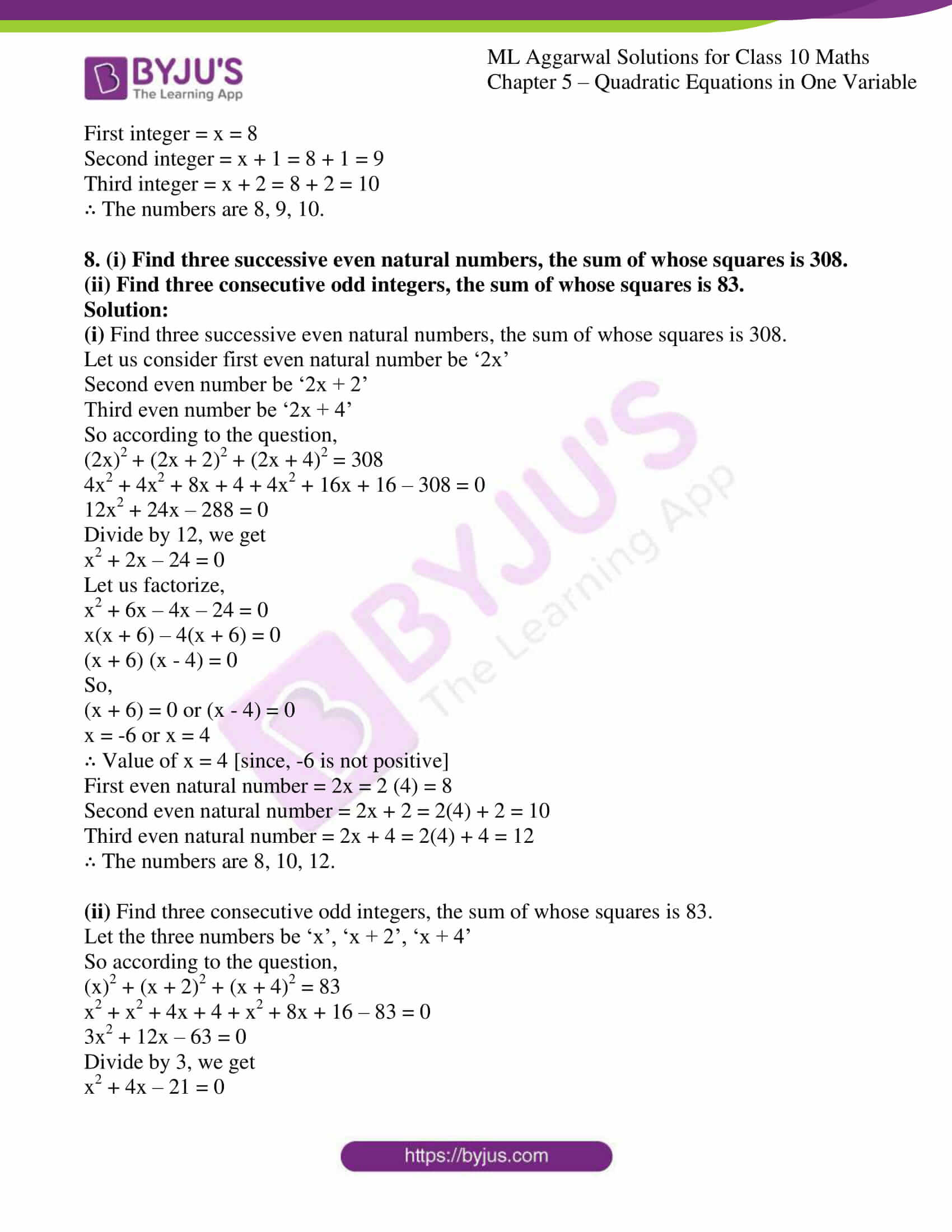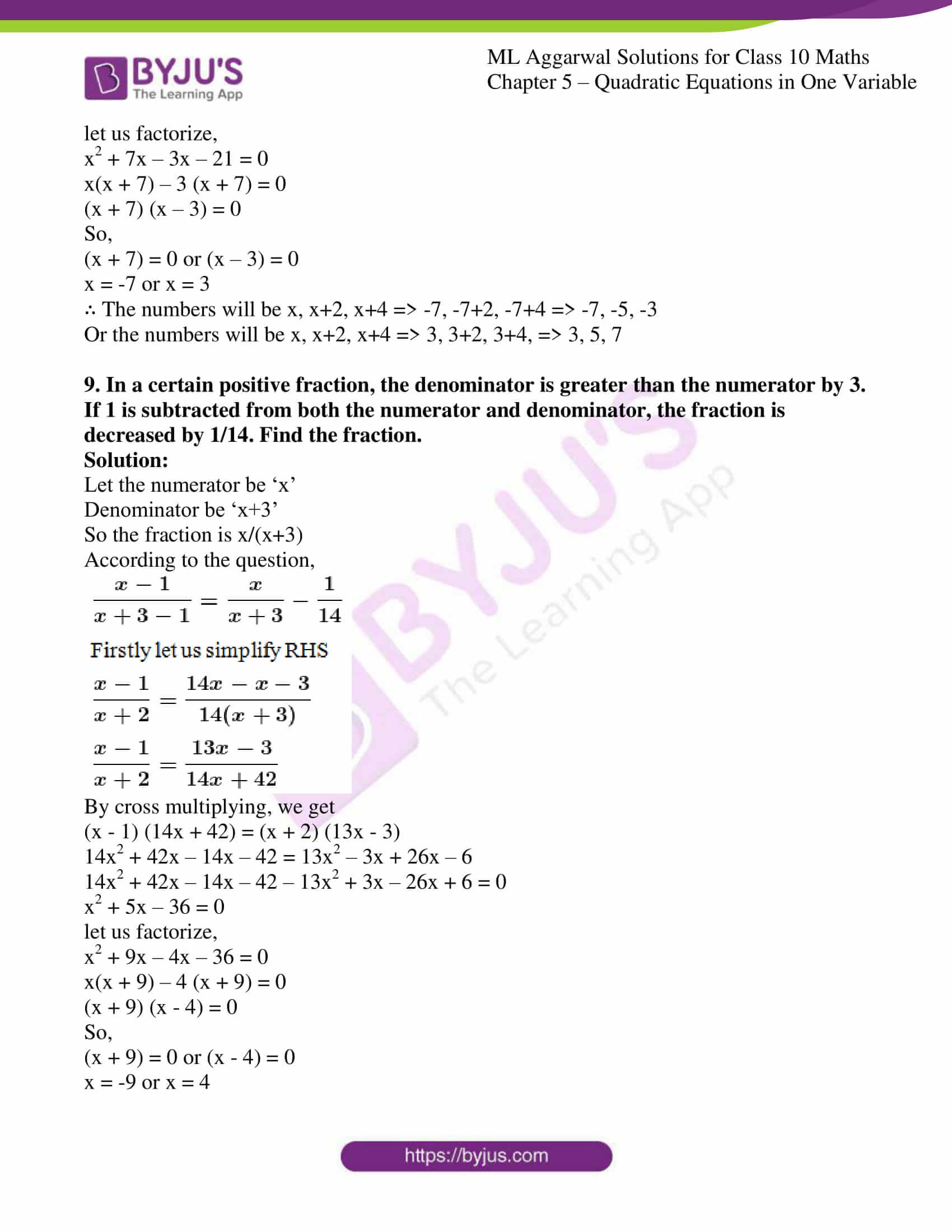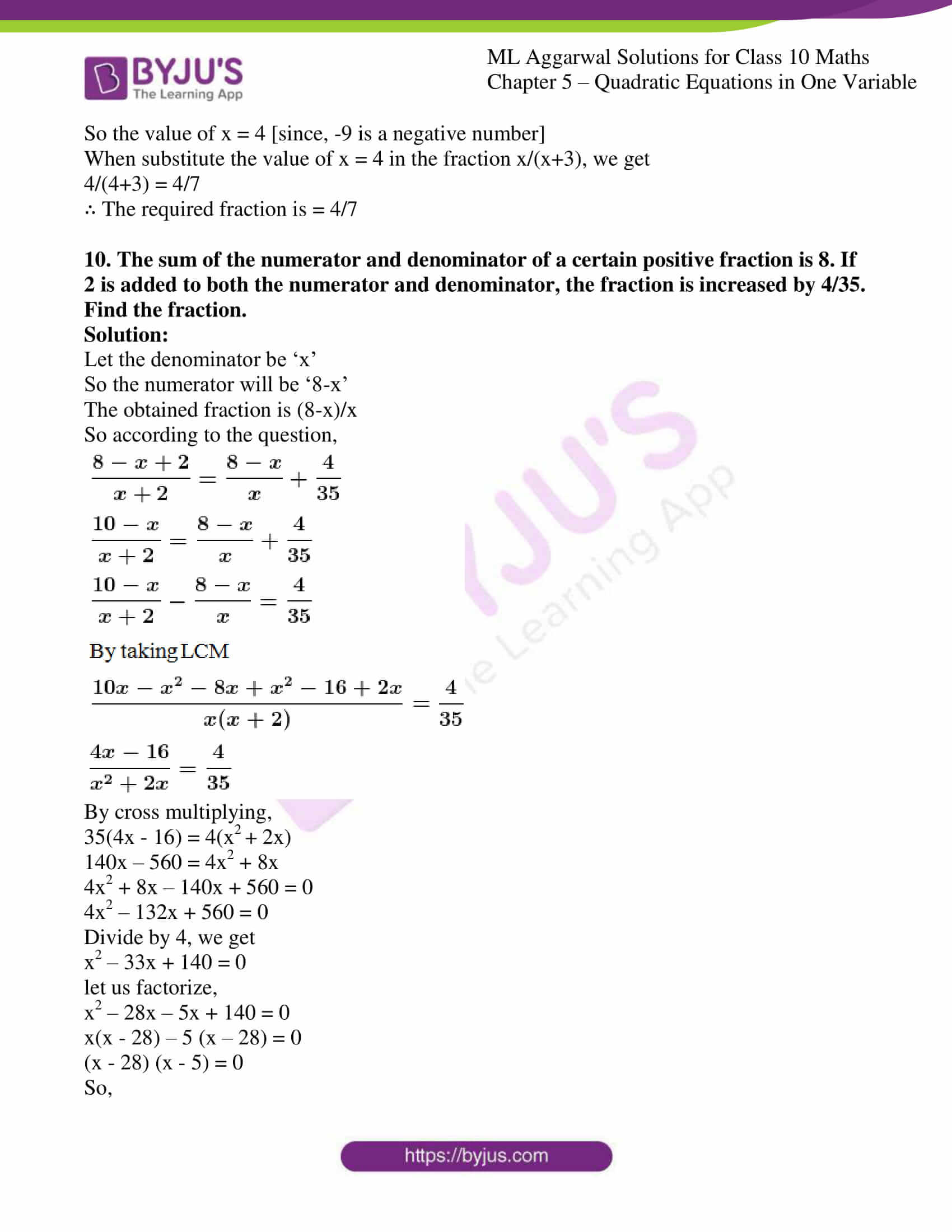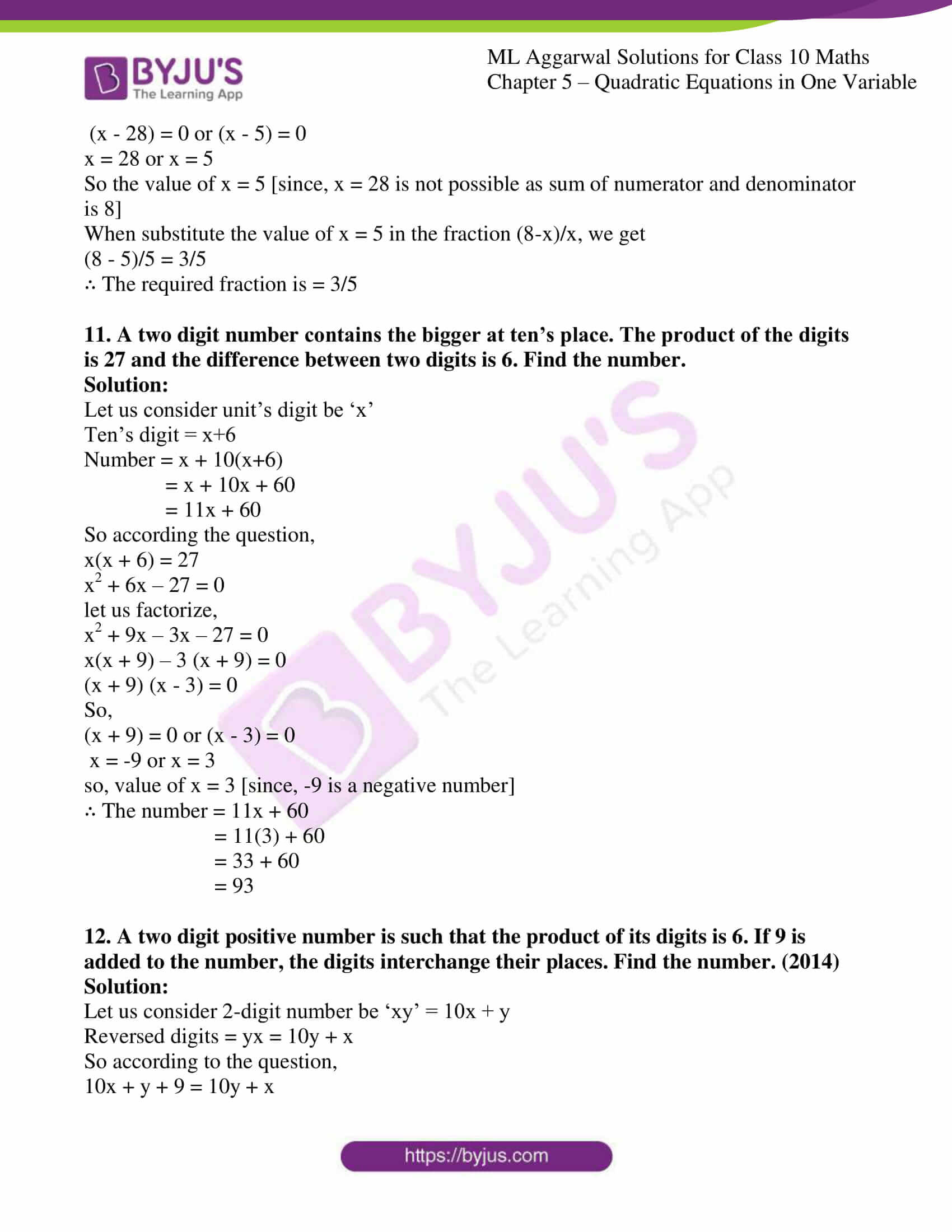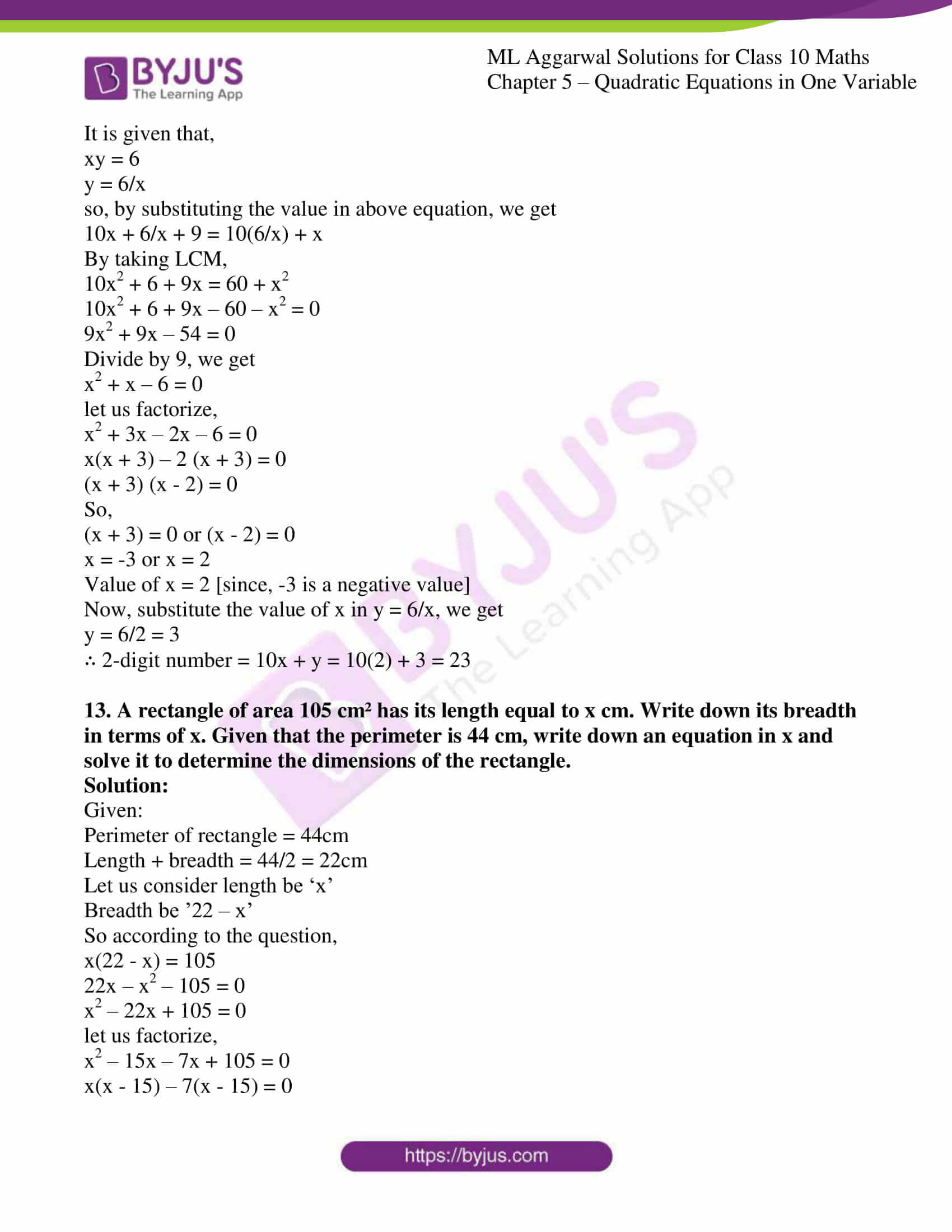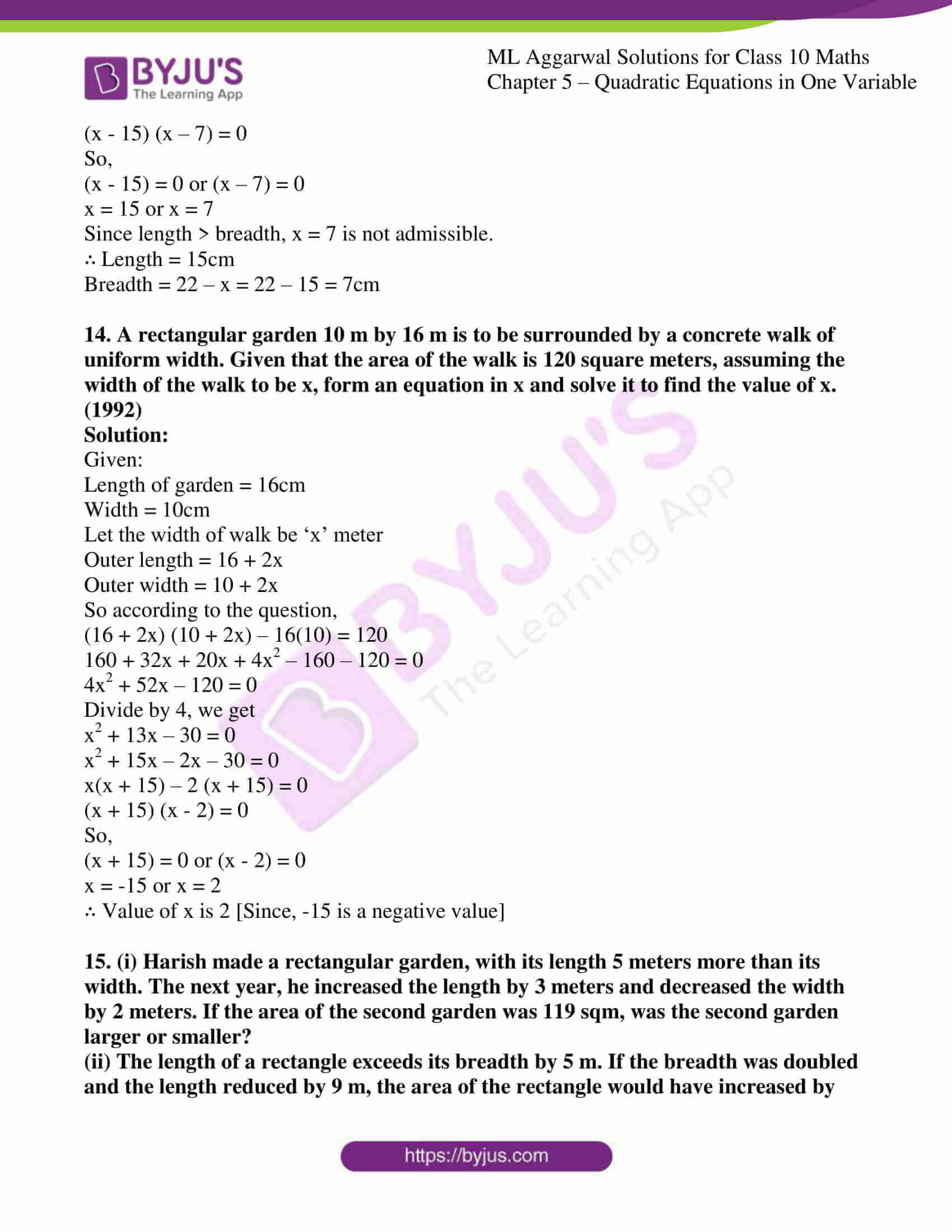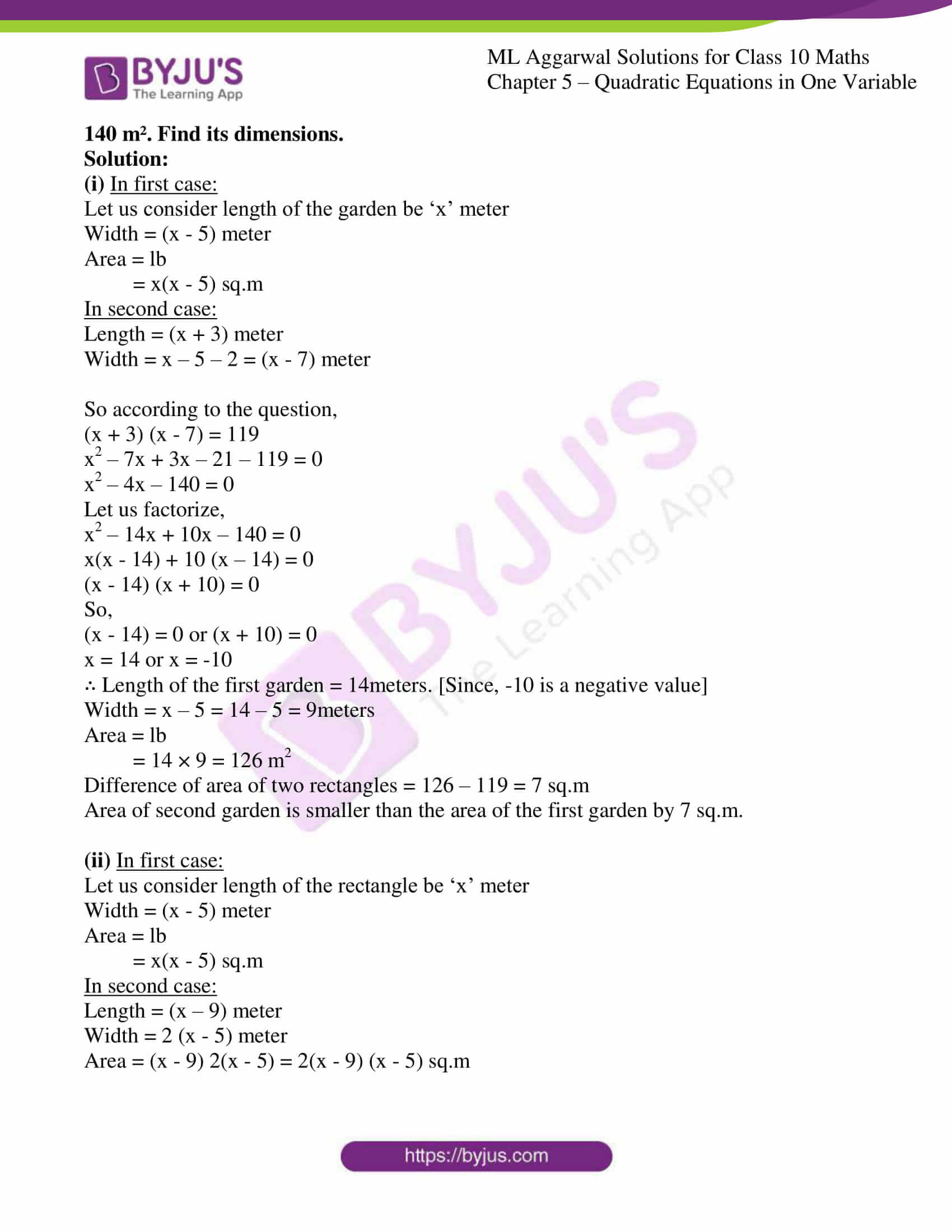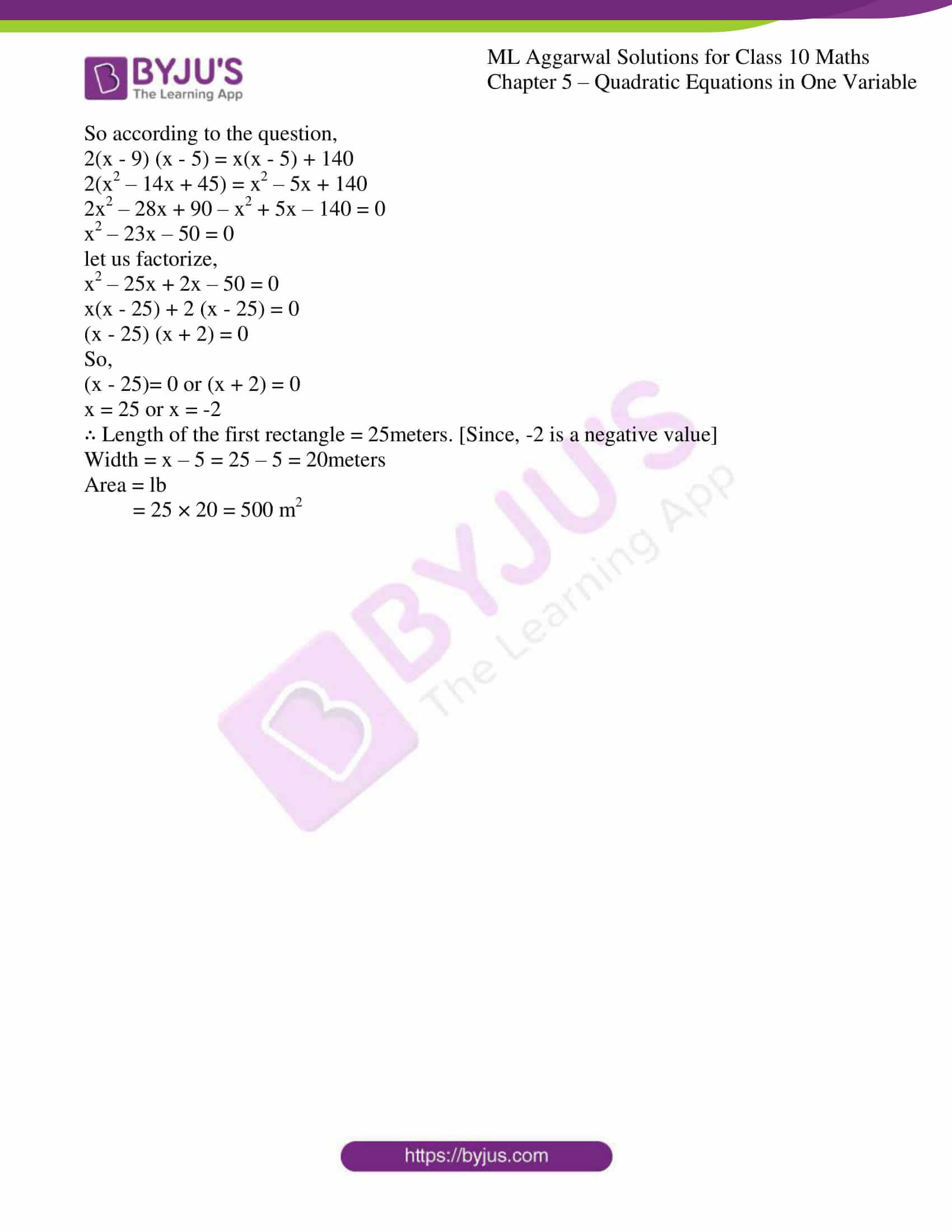### Access answers to ML Aggarwal Solutions for Class 10 Maths Chapter 5 – Quadratic Equations in One Variable

1. Check whether the following are quadratic equations:

(i) √3x2 – 2x + 3/5 = 0

(ii) (2x + 1) (3x – 2) = 6(x + 1) (x – 2)

(iii) (x – 3)3 + 5 = x3 + 7x2 – 1

(iv) x – 3/x = 2, x ≠ 0

(v) x + 2/x = x2, x ≠ 0

(vi) x2 + 1/x2 = 3, x ≠ 0

Solution:

(i) √3x2 – 2x + 3/5 = 0

Yes, the given equation is a quadratic equation since it has power of 2.

(ii) (2x + 1) (3x – 2) = 6(x + 1) (x – 2)

Let us solve the given expression,

6x2 – 4x + 3x – 2 = 6(x2 – 2x + x – 2)

6x2 – x – 2 = 6x2 – 12x + 6x – 12

12x – 6x – x = -12 + 2

5x = -10

∴ The given expression is not a quadratic equation.

(iii) (x – 3)3 + 5 = x3 + 7x2 – 1

Let us solve the given expression,

x3 – 3x2 (3) + 3x (9) – 27 + 5 = x3 + 7x2 – 1

-9x2 + 27x – 22 – 7x2 + 1 = 0

-16x2 + 27x – 21 = 0

16x2 – 27x + 21 = 0

∴ The given expression is a quadratic equation.

(iv) x – 3/x = 2, x ≠ 0

Let us solve the given expression,

x2 – 3 = 2x

x2 – 2x – 3 = 0

∴ The given expression is a quadratic equation.

(v) x + 2/x = x2, x ≠ 0

Let us solve the given expression,

By taking LCM we,

x2 + 2 = x3

x3 – x2 – 2 = 0

∴ The given expression is a quadratic equation.

(vi) x2 + 1/x2 = 3, x ≠ 0

Let us solve the given expression,

By taking LCM we,

x4 + 1 = 3x2

x4 – 3x2 + 1 = 0

∴ The given expression is not a quadratic equation.

2. In each of the following, determine whether the given numbers are roots of the given equations or not;
(i) x2 – x + 1 = 0; 1, -1
(ii) x2 – 5x + 6 = 0; 2, -3

(iii) 3x2 – 13x – 10 = 0; 5, -2/3

(iv) 6x2 – x – 2 = 0; -1/2, 2/3

Solution:

(i) x2 – x + 1 = 0; 1, -1

Let us substitute the given values in the expression and check,

When, x = 1

x2 – x + 1 = 0

(1)2 – 1 + 1 = 0

1 – 1 + 1 = 0

1 = 0

∴ x ≠ 0

When, x = -1

x2 – x + 1 = 0

(-1)2 – 1 + 1 = 0

1 – 1 + 1 = 0

1 = 0

∴ x ≠ 0

Hence, the given values x = 1, -1 are not roots of the equation.

(ii) x2 – 5x + 6 = 0; 2, -3

Let us substitute the given values in the expression and check,

When, x = 2

x2 – 5x + 6 = 0

(2)2 – 5(2) + 6 = 0

4 – 10 + 6 = 0

0 = 0

∴ x = 0

When, x = -3

x2 – 5x + 6 = 0

(-3)2 – 5(-3) + 6 = 0

9 + 15 + 6 = 0

30 = 0

∴ x ≠ 0

Hence, the value x = 2 is the root of the equation.

And value x = -3 is not a root of the equation.

(iii) 3x2 – 13x – 10 = 0; 5, -2/3

Let us substitute the given values in the expression and check,

When, x = 5

3x2 – 13x – 10 = 0

3(5)2 – 13(5) – 10 = 0

3(25) – 65 – 10 = 0

75 – 75 = 0

0 = 0

∴ x = 0

When, x = -2/3

3x2 – 13x – 10 = 0

3(-2/3)2 – 13(-2/3) – 10 = 0

4/9 + 26/3 – 10 = 0

4/3 + 26/3 – 10 = 0

30/3 – 10 = 0

10 – 10 = 0

∴ x = 0

Hence, the value x = 5, -2/3 are the roots of the equation.

(iv) 6x2 – x – 2 = 0; -1/2, 2/3

Let us substitute the given values in the expression and check,

When, x = -1/2

6x2 – x – 2 = 0

6(-1/2)2 – (-1/2) – 2 = 0

6/4 + ½ – 2 = 0

3/2 + ½ – 2 = 0

4/2 – 2 = 0

2 – 2 = 0

0 = 0

∴ x = 0

When, x = 2/3

6x2 – x – 2 = 0

6(2/3)2 – (2/3) – 2 = 0

6(4/9) – 2/3 – 2 = 0

8/3 – 2/3 – 2 = 0

6/3 – 2 = 0

2 – 2 = 0

0 = 0

∴ x = 0

Hence, the value x = -1/2, 2/3 are the roots of the equation.

3. In each of the following, determine whether the given numbers are solutions of the given equation or not:

(i) x2 – 3√3x + 6 = 0; x = √3, -2√3

(ii) x2 – √2x – 4 = 0; x = -√2, 2√2

Solution:

(i) x2 – 3√3x + 6 = 0; x = √3, -2√3

Let us substitute the given values in the expression and check,

When, x = √3

x2 – 3√3x + 6 = 0

(√3)2 – 3√3(√3) + 6 = 0

3 – 9 + 6 = 0

-9 + 9 =0

0 = 0

∴ √3 is the solution of the equation.

When, x = -2√3

x2 – 3√3x + 6 = 0

(-2√3)2 – 3√3(-2√3) + 6 = 0

4(3) +18 + 6 = 0

12 + 18 + 6 = 0

36 =0

∴ -2√3 is not the solution of the equation.

(ii) x2 – √2x – 4 = 0; x = -√2, 2√2

Let us substitute the given values in the expression and check,

When, x = -√2

x2 – √2x – 4 = 0

(-√2)2 – √2(-√2) – 4 = 0

2 + 2 – 4 = 0

4 – 4 = 0

0 = 0

∴ -√2 is the solution of the equation.

When, x = 2√2

x2 – √2x – 4 = 0

(2√2)2 – √2(2√2) – 4 = 0

4(2) – 4 – 4 = 0

4 – 4 = 0

0 = 0

∴ 2√2 is the solution of the equation.

4. (i) If –1/2 is a solution of the equation 3x² + 2kx – 3 = 0, find the value of k.
(ii) If 2/3 is a solution of the equation 7x² + kx – 3 = 0, find the value of k.
Solution:

(i) If –1/2 is a solution of the equation 3x² + 2kx – 3 = 0, find the value of k.

Let us substitute the given value x = -1/2 in the expression, we get

3x² + 2kx – 3 = 0

3(-1/2)2 + 2k(-1/2) – 3 = 0

3/4 – k – 3 = 0

¾ – 3 = k

By taking LCM

k = (3-12)/4

= -9/4

∴ Value of k = -9/4.

(ii) If 2/3 is a solution of the equation 7x² + kx – 3 = 0, find the value of k.

Let us substitute the given value x = 2/3 in the expression, we get

7x² + kx – 3 = 0

7(2/3)2 + k(2/3) – 3 = 0

7(4/9) + 2k/3 – 3 = 0

28/9 – 3 + 2k/3 = 0

2k/3 = 3 – 28/9

By taking LCM on the RHS

2k/3 = (27 – 28)/9

= -1/9

k = -1/9 × (3/2)

= -1/6

∴ Value of k = -1/6.

5. (i) If √2 is a root of the equation kx² + √2x – 4 = 0, find the value of k.
(ii) If a is a root of the equation x² – (a + b)x + k = 0, find the value of k.
Solution:

(i) If √2 is a root of the equation kx² + √2x – 4 = 0, find the value of k.

Let us substitute the given value x = √2 in the expression, we get

kx² + √2x – 4 = 0

k(√2)2 + √2(√2) – 4 = 0

2k + 2 – 4 = 0

2k – 2 = 0

k = 2/2

= 1

∴ Value of k = 1.

(ii) If a is a root of the equation x² – (a + b)x + k = 0, find the value of k.

Let us substitute the given value x = a in the expression, we get

x² – (a + b)x + k = 0

a2 – (a + b)a + k = 0

a2 – a2 – ab + k = 0

-ab + k = 0

k = ab

∴ Value of k = ab.

6. If 2/3 and -3 are the roots of the equation px² + 7x + q = 0, find the values of p and q.
Solution:

Let us substitute the given value x = 2/3 in the expression, we get

px² + 7x + q = 0

p(2/3)2 + 7(2/3) + q = 0

4p/9 + 14/3 + q = 0

By taking LCM

4p + 42 + 9q = 0

4p + 9q = – 42 … (1)

Now, substitute the value x = -3 in the expression, we get

px² + 7x + q = 0

p(-3)2 + 7(-3) + q = 0

9p + q – 21 = 0

9p + q = 21

q = 21 – 9p…. (2)

By substituting the value of q in equation (1), we get

4p + 9q = – 42

4p + 9(21 – 9p) = -42

4p + 189 – 81p = -42

189 – 77p = -42

189 + 42 = 77p

231 = 77p

p = 231/77

p = 3

Now, substitute the value of p in equation (2), we get

q = 21 – 9p

= 21 – 9(3)

= 21 – 27

= -6

∴ Value of p is 3 and q is -6.

EXERCISE 5.2

Solve the following equations (1 to 24) by factorization:

1. (i) 4x2 = 3x

(ii) (x2 – 5x)/2 = 0

Solution:

(i) 4x2 = 3x

Let us simplify the given expression,

4x2 – 3x = 0

x (4x – 3) = 0

x = 0 or 4x – 3 = 0

x = 0 or 4x = 3

x = 0 or x = ¾

∴ Value of x = 0, ¾

(ii) (x2 – 5x)/2 = 0

Let us simplify the given expression,

x2 – 5x = 0

x(x – 5) = 0

x = 0 or x – 5 = 0

x = 0 or x = 5

∴ Value of x = 0, 5

2. (i) (x – 3) (2x + 5) = 0
(ii) x (2x + 1) = 6
Solution:

(i) (x – 3) (2x + 5) = 0

Let us simplify the given expression,

(x – 3) = 0 or (2x + 5) = 0

x = 3 or 2x = -5

x = 3 or x = -5/2

∴ Value of x = 3, -5/2

(ii) x (2x + 1) = 6

Let us simplify the given expression,

2x2 + x – 6 = 0

Let us factorize,

2x2 + 4x – 3x – 6 = 0

2x(x + 2) – 3(x + 2) = 0

(2x – 3) (x + 2) = 0

So now,

(2x – 3) = 0 or (x + 2) = 0

2x = 3 or x = -2

x = 3/2 or x = -2

∴ Value of x = 3/2, -2

3. (i) x² – 3x – 10 = 0
(ii) x(2x + 5) = 3
Solution:

(i) x² – 3x – 10 = 0

Let us simplify the given expression,

x2 – 5x + 2x – 10 = 0

x(x – 5) + 2(x – 5) = 0

(x + 2) (x – 5) =0

So now,

(x + 2) = 0 or (x – 5) =0

x = -2 or x = 5

∴ Value of x = -2, 5

(ii) x(2x + 5) = 3

Let us simplify the given expression,

2x2 + 5x – 3 = 0

Now, let us factorize

2x2 + 6x – x – 3 = 0

2x(x + 3) -1(x + 3) = 0

(2x – 1) (x + 3) = 0

So now,

(2x – 1) = 0 or (x + 3) = 0

2x = 1 or x = -3

x = ½ or x = -3

∴ Value of x = ½, -3

4. (i) 3x2 – 5x – 12 = 0

(ii) 21x2 – 8x – 4 = 0

Solution:

(i) 3x2 – 5x – 12 = 0

Let us simplify the given expression,

3x2 – 9x + 4x – 12 = 0

3x(x – 3) + 4(x – 3) = 0

(3x + 4) (x – 3) =0

So now,

(3x + 4) = 0 or (x – 3) =0

3x = -4 or x = 3

x = -4/3 or x = 3

∴ Value of x = -4/3, 3

(ii) 21x2 – 8x – 4 = 0

Let us simplify the given expression,

21x2 – 14x + 6x – 4 = 0

7x(3x – 2) + 2(3x – 2) = 0

(7x + 2) (3x – 2) = 0

So now,

(7x + 2) = 0 or (3x – 2) = 0

7x = -2 or 3x = 2

x = -2/7 or x = 2/3

∴ Value of x = -2/7, 2/3

5. (i) 3x² = x + 4
(ii) x(6x – 1) = 35
Solution:

(i) 3x² = x + 4

Let us simplify the given expression,

3x2 – x – 4 = 0

Now, let us factorize

3x2 – 4x + 3x – 4 = 0

x(3x – 4) + 1(3x – 4) = 0

(x + 1) (3x – 4) = 0

So now,

(x + 1) = 0 or (3x – 4) = 0

x = -1 or 3x = 4

x = -1 or x = 4/3

∴ Value of x = -1, 4/3

(ii) x(6x – 1) = 35

Let us simplify the given expression,

6x2 – x – 35 = 0

Now, let us factorize

6x2 – 15x + 14x – 35 = 0

3x(2x – 5) + 7(2x – 5) = 0

(3x + 7) (2x – 5) = 0

So now,

(3x + 7) = 0 or (2x – 5) = 0

3x = -7 or 2x = 5

x = -7/3 or x = 5/2

∴ Value of x = -7/3, 5/2

6. (i) 6p² + 11p – 10 = 0
(ii) 2/3x2 −1/3x = 1
Solution:

(i) 6p² + 11p – 10 = 0

Let us factorize the given expression,

6p2 + 15p – 4p – 10 = 0

3p(2p + 5) – 2(2p + 5) = 0

(3p – 2) (2p + 5) = 0

So now,

(3p – 2) = 0 or (2p + 5) = 0

3p = 2 or 2p = -5

p = 2/3 or p = -5/2

∴ Value of p = 2/3, -5/2

(ii) 2/3x2 −1/3x = 1

Let us simplify the given expression,

2x2 – x = 3

2x2 – x – 3 = 0

Let us factorize the given expression,

2x2 – 3x + 2x – 3 = 0

x(2x – 3) + 1(2x – 3) = 0

(x + 1) (2x – 3) = 0

So now,

(x + 1) = 0 or (2x – 3) = 0

x = -1 or 2x = 3

x = -1 or x = 3/2

∴ Value of x = -1, 3/2

7. (i) (x – 4)2 + 52 = 132
(ii) 3(x – 2)2 = 147
Solution:

(i) (x – 4)2 + 52 = 132

Firstly let us expand the given expression,

x2 – 8x + 16 ++ 25 = 169

x2 – 8x + 41 – 169 = 0

x2 – 8x – 128 = 0

Let us factorize the expression,

x2 – 16x + 8x – 128 = 0

x(x – 16) + 8(x – 1) = 0

(x + 8) (x – 16) = 0

So now,

(x + 8) = 0 or (x – 16) = 0

x = -8 or x = 16

∴ Value of x = -8, 16

(ii) 3(x – 2)2 = 147

Firstly let us expand the given expression,

3(x2 – 4x + 4) = 147

3x2 – 12x + 12 = 147

3x2 – 12x +12 – 147 = 0

3x2 – 12x – 135 = 0

Divide by 3, we get

x2 – 4x – 45 = 0

Let us factorize the expression,

x2 – 9x + 5x – 45 = 0

x(x – 9) + 5(x – 9) = 0

(x + 5) (x – 9) = 0

So now,

(x + 5) = 0 or (x – 9) = 0

x = -5 or x = 9

∴ Value of x = -5, 9

8. (i) 1/7(3x – 5)² = 28
(ii) 3(y² – 6) = y(y + 7) – 3
Solution:

(i) 1/7(3x – 5)2 = 28

Let us simplify the expression,

(3x – 5)2 = 28 × 7

(3x – 5)2 = 196

Now let us expand,

9x2 – 30x + 25 = 196

9x2 – 30x + 25 – 196 = 0

9x2 – 30x – 171 = 0

Divide by 3, we get

3x2 – 10x – 57 = 0

Let us factorize the expression,

3x2 – 19x + 9x – 57 = 0

x(3x – 19) + 3(3x – 19) = 0

(x + 3) (3x – 19) = 0

So now,

(x + 3) = 0 or (3x – 19) = 0

x = -3 or 3x = 19

x = -3 or x = 19/3

∴ Value of x = -3, 19/3

(ii) 3(y² – 6) = y(y + 7) – 3

Let us simplify the expression,

3y2 – 18 = y2 + 7y – 3

3y2 – 18 – y2 – 7y + 3 = 0

2y2 – 7y – 15 = 0

Let us factorize the expression,

2y2 – 10y + 3y – 15 = 0

2y(y – 5) + 3(y – 5) = 0

(2y + 3) (y – 5) = 0

So now,

(2y + 3) = 0 or (y – 5) = 0

2y = -3 or y = 5

y = -3/2 or y = 5

∴ Value of y = -3/2, 5

9. x2 – 4x – 12 = 0, when x ∈ N
Solution:

Let us factorize the expression,

x2 – 4x – 12 = 0

x2 – 6x + 2x – 12 = 0

x(x – 6) + 2(x – 6) = 0

(x + 2) (x – 6) = 0

So now,

(x + 2) = 0 or (x – 6) = 0

x = -2 or x = 6

∴ Value of x = 6 (Since, -2 is not a natural number).

10. 2x² – 8x – 24 = 0 when x ∈ I
Solution:

Let us simplify the expression,

2x² – 8x – 24 = 0

Divide the expression by 2, we get

x2 – 4x – 12 = 0

Now, let us factorize the expression,

x2 – 6x + 2x – 12 = 0

x(x- 6) + 2 (x – 6) = 0

(x + 2) (x – 6) = 0

So now,

(x + 2) = 0 or (x – 6) = 0

x = -2 or x = 6

∴ Value of x = -2, 6

11. 5x² – 8x – 4 = 0 when x ∈ Q
Solution:

Let us factorize the expression,

5x² – 8x – 4 = 0

5x2 – 10x + 2x – 4 = 0

5x(x – 2) + 2 (x – 2) = 0

(5x + 2) (x – 2) = 0

So now,

(5x + 2) = 0 or (x – 2) = 0

5x = -2 or x = 2

x = -2/5 or x = 2

∴ Value of x = -2/5, 2

12. 2x² – 9x + 10 = 0, when
(i) x ∈ N
(ii) x ∈ Q
Solution:

Let us factorize the expression,

2x² – 9x + 10 = 0

2x2 – 4x – 5x + 10 = 0

2x(x – 2) – 5(x – 2) = 0

(2x – 5) (x – 2) = 0

So now,

(2x – 5) = 0 or (x – 2) = 0

2x = 5 or x = 2

x = 5/2 or x = 2

(i) When, x ∈ N then, x = 2

(ii) When, x ∈ Q then, x = 2, 5/2

13. (i) a²x² + 2ax + 1 = 0, a ≠ 0
(ii) x² – (p + q)x + pq = 0
Solution:

(i) a²x² + 2ax + 1 = 0, a ≠ 0

Let us factorize the expression,

a²x² + 2ax + 1 = 0

a²x² + ax + ax + 1 = 0

ax(ax + 1) + 1(ax + 1) = 0

(ax + 1) (ax + 1) = 0

So now,

(ax + 1) = 0 or (ax + 1) = 0

ax = -1 or ax = -1

x = -1/a or x = -1/a

∴ Value of x = -1/a, -1/a

(ii) x² – (p + q)x + pq = 0

Let us simplify the expression,

x² – (p + q)x + pq = 0

x2 – px – qx + pq = 0

x(x – p) – q(x – p) = 0

(x – q) (x – p) = 0

So now,

(x – q) = 0 or (x – p) = 0

x = q or x = p

∴ Value of x = q, p

14. a²x² + (a² + b²)x + b² = 0, a≠0
Solution:

Let us simplify the expression,

a²x² + (a² + b²)x + b² = 0

a²x² + a2x + b2x + b2 = 0

a2x(x + 1) + b2(x + 1) = 0

(a2x + b2) (x + 1) = 0

So now,

(a2x + b2) = 0 or (x + 1) = 0

a2x = -b2 or x = -1

x = -b2/a2 or x = -1

∴ Value of x = -b2/a2, -1

EXERCISE 5.3

Solve the following (1 to 8) equations by using the formula:

1. (i) 2x² – 7x + 6 = 0
(ii) 2x² – 6x + 3 = 0
Solution:

(i) 2x² – 7x + 6 = 0

Let us consider,

a = 2, b = -7, c = 6

So, by using the formula,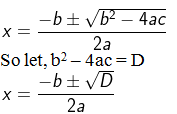D = b2 – 4ac

= (-7)2 – 4(2) (6)

= 49 – 48

= 1

So,

x = [-(-7) ± √1] / 2(2)

= [7 + 1]/ 4 or [7 – 1]/4

= 8/4 or 6/4

= 2 or 3/2

∴ Value of x = 2, 3/2

(ii) 2x² – 6x + 3 = 0

Let us consider,

a = 2, b = -6, c = 3

So, by using the formula,D = b2 – 4ac

= (-6)2 – 4(2) (3)

= 36 – 24

= 12

So,

x = [-(-6) ± √12] / 2(2)

= [6 ± 2√3] / 4

= [6 + 23]/ 4 or [6 – 23]/4

= 2(3 + 3)/4 or 2(3 – 3)/4

= (3 + 3)/2 or (3 – 3)/2

∴ Value of x = (3 + 3)/2, (3 – 3)/2

2. (i) x2 + 7x – 7 = 0

(ii) (2x + 3) (3x – 2) + 2 = 0

Solution:

(i) x2 + 7x – 7 = 0

Let us consider,

a = 1, b = 7, c = -7

So, by using the formula,D = b2 – 4ac

= (7)2 – 4(1) (-7)

= 49 + 28

= 77

So,

x = [-(7) ± √77] / 2(1)

= [-7 ± √77] / 2

= [-7 + 77]/ 2 or [-7 – 77]/2

∴ Value of x = [-7 + 77]/ 2, [-7 – 77]/2

(ii) (2x + 3) (3x – 2) + 2 = 0

Let us expand the expression,

6x2 – 4x + 9x – 6 + 2 = 0

6x2 + 5x – 4 = 0

Let us consider,

a = 6, b = 5, c = -4

So, by using the formula,D = b2 – 4ac

= (5)2 – 4(6) (-4)

= 25 + 96

= 121

So,

x = [-(5) ± √121] / 2(6)

= [-5 ± 11] / 12

= [-5 + 11]/ 12 or [-5 – 11]/12

= 6/12 or -16/12

= ½ or -4/3

∴ Value of x = ½, -4/3

3. (i) 256x² – 32x + 1 = 0
(ii) 25x² + 30x + 7 = 0
Solution:

(i) 256x² – 32x + 1 = 0

Let us consider,

a = 256, b = -32, c = 1

So, by using the formula,D = b2 – 4ac

= (-32)2 – 4(256) (1)

= 1024 – 1024

= 0

So,

x = [-(-32) ± √0] / 2(256)

=  / 512

= 1/16

∴ Value of x = 1/16

(ii) 25x² + 30x + 7 = 0

Let us consider,

a = 25, b = 30, c = 7

So, by using the formula,D = b2 – 4ac

= (30)2 – 4(25) (7)

= 900 – 700

= 200

So,

x = [-(30) ± √200] / 2(25)

= [-30 ± (100×2)]/ 50

= [-30 ± 102]/ 50

= [-3 ± 2]/ 5

= [-3 + 2)]/ 5 or [-3 – 2]/ 5

∴ Value of x = [-3 + 2)]/ 5, [-3 – 2]/ 5

4. (i) 2x² + √5x – 5 = 0
(ii) √3x² + 10x – 8√3 = 0
Solution:

(i) 2x² + √5x – 5 = 0

Let us consider,

a = 2, b = √5, c = -5

So, by using the formula,D = b2 – 4ac

= (√5)2 – 4(2) (-5)

= 5 + 40

= 45

So,

x = [-(√5) ± √45] / 2(2)

= [-√5 ± 35)]/ 4

= [-√5 + 35)]/ 4 or [-√5 – 35]/ 4

= 2√5/4 or -4√5/4

= √5/2 or -√5

∴ Value of x = √5/2, -√5

(ii) √3x² + 10x – 8√3 = 0

Let us consider,

a = √3, b = 10, c = -8√3

So, by using the formula,D = b2 – 4ac

= (10)2 – 4(√3) (-8√3)

= 100 + 96

= 196

So,

x = [-(10) ± √196] / 2(√3)

= [-10 ± 14] / 2(√3)

= [-10 + 14)]/ 2√3 or [-10 – 14)]/ 2√3

= 4/2√3 or -24/2√3

∴ Value of x = 4/2√3, -24/2√3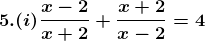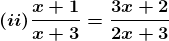Solution: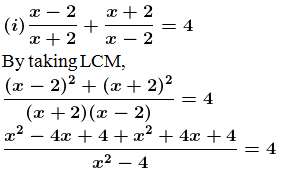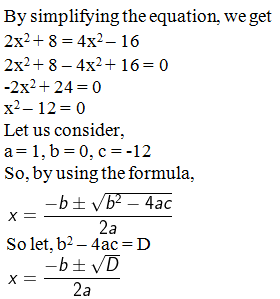D = b2 – 4ac

= (0)2 – 4(1) (-12)

= 0 + 48

= 48

So,

x = [-(0) ± √48] / 2(1)

= [±√48] / 2

= [±√(16×3)]/2

= ±4√3/2

= ±2√3

= 2√3 or -2√3

∴ Value of x = 2√3, -2√3Let us cross multiply, we get

(x + 1) (2x + 3) = (x + 3) (3x + 2)

Now by simplifying we get

2x2 + 3x + 2x + 3 = 3x2 + 9x + 2x + 6

2x2 + 5x + 3 – 3x2 – 11x – 6 = 0

-x2 – 6x – 3 = 0

x2 + 6x + 3 = 0

Let us consider,

a = 1, b = 6, c = 3

So, by using the formula,D = b2 – 4ac

= (6)2 – 4(1) (3)

= 36 -12

= 24

So,

x = [-(6) ± √24] / 2(1)

= [-6 ± √(4×6)] / 2

= [-6 ± 2√6]/2

= -3 ± √6

= -3 + √6 or -3 – √6

∴ Value of x = -3 + √6, -3 – √6

6. (i) a (x² + 1) = (a² + 1) x, a ≠ 0
(ii) 4x² – 4ax + (a² – b²) = 0
Solution:

(i) a (x² + 1) = (a² + 1) x, a ≠ 0

Let us simplify the expression,

ax2 + a – a2x + x = 0

ax2 – (a2 + 1)x + a = 0

Let us consider,

a = a, b = -(a2 + 1), c = a

So, by using the formula,D = b2 – 4ac

= (-(a2 + 1))2 – 4(a) (a)

= a4 + 2a2 + 1 – 4a2

= a4 – 2a2 + 1

= (a2 – 1)2

So,

x = [-(-(a2 + 1)) ± √(a2 – 1)2] / 2(a)

= [(a2 + 1) ± (a2 – 1)] / 2a

= [(a2 + 1) + (a2 – 1)] / 2a or [(a2 + 1) (a2 – 1)] / 2a

= [a2 + 1 + a2 – 1]/2a or [a2 + 1 – a2 + 1]/2a

= 2a2/2a or 2/2a

= a or 1/a

∴ Value of x = a, 1/a

(ii) 4x² – 4ax + (a² – b²) = 0

Let us consider,

a = 4, b = -4a, c = (a2 – b2)

So, by using the formula,D = b2 – 4ac

= (-4a)2 – 4(4) (a2 – b2)

= 16a2 – 16(a2 – b2)

= 16a2 – 16a2 + 16b2

= 16b2

So,

x = [-(-4a) ± √16b2] / 2(4)

= [4a ± 4b] / 8

= 4[a ± b] / 8

= [a ± b] / 2

= [a + b] / 2 or [a b] / 2

∴ Value of x = [a + b] / 2, [a b] / 2

7. (i) x−1/x = 3, x ≠ 0
(ii) 1/ x + 1/(x−2) = 3, x ≠ 0, 2
Solution:

(i) x−1/x = 3, x ≠ 0

Let us simplify the given expression,

By taking LCM

x2 – 1 = 3x

x2 – 3x – 1 = 0

Let us consider,

a = 1, b = -3, c = -1

So, by using the formula,D = b2 – 4ac

= (-3)2 – 4(1) (-1)

= 9 + 4

= 13

So,

x = [-(-3) ± √13] / 2(1)

= [3 ± √13] / 2

= [3 + √13] / 2 or [3 – √13] / 2

∴ Value of x = [3 + √13] / 2 or [3 – √13] / 2

(ii) 1/ x + 1/(x−2) = 3, x ≠ 0, 2

Let us simplify the given expression,

By taking LCM

[(x – 2) + x] / [x(x – 2)] = 3

[x – 2 + x] / [x2 – 2x] = 3

2x – 2 = 3(x2 – 2x)

2x – 2 = 3x2 – 6x

3x2 – 6x – 2x + 2 = 0

3x2 – 8x + 2

Let us consider,

a = 3, b = -8, c = 2

So, by using the formula,D = b2 – 4ac

= (-8)2 – 4(3) (2)

= 64 – 24

= 40

So,

x = [-(-8) ± √40] / 2(3)

= [8 ± 210] / 6

= 2[4 ± √10] / 6

= [4 ± √10] / 3

= [4 + √10] / 3 or [4 – √10] / 3

∴ Value of x = [4 + √10] / 3 or [4 – √10] / 3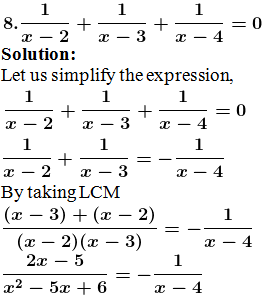By cross multiplying,

(2x – 5) (x – 4) = – (x2 – 5x + 6)

2x2 – 8x – 5x + 20 = -x2 + 5x – 6

2x2 – 8x – 5x + 20 + x2 – 5x + 6 = 0

3x2 – 18x + 26 = 0

Let us consider,

a = 3, b = -18, c = 26

So, by using the formula,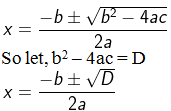D = b2 – 4ac

= (-18)2 – 4(3) (26)

= 324 – 312

= 12

So,

x = [-(-18) ± √12] / 2(3)

= [18 ± √12] / 6

= [18 + √12] / 6 or [18 – √12] / 6

∴ Value of x = [18 + √12] / 6 or [18 – √12] / 6

9. Solve for x: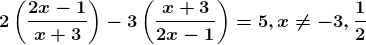Solution: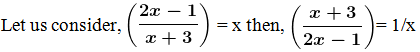So the equation becomes,

2x – 3/x = 5

By taking LCM

2x2 – 3 = 5x

2x2 – 5x – 3 = 0

Let us consider,

a = 2, b = -5, c = -3

So, by using the formula,D = b2 – 4ac

= (-5)2 – 4(2) (-3)

= 25 + 24

= 49

So,

x = [-(-5) ± √49] / 2(2)

= [5 ± 7] / 4

= [5 + 7] / 4 or [5 7] / 4

= /4 or [-2]/4

= 3 or -1/2

So, x = 3 or -1/2

Now,

Let us substitute in the equations,

When x = 3, then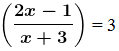By cross multiplying,

2x – 1 = 3x + 9

3x + 9 – 2x + 1 = 0

x + 10 = 0

x = -10

When x = -1/2, then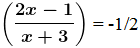By cross multiplying,

2(2x – 1) = -(x + 3)

4x – 2 = -x – 3

4x – 2 + x + 3 = 0

5x + 1 = 0

5x = -1

x = -1/5

∴ Value of x = -10, -1/5

10. Solve the following equation by using quadratic equations for x.
(i) x² – 5x – 10 = 0
(ii) 5x(x + 2) = 3
Solution:

(i) x² – 5x – 10 = 0

Let us consider,

a = 1, b = -5, c = -10

So, by using the formula,D = b2 – 4ac

= (-5)2 – 4(1) (-10)

= 25 + 40

= 65

So,

x = [-(-5) ± √65] / 2(1)

= [5 ± √65] / 2

= [5 ± 8.06] / 2

= [5 + 8.06] / 2 or [5 8.06] / 2

= [13.06]/2 or [-3.06]/2

= 6.53 or -1.53

∴ Value of x = 6.53 or -1.53

(ii) 5x(x + 2) = 3

Let us simplify the expression,

5x2 + 10x – 3 = 0

Let us consider,

a = 5, b = 10, c = -3

So, by using the formula,D = b2 – 4ac

= (10)2 – 4(5) (-3)

= 100 + 60

= 160

So,

x = [-(10) ± √160] / 2(5)

= [-10 ± 12.64] / 10

= [-10 + 12.64] / 10 or [-1012.64] / 10

= 2.64/10 or -22.64/10

= 0.264 or -2.264

∴ Value of x = 0.264 or -2.264

11. Solve the following equations by using quadratic formula and give your answer correct to 2 decimal places:
(i) 4x2 – 5x – 3 = 0
(ii) 2x – 1/x = 1
Solution:

(i) 4x2 – 5x – 3 = 0

Let us consider,

a = 4, b = -5, c = -3

So, by using the formula,D = b2 – 4ac

= (-5)2 – 4(4) (-3)

= 25 + 48

= 73

So,

x = [-(-5) ± √73] / 2(4)

= [5 ± 8.54] / 8

= [5 + 8.54] / 8 or [5 8.54] / 8

= 13.54/8 or -3.54/8

= 1.6925 or -0.4425

∴ Value of x = 1.69 or -0.44

(ii) 2x – 1/x = 7

By taking LCM

2x2 – 1 = 7x

2x2 – 7x – 1 = 0

Let us consider,

a = 2, b = -7, c = -1

So, by using the formula,D = b2 – 4ac

= (-7)2 – 4(2) (-1)

= 49 + 8

= 57

So,

x = [-(-7) ± √57] / 2(2)

= [7 ± 7.549] / 4

= [7 + 7.549] / 4 or [7 7.549] / 4

= 14.549/4 or -0.549/4

= 3.637 or -0.137

= 3.64 or -0.14

∴ Value of x = 3.64 or -0.14

12. Solve the following equation: x−18/x = 6. Give your answer correct to two x significant figures. (2011)

Solution:

Given equation:

x−18/x = 6

By taking LCM

x2 – 18 = 6x

x2 – 6x – 18 = 0

Let us consider,

a = 1, b = -6, c = -18

So, by using the formula,D = b2 – 4ac

= (-6)2 – 4(1) (-18)

= 36 + 72

= 108

So,

x = [-(-6) ± √108] / 2(1)

= [6 ± 10.39]/2

= [6 + 10.39]/2 or [6 10.39]/2

= [16.39]/2 or -4.39/2

= 8.19 or -2.19

∴ Value of x = 8.19 or -2.19

13. Solve the equation 5x² – 3x – 4 = 0 and give your answer correct to 3 significant figures:
Solution:

Given equation:

5x² – 3x – 4 = 0

Let us consider,

a = 5, b = -3, c = -4

So, by using the formula,D = b2 – 4ac

= (-3)2 – 4(5) (-4)

= 9 + 80

= 89

So,

x = [-(-3) ± √89] / 2(5)

= [3 ± 9.43] / 10

= [3 + 9.43] / 10 or [3 9.43] / 10

= 12.433/10 or -6.43/10

= 1.24 or -0.643

∴ Value of x = 1.24 or -0.643

EXERCISE 5.4

1. Find the discriminate of the following equations and hence find the nature of roots:
(i) 3x² – 5x – 2 = 0
(ii) 2x² – 3x + 5 = 0
(iii) 7x² + 8x + 2 = 0
(iv) 3x² + 2x – 1 = 0
(v) 16x² – 40x + 25 = 0
(vi) 2x² + 15x + 30 = 0
Solution:

(i) 3x² – 5x – 2 = 0

Let us consider,

a = 3, b = -5, c = -2

By using the formula,

D = b2 – 4ac

= (-5)2 – 4(3) (-2)

= 25 + 24

= 49

So,

Discriminate, D = 49

D > 0

∴ Roots are real and distinct.

(ii) 2x² – 3x + 5 = 0

Let us consider,

a = 2, b = -3, c = 5

By using the formula,

D = b2 – 4ac

= (-3)2 – 4(2) (5)

= 9 – 40

= -31

So,

Discriminate, D = -31

D < 0

∴ Roots are not real.

(iii) 7x² + 8x + 2 = 0

Let us consider,

a = 7, b = 8, c = 2

By using the formula,

D = b2 – 4ac

= (8)2 – 4(7) (2)

= 64 – 56

= 8

So,

Discriminate, D = 8

D > 0

∴ Roots are real and distinct.

(iv) 3x² + 2x – 1 = 0

Let us consider,

a = 3, b = 2, c = -1

By using the formula,

D = b2 – 4ac

= (2)2 – 4(3) (-1)

= 4 + 12

= 16

So,

Discriminate, D = 16

D > 0

∴ Roots are real and distinct.

(v) 16x² – 40x + 25 = 0

Let us consider,

a = 16, b = -40, c = 25

By using the formula,

D = b2 – 4ac

= (-40)2 – 4(16) (25)

= 1600 – 1600

= 0

So,

Discriminate, D = 0

D = 0

∴ Roots are real and equal.

(vi) 2x² + 15x + 30 = 0

Let us consider,

a = 2, b = 15, c = 30

By using the formula,

D = b2 – 4ac

= (15)2 – 4(2) (30)

= 225 – 240

= – 15

So,

Discriminate, D = -15

D < 0

∴ Roots are not real.

2. Discuss the nature of the roots of the following quadratic equations:
(i) x² – 4x – 1 = 0
(ii) 3x² – 2x + 1/3 = 0
(iii) 3x² – 4√3x + 4 = 0
(iv) x² – 1/2x + 4 = 0
(v) – 2x² + x + 1 = 0
(vi) 2√3x² – 5x + √3 = 0
Solution:

(i) x2 – 4x – 1 = 0

Let us consider,

a = 1, b = -4, c = -1

By using the formula,

D = b2 – 4ac

= (-4)2 – 4(1) (-1)

= 16 + 4

= 20

So,

Discriminate, D = 20

D > 0

∴ Roots are real and distinct.

(ii) 3x² – 2x + 1/3 = 0

Let us consider,

a = 3, b = -2, c = 1/3

By using the formula,

D = b2 – 4ac

= (-2)2 – 4(3) (1/3)

= 4 – 4

= 0

So,

Discriminate, D = 0

D = 0

∴ Roots are real and equal.

(iii) 3x² – 4√3x + 4 = 0

Let us consider,

a = 3, b = -4√3, c = 4

By using the formula,

D = b2 – 4ac

= (-4√3)2 – 4(3) (4)

= 16(3) – 48

= 48 – 48

= 0

So,

Discriminate, D = 0

D = 0

∴ Roots are real and equal.

(iv) x² – 1/2x + 4 = 0

Let us consider,

a = 1, b = -1/2, c = 4

By using the formula,

D = b2 – 4ac

= (-1/2)2 – 4(1) (4)

= 1/4 – 16

= -63/4

So,

Discriminate, D = -63/4

D < 0

∴ Roots are not real.

(v) – 2x² + x + 1 = 0

Let us consider,

a = -2, b = 1, c = 1

By using the formula,

D = b2 – 4ac

= (1)2 – 4(-2) (1)

= 1 + 8

= 9

So,

Discriminate, D = 9

D > 0

∴ Roots are real and distinct.

(vi) 2√3x² – 5x + √3 = 0

Let us consider,

a = 2√3, b = -5, c = √3

By using the formula,

D = b2 – 4ac

= (-5)2 – 4(2√3) (√3)

= 25 – 24

= 1

So,

Discriminate, D = 1

D > 0

∴ Roots are real and distinct.

3. Find the nature of the roots of the following quadratic equations:
(i) x² – 1/2x – 1/2 = 0
(ii) x² – 2√3x – 1 = 0 If real roots exist, find them.
Solution:

(i) x² – 1/2x – 1/2 = 0

Let us consider,

a = 1, b = -1/2, c = -1/2

By using the formula,

D = b2 – 4ac

= (-1/2)2 – 4(1) (-1/2)

= 1/4 + 2

= (1+8)/4

= 9/4

So,

Discriminate, D = 9/4

D > 0

∴ Roots are real and unequal.

(ii) x² – 2√3x – 1 = 0

Let us consider,

a = 1, b = 2√3, c = -1

By using the formula,

D = b2 – 4ac

= (2√3)2 – 4(1) (-1)

= 12 + 4

= 16

So,

Discriminate, D = 16

D > 0

∴ Roots are real and unequal.

4. Without solving the following quadratic equation, find the value of ‘p’ for which the given equations have real and equal roots:
(i) px² – 4x + 3 = 0
(ii) x² + (p – 3)x + p = 0
Solution:

(i) px² – 4x + 3 = 0

Let us consider,

a = p, b = -4, c = 3

By using the formula,

D = b2 – 4ac

= (-4)2 – 4(p) (3)

= 16 – 12p

Since, roots are real.

16 – 12p = 0

16 = 12p

p = 16/12

= 4/3

∴ p = 4/3

(ii) x² + (p – 3)x + p = 0

Let us consider,

a = 1, b = (p – 3), c = p

By using the formula,

D = b2 – 4ac

= (p – 3)2 – 4(1) (p)

= p2 – 32 – 2(3) (p) – 4p

= p2 + 9 – 6p – 4p

= p2 – 10p + 9

Since, roots are real and have equal roots.

p2 – 10p + 9 = 0

Now let us factorize,

p2 – 9p – p + 9 = 0

p(p – 9) – 1 (p – 9) = 0

(p – 9) (p – 1) = 0

So,

(p – 9) = 0 or (p – 1) = 0

p = 9 or p = 1

∴ p = 1, 9

5. Find the value (s) of k for which each of the following quadratic equation has equal roots:
(i) kx² – 4x – 5 = 0
(ii) (k – 4)x2 + 2(k – 4)x + 4 = 0
Solution:

(i) kx² – 4x – 5 = 0

Let us consider,

a = k, b = -4, c = -5

By using the formula,

D = b2 – 4ac

= (-4)2 – 4(k) (-5)

= 16 + 20k

Since, roots are equal.

16 + 20k = 0

20k = -16

k = -16/20

= -4/5

∴ k = -4/5

(ii) (k – 4)x² + 2(k – 4)x + 4 = 0

Let us consider,

a = (k – 4), b = 2(k – 4), c = 4

By using the formula,

D = b2 – 4ac

= (2(k – 4))2 – 4(k – 4) (4)

= (4(k2 + 16 – 8k)) – 16(k – 4)

= 4(k2 – 8k + 16) – 16k + 64

= 4 [k2 – 8k + 16 – 4k + 16]

= 4 [k2 – 12k + 32]

Since, roots are equal.

4 [k2 – 12k + 32] = 0

k2 – 12k + 32 = 0

Now let us factorize,

k2 – 8k – 4k + 32 = 0

k(k – 8) – 4 (k – 8) = 0

(k – 8) (k – 4) = 0

So,

(k – 8) = 0 or (k – 4) 0

k = 8 or k 4

∴ k = 8

6. Find the value(s) of m for which each of the following quadratic equation has real and equal roots:
(i) (3m + 1)x² + 2(m + 1)x + m = 0
(ii) x² + 2(m – 1) x + (m + 5) = 0
Solution:

(i) (3m + 1)x² + 2(m + 1)x + m = 0

Let us consider,

a = (3m + 1), b = 2(m + 1), c = m

By using the formula,

D = b2 – 4ac

= (2(m + 1))2 – 4 (3m + 1) (m)

= 4(m2 + 1 + 2m) – 4m(3m + 1)

= 4(m2 + 2m + 1) – 12m2 – 4m

= 4m2 + 8m + 4 – 12m2 – 4m

= -8m2 + 4m + 4

Since, roots are equal.

D = 0

-8m2 + 4m + 4 = 0

Divide by 4, we get

-2m2 + m + 1 = 0

2m2 – m – 1 = 0

Now let us factorize,

2m2 – 2m + m – 1 = 0

2m(m – 1) +1 (m – 1) = 0

(m – 1) (2m + 1) = 0

So,

(m – 1) = 0 or (2m + 1) = 0

m = 1 or 2m = -1

m = 1 or m = -1/2

∴ m = 1, -1/2

(ii) x² + 2(m – 1) x + (m + 5) = 0

Let us consider,

a = 1, b = 2(m – 1), c = (m + 5)

By using the formula,

D = b2 – 4ac

= (2(m – 1))2 – 4 (1) (m + 5)

= [4(m2 + 1 – 2m)] – 4m – 20

= 4m2 – 8m + 4 – 4m – 20

= 4m2 – 12m – 16

Since, roots are equal.

D = 0

4m2 – 12m – 16 = 0

Divide by 4, we get.

m2 – 3m – 4 = 0

Now let us factorize,

m2 – 4m + m – 4 = 0

m(m – 4) + 1 (m – 4) = 0

(m – 4) (m + 1) = 0

So,

(m – 4) = 0 or (m + 1) = 0

m = 4 or m = -1

∴ m = 4, -1

7. Find the values of k for which each of the following quadratic equation has equal roots:
(i) 9x² + kx + 1 = 0
(ii) x² – 2kx + 7k – 12 = 0
Also, find the roots for those values of k in each case.
Solution:

(i) 9x² + kx + 1 = 0

Let us consider,

a = 9, b = k, c = 1

By using the formula,

D = b2 – 4ac

= (k)2 – 4 (9) (1)

= k2 – 36

Since, roots are equal.

D = 0

k2 – 36 = 0

(k + 6) (k – 6) = 0

So,

(k + 6) = 0 or (k – 6) = 0

k = -6 or k = 6

∴ k = 6, -6

Now, let us substitute in the equation

When k = 6, then

9x² + kx + 1 = 0

9x2 + 6x + 1 = 0

(3x)2 + 2(3x)(1) + 12 = 0

(3x + 1)2 = 0

3x + 1 = 0

3x = -1

x = -1/3, -1/3

When k = -6, then

9x² + kx + 1 = 0

9x2 – 6x + 1 = 0

(3x)2 – 2(3x)(1) + 12 = 0

(3x – 1)2 = 0

3x – 1 = 0

3x = 1

x = 1/3, 1/3

(ii) x² – 2kx + 7k – 12 = 0

Let us consider,

a = 1, b = -2k, c = (7k – 12)

By using the formula,

D = b2 – 4ac

= (-2k)2 – 4 (1) (7k – 12)

= 4k2 – 28k + 48

Since, roots are equal.

D = 0

4k2 – 28k + 48 = 0

Divide by 4, we get

k2 – 7k + 12 = 0

Now let us factorize,

k2 – 3k – 4k + 12 = 0

k(k – 3) – 4 (k – 3) = 0

(k – 3) (k – 4) = 0

So,

(k – 3) = 0 or (k – 4) = 0

k = 3 or k = 4

∴ k = 3, 4

Now, let us substitute in the equation

When k = 3, then

By using the formula,= [-(-2k) ± √0] / 2(1)

= [2(3)]/2

= 3

x = 3, 3

When k = 4, then

By using the formula,= [-(-2k) ± √0] / 2(1)

= [2(4)] / 2

= 8/2

= 4

x = 4, 4

8. Find the value(s) of p for which the quadratic equation (2p + 1)x² – (7p + 2)x + (7p – 3) = 0 has equal roots. Also find these roots.
Solution:

Given:

(2p + 1)x² – (7p + 2)x + (7p – 3) = 0

Let us compare with ax2+ bx + c = 0

So we get,

a = (2p + 1), b = – (7p + 2), c = (7p – 3)

By using the formula,

D = b2 – 4ac

0 = (– (7p + 2))2 – 4 (2p + 1) (7p – 3)

= 49p2 + 4 + 28p – 4[14p2 – 6p + 7p – 3]

= 49p2 + 4 + 28p – 56p2 – 4p + 12

= -7p2 + 24p + 16

Let us factorize,

-7p2 + 28p – 4p + 16 = 0

-7p(p – 4) – 4 (p – 4) = 0

(p – 4) (-7p – 4) = 0

So,

(p – 4) = 0 or (-7p – 4) = 0

p = 4 or -7p = 4

p = 4 or p = -4/7

∴ Value of p = 4, -4/7

9. If – 5 is a root of the quadratic equation 2x² + px – 15 = 0 and the quadratic equation p(x² + x) + k = 0 has equal roots, find the value of k.
Solution:

Given:

– 5 is a root of the quadratic equation

2x² + px – 15 = 0, then

2(5)2 + p(-5) – 15 = 0

50 – 5p – 15 = 0

35 – 5p = 0

5p = 35

p = 35/5

= 7

p(x2 + x) + k = 0 has equal roots

px2 + px + k = 0

substitute the value of p,

7x2 + 7x + k = 0

Let us consider,

a = 7, b = 7, c = k

By using the formula,

D = b2 – 4ac

= (7)2 – 4 (7) (k)

= 49 – 28k

Since, roots are equal.

49 – 28k = 0

28k = 49

k = 49/28

= 7/4

∴ Value of k = 7/4

10. Find the value(s) of p for which the equation 2x² + 3x + p = 0 has real roots.
Solution:

Given:

2x² + 3x + p = 0

Let us consider,

a = 2, b = 3, c = p

By using the formula,

D = b2 – 4ac

= (3)2 – 4 (2) (p)

= 9 – 8p

Since, roots are real.

9 – 8p ≥ 0

9 ≥ 8p

8p ≤ 9

p ≤ 9/8

11. Find the least positive value of k for which the equation x² + kx + 4 = 0 has real roots.
Solution:

Given:

x² + kx + 4 = 0

Let us consider,

a = 1, b = k, c = 4

By using the formula,

D = b2 – 4ac

= (k)2 – 4 (1) (4)

= k2 – 16

Since, roots are real and positive.

k2 – 16 ≥ 0

k2 ≥ 16

k ≥ 4

k = 4

∴ Value of k = 4

12. Find the values of p for which the equation 3x² – px + 5 = 0 has real roots.
Solution:

Given:

3x² – px + 5 = 0

Let us consider,

a = 3, b = -p, c = 5

By using the formula,

D = b2 – 4ac

= (-p)2 – 4 (3) (5)

= p2 – 60

Since, roots are real.

p2 – 60 ≥ 0

p2 ≥ 60

p ≥ ± √60

p ≥ ± 215

p ≥ + 215 or p ≤ -215

∴ Value of p = 215, -215

EXERCISE 5.5

1. (i) Find two consecutive natural numbers such that the sum of their squares is 61.
(ii) Find two consecutive integers such that the sum of their squares is 61.
Solution:

(i) Find two consecutive natural numbers such that the sum of their squares is 61.

Let us consider first natural number be ‘x’

Second natural number be ‘x + 1’

So according to the question,

x2 + (x + 1)2 = 61

let us simplify the expression,

x2 + x2 + 12 + 2x – 61 = 0

2x2 + 2x – 60 = 0

Divide by 2, we get

x2 + x – 30 = 0

Let us factorize,

x2 + 6x – 5x – 30 = 0

x(x + 6) – 5 (x + 6) = 0

(x + 6) (x – 5) = 0

So,

(x + 6) = 0 or (x – 5) = 0

x = -6 or x = 5

∴ x = 5 [Since -6 is not a positive number]

Hence the first natural number = 5

Second natural number = 5 + 1 = 6

(ii) Find two consecutive integers such that the sum of their squares is 61.

Let us consider first integer number be ‘x’

Second integer number be ‘x + 1’

So according to the question,

x2 + (x + 1)2 = 61

let us simplify the expression,

x2 + x2 + 12 + 2x – 61 = 0

2x2 + 2x – 60 = 0

Divide by 2, we get

x2 + x – 30 = 0

Let us factorize,

x2 + 6x – 5x – 30 = 0

x(x + 6) – 5 (x + 6) = 0

(x + 6) (x – 5) = 0

So,

(x + 6) = 0 or (x – 5) = 0

x = -6 or x = 5

Now,

If x = -6, then

First integer number = -6

Second integer number = -6 + 1 = -5

If x = 5, then

First integer number = 5

Second integer number = 5 + 1 = 6

2. (i) If the product of two positive consecutive even integers is 288, find the integers.
(ii) If the product of two consecutive even integers is 224, find the integers.
(iii) Find two consecutive even natural numbers such that the sum of their squares is 340.
(iv) Find two consecutive odd integers such that the sum of their squares is 394.
Solution:

(i) If the product of two positive consecutive even integers is 288, find the integers.

Let us consider first positive even integer number be ‘2x’

Second even integer number be ‘2x + 2’

So according to the question,

2x × (2x + 2) = 288

4x2 + 4x – 288 = 0

Divide by 4, we get

x2 + x – 72 = 0

Let us factorize,

x2 + 9x – 8x – 72 = 0

x(x + 9) – 8(x + 9) = 0

(x + 9) (x – 8) = 0

So,

(x + 9) = 0 or (x – 8) = 0

x = -9 or x = 8

∴ Value of x = 8 [since, -9 is not positive]

First even integer = 2x = 2 (8) = 16

Second even integer = 2x + 2 = 2(8) + 2 = 18

(ii) If the product of two consecutive even integers is 224, find the integers.

Let us consider first positive even integer number be ‘2x’

Second even integer number be ‘2x + 2’

So according to the question,

2x × (2x + 2) = 224

4x2 + 4x – 224 = 0

Divide by 4, we get

x2 + x – 56 = 0

Let us factorize,

x2 + 8x – 7x – 56 = 0

x(x + 8) – 7(x + 8) = 0

(x + 8) (x – 7) = 0

So,

(x + 8) = 0 or (x – 7) = 0

x = -8 or x = 7

∴ Value of x = 7 [since, -8 is not positive]

First even integer = 2x = 2 (7) = 14

Second even integer = 2x + 2 = 2(7) + 2 = 16

(iii) Find two consecutive even natural numbers such that the sum of their squares is 340.

Let us consider first positive even natural number be ‘2x’

Second even number be ‘2x + 2’

So according to the question,

(2x)2 + (2x + 2)2 = 340

4x2 + 4x2 + 8x + 4 – 340 = 0

8x2 + 8x – 336 = 0

Divide by 8, we get

x2 + x – 42 = 0

Let us factorize,

x2 + 7x – 6x – 56 = 0

x(x + 7) – 6(x + 7) = 0

(x + 7) (x – 6) = 0

So,

(x + 7) = 0 or (x – 6) = 0

x = -7 or x = 6

∴ Value of x = 6 [since, -7 is not positive]

First even natural number = 2x = 2 (6) = 12

Second even natural number = 2x + 2 = 2(6) + 2 = 14

(iv) Find two consecutive odd integers such that the sum of their squares is 394.

Let us consider first odd integer number be ‘2x + 1’

Second odd integer number be ‘2x + 3’

So according to the question,

(2x + 1)2 + (2x + 3)2 = 394

4x2 + 4x + 1 + 4x2 + 12x + 9 – 394 = 0

8x2 + 16x – 384 = 0

Divide by 8, we get

x2 + 2x – 48 = 0

Let us factorize,

x2 + 8x – 6x – 48 = 0

x(x + 8) – 6(x + 8) = 0

(x + 8) (x – 6) = 0

So,

(x + 8) = 0 or (x – 6) = 0

x = -8 or x = 6

When x = -8, then

First odd integer = 2x + 1 = 2 (-8) + 1 = -16 + 1 = -15

Second odd integer = 2x + 3 = 2(-8) + 3 = -16 + 3 = -13

When x = 6, then

First odd integer = 2x + 1 = 2 (6) + 1 = 12 + 1 = 13

Second odd integer = 2x + 3 = 2(6) + 3 = 12 + 3 = 15

∴ The required odd integers are -15, -13, 13, 15.

3. The sum of two numbers is 9 and the sum of their squares is 41. Taking one number as x, form ail equation in x and solve it to find the numbers.
Solution:

Given:

Sum of two number = 9

Let us consider first number be ‘x’

Second number be ‘9 – x’

So according to the question,

(x)2 + (9 – x)2 = 41

x2 + 81 – 18x + x2 – 41 = 0

2x2 – 18x + 40 = 0

Divide by 2, we get

x2 – 9x + 20 = 0

Let us factorize,

x2 – 4x – 5x + 20 = 0

x(x – 4) – 5(x – 4) = 0

(x – 4) (x – 5) = 0

So,

(x – 4) = 0 or (x – 5) = 0

x = 4 or x = 5

When x = 4, then

First number = x = 4

Second number = 9 – x = 9 – 4 = 5

When x = 5, then

First number = x = 5

Second number = 9 – x = 9 – 5 = 4

∴ The required numbers are 4 and 5.

4. Five times a certain whole number is equal to three less than twice the square of the number. Find the number.
Solution:

Let us consider the number be ‘x’

So according to the question,

5x = 2x2 – 3

2x2 – 3 – 5x = 0

2x2 – 5x – 3 = 0

Let us factorize,

2x2 – 6x + x – 3 = 0

2x(x – 3) + 1(x – 3) = 0

(x – 3) (2x + 1) = 0

So,

(x – 3) = 0 or (2x + 1) = 0

x = 3 or 2x = -1

x = 3 or x = -1/2

∴ The required number is 3 [since, -1/2 cannot be a whole number].

5. Sum of two natural numbers is 8 and the difference of their reciprocals is 2/15. Find the numbers.
Solution:

Let us consider two numbers as ‘x’ and ‘y’

So according to the question,

1/x – 1/y = 2/15 ….. (i)

It is given that, x + y = 8

So, y = 8 – x … (ii)

Now, substitute the value of y in equation (i), we get

1/x – 1/(8 – x) 2/15

By taking LCM,

[8 – x – x] / x(8 – x) = 2/15

(8 – 2x) / x(8 – x) = 2/15

By cross multiplying,

15(8 – 2x) = 2x(8 – x)

120 – 30x = 16x – 2x2

120 – 30x – 16x + 2x2 = 0

2x2 – 46x + 120 = 0

Divide by 2, we get

x2 – 23x + 60 = 0

let us factorize,

x2 – 20x – 3x + 60 = 0

x(x – 20) – 3 (x – 20) = 0

(x – 20) (x – 3) = 0

So,

(x – 20) = 0 or (x – 3) = 0

x = 20 or x = 3

Now,

Sum of two natural numbers, y = 8 – x = 8 – 20 = -12, which is a negative value.

So value of x = 3, y = 8 – x = 8 – 3 = 5

∴ The value of x and y are 3 and 5.

6. The difference between the squares of two numbers is 45. The square of the smaller number is 4 times the larger number. Determine the numbers.
Solution:

Let us consider the larger number be ‘x’

Smaller number be ‘y’

So according to the question,

x2 – y2 = 45 … (i)

y2 = 4x … (ii)

Now substitute the value of y in equation (i), we get

x2 – 4x = 45

x2 – 4x – 45 = 0

let us factorize,

x2 – 9x + 5x – 45 = 0

x(x – 9) + 5 (x – 9) = 0

(x – 9) (x + 5) = 0

So,

(x – 9) = 0 or (x + 5) = 0

x = 9 or x = -5

When x = 9, then

The larger number = x = 9

Smaller number = y => y2 = 4x

y = 4x = 4(9) = 36 = 6

When x = -5, then

The larger number = x = -5

Smaller number = y => y2 = 4x

y = 4x = 4(-5) = -20 (which is not possible)

∴ The value of x and y are 9, 6.

7. There are three consecutive positive integers such that the sum of the square of the first and the product of the other two is 154. What are the integers?
Solution:

Let us consider the first integer be ‘x’

Second integer be ‘x + 1’

Third integer be ‘x + 2’

So, according to the question,

x2 + (x + 1) (x + 2) = 154

let us simplify,

x2 + x2 + 3x + 2 – 154 = 0

2x2 + 3x – 152 = 0

Let us factorize,

2x2 + 19x – 16x – 152 = 0

x(2x + 19) – 8 (2x + 19) = 0

(2x + 19) (x – 8) = 0

So,

(2x + 19) = 0 or (x – 8) = 0

2x = -19 or x = 8

x = -19/2 or x = 8

∴ The value of x = 8 [since -19/2 is a negative value]

So,

First integer = x = 8

Second integer = x + 1 = 8 + 1 = 9

Third integer = x + 2 = 8 + 2 = 10

∴ The numbers are 8, 9, 10.

8. (i) Find three successive even natural numbers, the sum of whose squares is 308.
(ii) Find three consecutive odd integers, the sum of whose squares is 83.
Solution:

(i) Find three successive even natural numbers, the sum of whose squares is 308.

Let us consider first even natural number be ‘2x’

Second even number be ‘2x + 2’

Third even number be ‘2x + 4’

So according to the question,

(2x)2 + (2x + 2)2 + (2x + 4)2 = 308

4x2 + 4x2 + 8x + 4 + 4x2 + 16x + 16 – 308 = 0

12x2 + 24x – 288 = 0

Divide by 12, we get

x2 + 2x – 24 = 0

Let us factorize,

x2 + 6x – 4x – 24 = 0

x(x + 6) – 4(x + 6) = 0

(x + 6) (x – 4) = 0

So,

(x + 6) = 0 or (x – 4) = 0

x = -6 or x = 4

∴ Value of x = 4 [since, -6 is not positive]

First even natural number = 2x = 2 (4) = 8

Second even natural number = 2x + 2 = 2(4) + 2 = 10

Third even natural number = 2x + 4 = 2(4) + 4 = 12

∴ The numbers are 8, 10, 12.

(ii) Find three consecutive odd integers, the sum of whose squares is 83.

Let the three numbers be ‘x’, ‘x + 2’, ‘x + 4’

So according to the question,

(x)2 + (x + 2)2 + (x + 4)2 = 83

x2 + x2 + 4x + 4 + x2 + 8x + 16 – 83 = 0

3x2 + 12x – 63 = 0

Divide by 3, we get

x2 + 4x – 21 = 0

let us factorize,

x2 + 7x – 3x – 21 = 0

x(x + 7) – 3 (x + 7) = 0

(x + 7) (x – 3) = 0

So,

(x + 7) = 0 or (x – 3) = 0

x = -7 or x = 3

∴ The numbers will be x, x+2, x+4 => -7, -7+2, -7+4 => -7, -5, -3

Or the numbers will be x, x+2, x+4 => 3, 3+2, 3+4, => 3, 5, 7

9. In a certain positive fraction, the denominator is greater than the numerator by 3. If 1 is subtracted from both the numerator and denominator, the fraction is decreased by 1/14. Find the fraction.
Solution:

Let the numerator be ‘x’

Denominator be ‘x+3’

So the fraction is x/(x+3)

According to the question,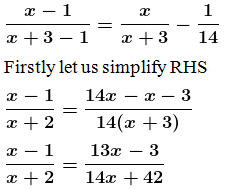By cross multiplying, we get

(x – 1) (14x + 42) = (x + 2) (13x – 3)

14x2 + 42x – 14x – 42 = 13x2 – 3x + 26x – 6

14x2 + 42x – 14x – 42 – 13x2 + 3x – 26x + 6 = 0

x2 + 5x – 36 = 0

let us factorize,

x2 + 9x – 4x – 36 = 0

x(x + 9) – 4 (x + 9) = 0

(x + 9) (x – 4) = 0

So,

(x + 9) = 0 or (x – 4) = 0

x = -9 or x = 4

So the value of x = 4 [since, -9 is a negative number]

When substitute the value of x = 4 in the fraction x/(x+3), we get

4/(4+3) = 4/7

∴ The required fraction is = 4/7

10. The sum of the numerator and denominator of a certain positive fraction is 8. If 2 is added to both the numerator and denominator, the fraction is increased by 4/35. Find the fraction.
Solution:

Let the denominator be ‘x’

So the numerator will be ‘8-x’

The obtained fraction is (8-x)/x

So according to the question,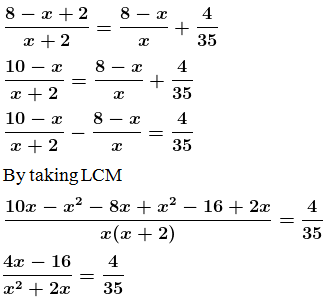By cross multiplying,

35(4x – 16) = 4(x2 + 2x)

140x – 560 = 4x2 + 8x

4x2 + 8x – 140x + 560 = 0

4x2 – 132x + 560 = 0

Divide by 4, we get

x2 – 33x + 140 = 0

let us factorize,

x2 – 28x – 5x + 140 = 0

x(x – 28) – 5 (x – 28) = 0

(x – 28) (x – 5) = 0

So,

(x – 28) = 0 or (x – 5) = 0

x = 28 or x = 5

So the value of x = 5 [since, x = 28 is not possible as sum of numerator and denominator is 8]

When substitute the value of x = 5 in the fraction (8-x)/x, we get

(8 – 5)/5 = 3/5

∴ The required fraction is = 3/5

11. A two digit number contains the bigger at ten’s place. The product of the digits is 27 and the difference between two digits is 6. Find the number.
Solution:

Let us consider unit’s digit be ‘x’

Ten’s digit = x+6

Number = x + 10(x+6)

= x + 10x + 60

= 11x + 60

So according the question,

x(x + 6) = 27

x2 + 6x – 27 = 0

let us factorize,

x2 + 9x – 3x – 27 = 0

x(x + 9) – 3 (x + 9) = 0

(x + 9) (x – 3) = 0

So,

(x + 9) = 0 or (x – 3) = 0

x = -9 or x = 3

so, value of x = 3 [since, -9 is a negative number]

∴ The number = 11x + 60

= 11(3) + 60

= 33 + 60

= 93

12. A two digit positive number is such that the product of its digits is 6. If 9 is added to the number, the digits interchange their places. Find the number. (2014)
Solution:

Let us consider 2-digit number be ‘xy’ = 10x + y

Reversed digits = yx = 10y + x

So according to the question,

10x + y + 9 = 10y + x

It is given that,

xy = 6

y = 6/x

so, by substituting the value in above equation, we get

10x + 6/x + 9 = 10(6/x) + x

By taking LCM,

10x2 + 6 + 9x = 60 + x2

10x2 + 6 + 9x – 60 – x2 = 0

9x2 + 9x – 54 = 0

Divide by 9, we get

x2 + x – 6 = 0

let us factorize,

x2 + 3x – 2x – 6 = 0

x(x + 3) – 2 (x + 3) = 0

(x + 3) (x – 2) = 0

So,

(x + 3) = 0 or (x – 2) = 0

x = -3 or x = 2

Value of x = 2 [since, -3 is a negative value]

Now, substitute the value of x in y = 6/x, we get

y = 6/2 = 3

∴ 2-digit number = 10x + y = 10(2) + 3 = 23

13. A rectangle of area 105 cm² has its length equal to x cm. Write down its breadth in terms of x. Given that the perimeter is 44 cm, write down an equation in x and solve it to determine the dimensions of the rectangle.
Solution:

Given:

Perimeter of rectangle = 44cm

Length + breadth = 44/2 = 22cm

Let us consider length be ‘x’

So according to the question,

x(22 – x) = 105

22x – x2 – 105 = 0

x2 – 22x + 105 = 0

let us factorize,

x2 – 15x – 7x + 105 = 0

x(x – 15) – 7(x – 15) = 0

(x – 15) (x – 7) = 0

So,

(x – 15) = 0 or (x – 7) = 0

x = 15 or x = 7

∴ Length = 15cm

Breadth = 22 – x = 22 – 15 = 7cm

14. A rectangular garden 10 m by 16 m is to be surrounded by a concrete walk of uniform width. Given that the area of the walk is 120 square meters, assuming the width of the walk to be x, form an equation in x and solve it to find the value of x. (1992)
Solution:

Given:

Length of garden = 16cm

Width = 10cm

Let the width of walk be ‘x’ meter

Outer length = 16 + 2x

Outer width = 10 + 2x

So according to the question,

(16 + 2x) (10 + 2x) – 16(10) = 120

160 + 32x + 20x + 4x2 – 160 – 120 = 0

4x2 + 52x – 120 = 0

Divide by 4, we get

x2 + 13x – 30 = 0

x2 + 15x – 2x – 30 = 0

x(x + 15) – 2 (x + 15) = 0

(x + 15) (x – 2) = 0

So,

(x + 15) = 0 or (x – 2) = 0

x = -15 or x = 2

∴ Value of x is 2 [Since, -15 is a negative value]

15. (i) Harish made a rectangular garden, with its length 5 meters more than its width. The next year, he increased the length by 3 meters and decreased the width by 2 meters. If the area of the second garden was 119 sqm, was the second garden larger or smaller?
(ii) The length of a rectangle exceeds its breadth by 5 m. If the breadth was doubled and the length reduced by 9 m, the area of the rectangle would have increased by 140 m². Find its dimensions.
Solution:

(i) In first case:

Let us consider length of the garden be ‘x’ meter

Width = (x – 5) meter

Area = lb

= x(x – 5) sq.m

In second case:

Length = (x + 3) meter

Width = x – 5 – 2 = (x – 7) meter

So according to the question,

(x + 3) (x – 7) = 119

x2 – 7x + 3x – 21 – 119 = 0

x2 – 4x – 140 = 0

Let us factorize,

x2 – 14x + 10x – 140 = 0

x(x – 14) + 10 (x – 14) = 0

(x – 14) (x + 10) = 0

So,

(x – 14) = 0 or (x + 10) = 0

x = 14 or x = -10

∴ Length of the first garden = 14meters. [Since, -10 is a negative value]

Width = x – 5 = 14 – 5 = 9meters

Area = lb

= 14 × 9 = 126 m2

Difference of area of two rectangles = 126 – 119 = 7 sq.m

Area of second garden is smaller than the area of the first garden by 7 sq.m.

(ii) In first case:

Let us consider length of the rectangle be ‘x’ meter

Width = (x – 5) meter

Area = lb

= x(x – 5) sq.m

In second case:

Length = (x – 9) meter

Width = 2 (x – 5) meter

Area = (x – 9) 2(x – 5) = 2(x – 9) (x – 5) sq.m

So according to the question,

2(x – 9) (x – 5) = x(x – 5) + 140

2(x2 – 14x + 45) = x2 – 5x + 140

2x2 – 28x + 90 – x2 + 5x – 140 = 0

x2 – 23x – 50 = 0

let us factorize,

x2 – 25x + 2x – 50 = 0

x(x – 25) + 2 (x – 25) = 0

(x – 25) (x + 2) = 0

So,

(x – 25)= 0 or (x + 2) = 0

x = 25 or x = -2

∴ Length of the first rectangle = 25meters. [Since, -2 is a negative value]

Width = x – 5 = 25 – 5 = 20meters

Area = lb

= 25 × 20 = 500 m2